US6867922B1 - Projection optical system and projection exposure apparatus using the same - Google Patents

Projection optical system and projection exposure apparatus using the same Download PDF

Info

Publication number
US6867922B1
US6867922B1 US09/593,584 US59358400A US6867922B1 US 6867922 B1 US6867922 B1 US 6867922B1 US 59358400 A US59358400 A US 59358400A US 6867922 B1 US6867922 B1 US 6867922B1
Authority
US
United States
Prior art keywords
lens
optical system
lens group
aspherical
projection optical
Prior art date
Legal status (The legal status is an assumption and is not a legal conclusion. Google has not performed a legal analysis and makes no representation as to the accuracy of the status listed.)
Active, expires
Application number
US09/593,584
Inventor
Chiaki Terasawa
Hiroyuki Ishii
Takashi Kato
Current Assignee (The listed assignees may be inaccurate. Google has not performed a legal analysis and makes no representation or warranty as to the accuracy of the list.)
Canon Inc
Original Assignee
Canon Inc
Priority date (The priority date is an assumption and is not a legal conclusion. Google has not performed a legal analysis and makes no representation as to the accuracy of the date listed.)
Filing date
Publication date
Priority to JP11167422A priority Critical patent/JP2000352667A/en
Priority to JP16742799A priority patent/JP3376318B2/en
Priority to JP16742199A priority patent/JP3423644B2/en
Priority to JP11167426A priority patent/JP2000356742A/en
Application filed by Canon Inc filed Critical Canon Inc
Assigned to CANON KABUSHIKI KAISHA reassignment CANON KABUSHIKI KAISHA ASSIGNMENT OF ASSIGNORS INTEREST (SEE DOCUMENT FOR DETAILS). Assignors: ISHII, HIROYUKI, KATO, TAKASHI, TERASAWA, CHIAKI
Publication of US6867922B1 publication Critical patent/US6867922B1/en
Application granted granted Critical
Application status is Active legal-status Critical
Adjusted expiration legal-status Critical

• 101700065210 ASP1 family Proteins 0 description 2
• 101700060050 ASP3 family Proteins 0 description 6
• 101700078948 ASP4 family Proteins 0 description 2
• 102100003265 ASPH Human genes 0 description 1
• 101700044969 ASPH family Proteins 0 description 1
• 102100015650 BACE1 Human genes 0 description 7
• 101700051112 BACE1 family Proteins 0 description 7
• 102100015648 BACE2 Human genes 0 description 2
• 101700051947 BACE2 family Proteins 0 description 2
• WUKWITHWXAAZEY-UHFFFAOYSA-L Calcium fluoride Chemical compound data:image/svg+xml;base64,PD94bWwgdmVyc2lvbj0nMS4wJyBlbmNvZGluZz0naXNvLTg4NTktMSc/Pgo8c3ZnIHZlcnNpb249JzEuMScgYmFzZVByb2ZpbGU9J2Z1bGwnCiAgICAgICAgICAgICAgeG1sbnM9J2h0dHA6Ly93d3cudzMub3JnLzIwMDAvc3ZnJwogICAgICAgICAgICAgICAgICAgICAgeG1sbnM6cmRraXQ9J2h0dHA6Ly93d3cucmRraXQub3JnL3htbCcKICAgICAgICAgICAgICAgICAgICAgIHhtbG5zOnhsaW5rPSdodHRwOi8vd3d3LnczLm9yZy8xOTk5L3hsaW5rJwogICAgICAgICAgICAgICAgICB4bWw6c3BhY2U9J3ByZXNlcnZlJwp3aWR0aD0nMzAwcHgnIGhlaWdodD0nMzAwcHgnID4KPCEtLSBFTkQgT0YgSEVBREVSIC0tPgo8cmVjdCBzdHlsZT0nb3BhY2l0eToxLjA7ZmlsbDojRkZGRkZGO3N0cm9rZTpub25lJyB3aWR0aD0nMzAwJyBoZWlnaHQ9JzMwMCcgeD0nMCcgeT0nMCc+IDwvcmVjdD4KPHRleHQgeD0nNTAuMDI1OCcgeT0nMTU4LjI1JyBzdHlsZT0nZm9udC1zaXplOjE1cHg7Zm9udC1zdHlsZTpub3JtYWw7Zm9udC13ZWlnaHQ6bm9ybWFsO2ZpbGwtb3BhY2l0eToxO3N0cm9rZTpub25lO2ZvbnQtZmFtaWx5OnNhbnMtc2VyaWY7dGV4dC1hbmNob3I6c3RhcnQ7ZmlsbDojMzNDQ0NDJyA+PHRzcGFuPkY8L3RzcGFuPjx0c3BhbiBzdHlsZT0nYmFzZWxpbmUtc2hpZnQ6c3VwZXI7Zm9udC1zaXplOjExLjI1cHg7Jz4tPC90c3Bhbj48dHNwYW4+PC90c3Bhbj48L3RleHQ+Cjx0ZXh0IHg9JzIzNS45NzQnIHk9JzE1OC4yNScgc3R5bGU9J2ZvbnQtc2l6ZToxNXB4O2ZvbnQtc3R5bGU6bm9ybWFsO2ZvbnQtd2VpZ2h0Om5vcm1hbDtmaWxsLW9wYWNpdHk6MTtzdHJva2U6bm9uZTtmb250LWZhbWlseTpzYW5zLXNlcmlmO3RleHQtYW5jaG9yOnN0YXJ0O2ZpbGw6IzMzQ0NDQycgPjx0c3Bhbj5GPC90c3Bhbj48dHNwYW4gc3R5bGU9J2Jhc2VsaW5lLXNoaWZ0OnN1cGVyO2ZvbnQtc2l6ZToxMS4yNXB4Oyc+LTwvdHNwYW4+PHRzcGFuPjwvdHNwYW4+PC90ZXh0Pgo8L3N2Zz4K data:image/svg+xml;base64,PD94bWwgdmVyc2lvbj0nMS4wJyBlbmNvZGluZz0naXNvLTg4NTktMSc/Pgo8c3ZnIHZlcnNpb249JzEuMScgYmFzZVByb2ZpbGU9J2Z1bGwnCiAgICAgICAgICAgICAgeG1sbnM9J2h0dHA6Ly93d3cudzMub3JnLzIwMDAvc3ZnJwogICAgICAgICAgICAgICAgICAgICAgeG1sbnM6cmRraXQ9J2h0dHA6Ly93d3cucmRraXQub3JnL3htbCcKICAgICAgICAgICAgICAgICAgICAgIHhtbG5zOnhsaW5rPSdodHRwOi8vd3d3LnczLm9yZy8xOTk5L3hsaW5rJwogICAgICAgICAgICAgICAgICB4bWw6c3BhY2U9J3ByZXNlcnZlJwp3aWR0aD0nODVweCcgaGVpZ2h0PSc4NXB4JyA+CjwhLS0gRU5EIE9GIEhFQURFUiAtLT4KPHJlY3Qgc3R5bGU9J29wYWNpdHk6MS4wO2ZpbGw6I0ZGRkZGRjtzdHJva2U6bm9uZScgd2lkdGg9Jzg1JyBoZWlnaHQ9Jzg1JyB4PScwJyB5PScwJz4gPC9yZWN0Pgo8dGV4dCB4PSc4LjY1NzA4JyB5PSc1MC4yNScgc3R5bGU9J2ZvbnQtc2l6ZToxNXB4O2ZvbnQtc3R5bGU6bm9ybWFsO2ZvbnQtd2VpZ2h0Om5vcm1hbDtmaWxsLW9wYWNpdHk6MTtzdHJva2U6bm9uZTtmb250LWZhbWlseTpzYW5zLXNlcmlmO3RleHQtYW5jaG9yOnN0YXJ0O2ZpbGw6IzMzQ0NDQycgPjx0c3Bhbj5GPC90c3Bhbj48dHNwYW4gc3R5bGU9J2Jhc2VsaW5lLXNoaWZ0OnN1cGVyO2ZvbnQtc2l6ZToxMS4yNXB4Oyc+LTwvdHNwYW4+PHRzcGFuPjwvdHNwYW4+PC90ZXh0Pgo8dGV4dCB4PSc2MS4zNDIzJyB5PSc1MC4yNScgc3R5bGU9J2ZvbnQtc2l6ZToxNXB4O2ZvbnQtc3R5bGU6bm9ybWFsO2ZvbnQtd2VpZ2h0Om5vcm1hbDtmaWxsLW9wYWNpdHk6MTtzdHJva2U6bm9uZTtmb250LWZhbWlseTpzYW5zLXNlcmlmO3RleHQtYW5jaG9yOnN0YXJ0O2ZpbGw6IzMzQ0NDQycgPjx0c3Bhbj5GPC90c3Bhbj48dHNwYW4gc3R5bGU9J2Jhc2VsaW5lLXNoaWZ0OnN1cGVyO2ZvbnQtc2l6ZToxMS4yNXB4Oyc+LTwvdHNwYW4+PHRzcGFuPjwvdHNwYW4+PC90ZXh0Pgo8L3N2Zz4K [F-].[F-].[Ca+2] WUKWITHWXAAZEY-UHFFFAOYSA-L 0 description 11
• 206010010071 Coma Diseases 0 description 45
• 210000000887 Face Anatomy 0 description 6
• 102100015302 NAPSA Human genes 0 description 2
• 101700082129 NAPSA family Proteins 0 description 2
• 206010073261 Ovarian theca cell tumour Diseases 0 description 4
• 210000001747 Pupil Anatomy 0 description 1
• 208000001644 Thecoma Diseases 0 description 4
• 239000003570 air Substances 0 description 1
• 230000004075 alteration Effects 0 description 400
• 201000009310 astigmatism Diseases 0 description 42
• 230000015556 catabolic process Effects 0 description 1
• 239000000562 conjugates Substances 0 abstract claims description 43
• 230000001276 controlling effects Effects 0 description 3
• 230000000875 corresponding Effects 0 description 11
• 230000003247 decreasing Effects 0 description 1
• 238000006731 degradation Methods 0 description 1
• 230000004059 degradation Effects 0 description 1
• 230000003292 diminished Effects 0 description 1
• 238000006073 displacement Methods 0 description 1
• 230000000694 effects Effects 0 description 14
• 238000005530 etching Methods 0 description 2
• 239000010408 films Substances 0 description 1
• 239000010436 fluorite Substances 0 description 11
• 239000002529 flux Substances 0 description 14
• 239000005350 fused silica glasses Substances 0 description 1
• 239000011521 glass Substances 0 description 5
• 238000000227 grinding Methods 0 description 1
• 125000001475 halogen functional group Chemical group 0 description 3
• 238000005286 illumination Methods 0 claims description 12
• 238000003384 imaging method Methods 0 description 15
• 230000001976 improved Effects 0 description 6
• 230000001965 increased Effects 0 description 6
• 238000007689 inspection Methods 0 description 6
• 150000002500 ions Chemical class 0 description 2
• 239000011133 lead Substances 0 description 3
• 239000004973 liquid crystal related substances Substances 0 description 3
• 239000007788 liquids Substances 0 description 4
• 238000001459 lithography Methods 0 description 5
• 238000003754 machining Methods 0 description 3
• 238000004519 manufacturing process Methods 0 claims description 10
• 239000000463 materials Substances 0 description 10
• 239000002184 metal Substances 0 description 2
• 229910052751 metals Inorganic materials 0 description 2
• 238000000034 methods Methods 0 description 32
• 239000000203 mixtures Substances 0 description 3
• 230000004048 modification Effects 0 description 1
• 238000006011 modification Methods 0 description 1
• 238000009740 moulding (composite fabrication) Methods 0 description 4
• 229910052757 nitrogen Inorganic materials 0 description 2
• 230000003287 optical Effects 0 abstract claims description title 392
• 238000005457 optimization Methods 0 description 1
• 230000003647 oxidation Effects 0 description 1
• 238000007254 oxidation reaction Methods 0 description 1
• 230000001590 oxidative Effects 0 description 1
• 239000002245 particles Substances 0 description 2
• 230000002093 peripheral Effects 0 abstract claims description 59
• 238000005498 polishing Methods 0 description 5
• 238000007639 printing Methods 0 description 3
• 238000003672 processing method Methods 0 description 2
• 238000005365 production Methods 0 description 10
• 239000000047 products Substances 0 description 5
• 229910052904 quartz Inorganic materials 0 description 1
• 239000010453 quartz Substances 0 description 1
• 230000002829 reduced Effects 0 description 9
• 230000001603 reducing Effects 0 description 8
• 238000006722 reduction reaction Methods 0 description 5
• 238000007789 sealing Methods 0 description 1
• 239000004065 semiconductor Substances 0 description 14
• 238000000926 separation method Methods 0 description 1
• 238000004904 shortening Methods 0 description 4
• 239000010703 silicon Substances 0 description 2
• 229910052710 silicon Inorganic materials 0 description 2
• 229910001885 silicon dioxide Inorganic materials 0 description 6
• 239000000377 silicon dioxide Substances 0 description 5
• 239000007787 solids Substances 0 description 1
• 239000000758 substrates Substances 0 description 9
• 230000001629 suppression Effects 0 description 2
• 238000007740 vapor deposition Methods 0 description 1

Images

•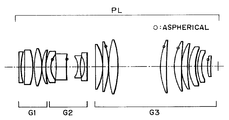•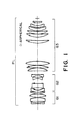•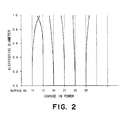•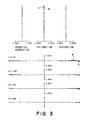•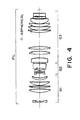•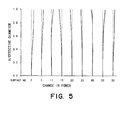•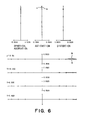•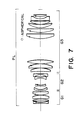•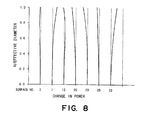•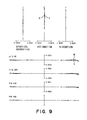•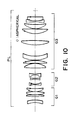•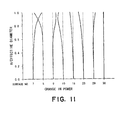•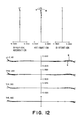•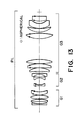•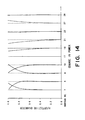•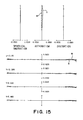•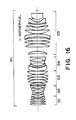•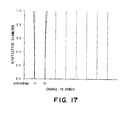•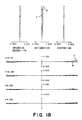•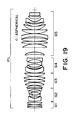•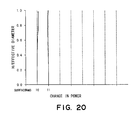•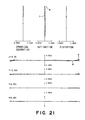•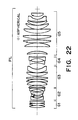•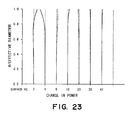•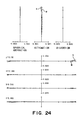•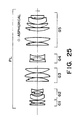•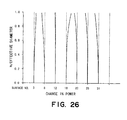•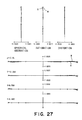•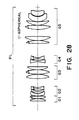•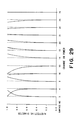•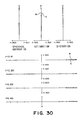••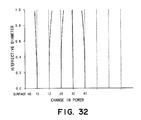•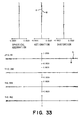•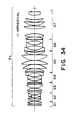•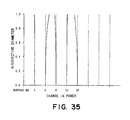•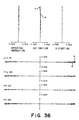•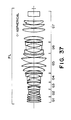•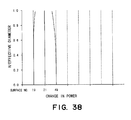•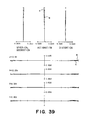•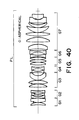•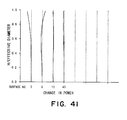•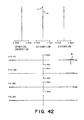•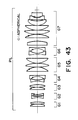•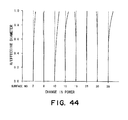•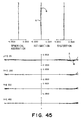•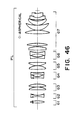••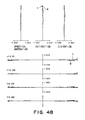•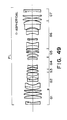•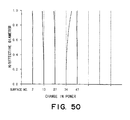•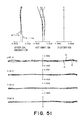•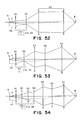•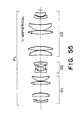•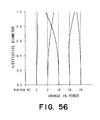•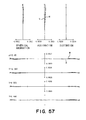•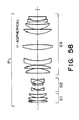•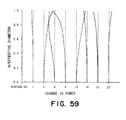•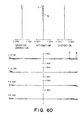•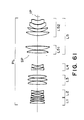•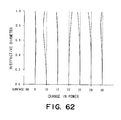•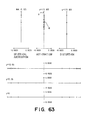•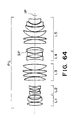•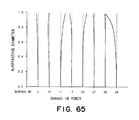•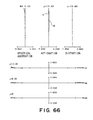•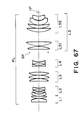•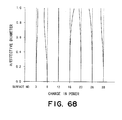•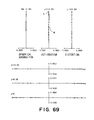•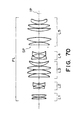••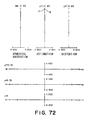•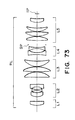•••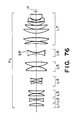•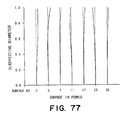•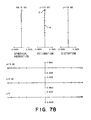•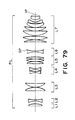•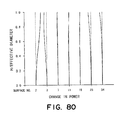•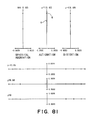•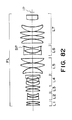•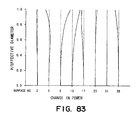•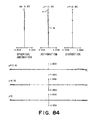•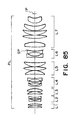•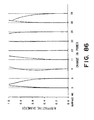•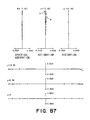•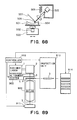•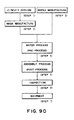•Classifications

• GPHYSICS
• G03PHOTOGRAPHY; CINEMATOGRAPHY; ANALOGOUS TECHNIQUES USING WAVES OTHER THAN OPTICAL WAVES; ELECTROGRAPHY; HOLOGRAPHY
• G03FPHOTOMECHANICAL PRODUCTION OF TEXTURED OR PATTERNED SURFACES, e.g. FOR PRINTING, FOR PROCESSING OF SEMICONDUCTOR DEVICES; MATERIALS THEREFOR; ORIGINALS THEREFOR; APPARATUS SPECIALLY ADAPTED THEREFOR
• G03F7/00Photomechanical, e.g. photolithographic, production of textured or patterned surfaces, e.g. printing surfaces; Materials therefor, e.g. comprising photoresists; Apparatus specially adapted therefor
• G03F7/70Exposure apparatus for microlithography
• G03F7/70216Systems for imaging mask onto workpiece
• G03F7/7025Size or form of projection system aperture
• GPHYSICS
• G02OPTICS
• G02BOPTICAL ELEMENTS, SYSTEMS, OR APPARATUS
• G02B13/00Optical objectives specially designed for the purposes specified below
• G02B13/14Optical objectives specially designed for the purposes specified below for use with infra-red or ultra-violet radiation
• G02B13/143Optical objectives specially designed for the purposes specified below for use with infra-red or ultra-violet radiation for use with ultra-violet radiation
• GPHYSICS
• G02OPTICS
• G02BOPTICAL ELEMENTS, SYSTEMS, OR APPARATUS
• G02B13/00Optical objectives specially designed for the purposes specified below
• G02B13/24Optical objectives specially designed for the purposes specified below for reproducing or copying at short object distances
• GPHYSICS
• G03PHOTOGRAPHY; CINEMATOGRAPHY; ANALOGOUS TECHNIQUES USING WAVES OTHER THAN OPTICAL WAVES; ELECTROGRAPHY; HOLOGRAPHY
• G03FPHOTOMECHANICAL PRODUCTION OF TEXTURED OR PATTERNED SURFACES, e.g. FOR PRINTING, FOR PROCESSING OF SEMICONDUCTOR DEVICES; MATERIALS THEREFOR; ORIGINALS THEREFOR; APPARATUS SPECIALLY ADAPTED THEREFOR
• G03F7/00Photomechanical, e.g. photolithographic, production of textured or patterned surfaces, e.g. printing surfaces; Materials therefor, e.g. comprising photoresists; Apparatus specially adapted therefor
• G03F7/70Exposure apparatus for microlithography
• G03F7/70216Systems for imaging mask onto workpiece
• G03F7/70241Optical aspects of refractive systems
• GPHYSICS
• G03PHOTOGRAPHY; CINEMATOGRAPHY; ANALOGOUS TECHNIQUES USING WAVES OTHER THAN OPTICAL WAVES; ELECTROGRAPHY; HOLOGRAPHY
• G03FPHOTOMECHANICAL PRODUCTION OF TEXTURED OR PATTERNED SURFACES, e.g. FOR PRINTING, FOR PROCESSING OF SEMICONDUCTOR DEVICES; MATERIALS THEREFOR; ORIGINALS THEREFOR; APPARATUS SPECIALLY ADAPTED THEREFOR
• G03F7/00Photomechanical, e.g. photolithographic, production of textured or patterned surfaces, e.g. printing surfaces; Materials therefor, e.g. comprising photoresists; Apparatus specially adapted therefor
• G03F7/70Exposure apparatus for microlithography
• G03F7/70691Handling of masks or wafers
• G03F7/70733Handling masks and workpieces, e.g. exchange of workpiece or mask, transport of workpiece or mask
• G03F7/70741Handling masks outside exposure position, e.g. reticle libraries

Abstract

A projection optical system includes a plurality of positive lens groups having a positive refractive power, and at least one negative lens group having a negative refractive power, wherein, when L is a conjugate distance of the projection optical system and φ0 is the sum of powers of the or each negative lens group, a relation |L×φ0|>17 is satisfied, wherein, when h is a height of an axial marginal light ray and hb is a height of a most abaxial chief ray, at least two aspherical surfaces are formed on surfaces which satisfy a relation |hb/h|>0.35, wherein, when ΔASPH is an aspherical amount of each aspherical surface, a relation |ΔASPH/L|>1.0×10−6 is satisfied, and wherein the at least two aspherical surfaces include regions in which, from a central portion toward a peripheral portion of the surface, their local curvature powers change with mutually opposite signs.

Description

FIELD OF THE INVENTION AND RELATED ART

This invention relates to a projection optical system and a projection exposure apparatus using the same. More particularly, the invention is suitably applicable to a projection exposure process for printing a reticle pattern on a photosensitive substrate in accordance with a step-and-repeat method or a step-and-scan method, for the manufacture of large-integration microdevices or semiconductor devices of submicron or quarter-micron order, such as ICs, LSIs, CCDs , or liquid crystal panels, for example.

Generally, in a projection exposure apparatus, a reticle having an electronic circuit pattern formed thereon is illuminated with light (exposure light) from an illumination system (illumination optical system) and the pattern is projected onto a wafer through a projection optical system.

With an increasing density of a semiconductor device, strict requirements have been applied to the performance or specifications of a projection optical system. Generally, for a higher resolution, attempts have been made in relation to shortening the wavelength of the exposure light, better correction of aberrations of a projection optical system, or enlargement of the numerical aperture (NA) of a projection optical system.

As regards the exposure light, light if an i-line lamp or a laser light of an excimer laser such as a KrF or an ArF, for example, is used. Further, use of light from an F2 excimer laser has been proposed.

As regards enlargement of the numerical aperture (NA) of a projection optical system, he NA is being increased from 0.6 to 0.65, and then to 0.7.

As regards aberration correction, many attempts have been made so that a dual-telecentric system (being telecentric on object and image sides) is defined to reduce image distortion resulting from a warp of a reticle or a wafer and, on the other hand, distortion attributable to the projection optical system is reduced as much as possible. Also, the image plane width (field curvature amount) of best image points at each of a plurality of image heights is minimized, while the contrast gain at each of the image heights is made uniform as much as possible.

On the other hand, semiconductor device manufacturing processes use many reticle patterns or linewidths and, in accordance with them, the illumination condition is changed variously to obtain a best pattern image. In order to minimize differences in distortion or image plane flatness, for example, under different illumination conditions, coma aberrations at each of the image heights are reduced to attain registration of the image plane.

Further, while the throughput of a projection exposure apparatus is an important factor, the chip size has been enlarged to increase the throughput. The exposure region of the projection optical system is enlarged to meet this.

As regards a projection optical system for use in a projection exposure apparatus, Japanese Laid-Open Patent Applications, Laid-Open No. 105861/1997, No. 48517/1998, and No. 79345/1998 have proposed projection optical systems wherein all lens systems are defined by spherical surfaces.

Japanese Published Patent Application Publication No. 48089/1995 and Japanese Laid-Open Patent Applications, Laid-Open No. 128592/1995, No. 179204/1996, No. 34593/1993, No. 197791/1998, No. 154657/1998, No. 325922/1998, No. 333030/1998, and No. 6957/1999, have proposed projection optical systems wherein an aspherical surface is used for aberration correction.

In projection optical systems, in order that the shortening of the exposure wavelength and enlargement of the numerical aperture as well as a relatively large exposure region are satisfied while a good optical performance with less change in performance over various illumination modes is held, it is necessary to appropriately set the refractive powers of lens groups (lens units) as well as the lens structures of them.

Generally, in order to obtain a good optical performance with less performance change, the refractive power of each lens group (unit) should be made smaller to reduce the aberration amount to be produced in each lens group or, alternatively, the number of lenses to be used in each lens group should be enlarged to expand the degree of freedom in regard to aberration correction.

Therefore, if use of a shortened exposure wavelength and an enlarged numerical aperture (NA) as well as a wide exposure area are all desired, it necessarily results in enlargement of the lens conjugate distance (object-to-image distance) or an increase in the lens diameter or in the number of lenses, which causes inconveniences such as bulkiness in weight or thickness of the whole lens system.

Then, on that occasion, there arises a problem of deterioration of the imaging performance due to lens deformation of the imaging performance due to lens deformation attributable to an environmental change or a lens assembling precision. With enlargement of the lens diameter, deformation of a lens due to the weight thereof becomes large. Additionally, if a desired performance is to be accomplished within a certain conjugate distance, a larger number of lenses has to be used. Therefore, the thickness of each lens has to be reduced, and the lens deformation due to the weight thereof grows more. With a large lens deformation, the curvature radius of each surface of a lens is deviated from a design value, which causes degradation of the imaging performance. Further, while lenses are held by a metal holding element, in a strict sense and due to a machining precision, it is difficult for such a metal holding element to hold the lenses uniformly. Therefore, if the lens deformation due to the weight thereof becomes large and the lens deforms asymmetrically with respect to an optical axis, an asymmetric aberration is produced thereby. This is a large factor for deterioration of the imaging performance.

Further, with regard to the design performance, there are problems in relation to changes in the best imaging position depending on the linewidth (pattern linewidth), changes in the image point position or contrast depending on the image height, changes in distortion between different illumination conditions, and changes in image plane flatness, for example.

A change in the best imaging position depending on the linewidth is attributable mainly to a remaining spherical aberration not corrected. A change in image point position or contrast depending on the image height is attributable to a change in sagittal and meridional image planes at each image height, or to a change in astigmatism and coma. A change in distortion or in image plane flatness between different illumination conditions results from a remaining distortion amount or an aberration amount in a region upon a pupil plane, passed by light rays, under different illumination conditions. The change in aberration such as described above becomes very noticeable if the shortening of exposure wavelength, the enlargement of numerical aperture and a wide exposure area are pursued more.

There is another problem that, in the short wavelength region of a light source such as an excimer laser, a usable lens material is limited to silica (quartz) and fluorite. This is mainly because of a decreased transmission factor. In an optical system having many lenses and a large total glass thickness, the exposure amount upon a wafer becomes very small such that the throughput is lowered very much. Further, there may occur a shift of the focal point position or a change in aberration.

In order to meet enlargement in the integration of a semiconductor chip, further shortening of the wavelength of the exposure light as well as further enlargement of the numerical aperture of a projection optical system are desired. However, it is very difficult to accomplish a required optical performance while suppressing enlargement in weight and thickness of the whole lens system and production of lens deformation due to the weight thereof. Currently, design optimization is made such that the refractive power of each lens group is made smaller and the number of lenses to be used is made larger.

In the projection optical systems disclosed in Japanese Laid-Open Patent Applications, Laid-Open No. 105861/1997, No. 48517/1998, and No. 79345/1998, all lenses are formed with spherical surfaces. The number of lenses is 27 to 30. The numerical aperture (NA) is about 0.6.

With such a structure, however, if the NA is made larger, aberration correction becomes very difficult to accomplish as long as the same lens number is held. Alternatively, the total lens length has to be enlarged, and also the lens diameter has to be made larger. Even if the lens number is made much larger for correction of aberration, since there is substantially no space for additional lenses, the thickness of each lens must be made small or, alternatively, the total lens length has to be made quite large.

Anyway, the lens deformation due to the weight thereof becomes notable, and the whole lens system becomes bulky. Moreover, if a light source of a short wavelength region is used, the transmission factor decreases due to large absorption by the lens material. In an optical system having lenses of a large number such as disclosed in these documents, the exposure amount upon a wafer decreases much more and the throughput is lowered considerably. Further, the shift of the focal point position of the change in aberration becomes large.

The projection optical systems disclosed in Japanese Published Patent Application, Publication No. 48089/1995 and Japanese Laid-Open Patent Application, Laid-Open No. 128592/1995, have a small numerical aperture (NA). Also, the exposure area is narrow. Because the power of a negative lens group used is small, the optical system is disadvantageous with respect to correction of the Petzval sum. If enlargement of the NA or expansion of the exposure region is attempted in this optical system, particularly, the field curvature would be much worse. Further, since, in both cases, the projection optical system is not made telecentric on the object side, any curvature of a reticle would directly produce image distortion.

In the projection optical system disclosed in Japanese Laid-Open Patent Application, Laid-Open No. 179204/1996, an aspherical surface is formed on the surface closest to the wafer side. However, no particular discussion is made in relation to the aspherical surface. As for the imaging performance, correction of distortion, field curvature and astigmatism is insufficient. There remain large aberrations such as distortion of 26.7 nm and 11.7 nm as well as abaxial astigmatism of 1.262 micron and 0.896 micron.

The projection optical system disclosed in Japanese Laid-Open Patent Application, Laid-Open No. 34593/1993 uses an aspherical surface, and the lens system is provided by a smaller number of lenses to keep the lens transmission factor and for correction of aberrations. However, as regards the numerical aperture (NA) which largely contributes to the resolution, it may be as small as 0.45. Also, the lens system has a very small exposure area of 10×10 to 15×15. According to the disclosure, aspherical surfaces are introduced into a negative second group and a positive fourth group, mainly for correction of spherical aberration. The power of the negative second group is enlarged while, on the other hand, an aspherical surface is added to the second group for correction of spherical aberration based on the balance with other positive lens groups. Also, an aspherical surface is introduced into a fourth group having a large axial light flux diameter, for correction of spherical aberration.

However, in the projection optical system, the height of an axial marginal light ray of the second group is very low as compared with those of the third and fourth groups and, therefore, correcting the spherical aberration with the introduction of an aspherical surface is practically very difficult. The reason is that the third-order spherical aberration coefficient is proportional to the fourth power of the height “h” of an axial margin light ray.

Also, the height of an outermost abaxial chief ray of the second group is very low, and the light goes just along the optical axis. Therefore, although the aspherical surface itself may function to correct aberrations such as distortion, field curvature or astigmatism while keeping the telecentricity on the object side, the effect is small. The reason is that the third-order astigmatism coefficient or field curvature coefficient is proportional to the second power of the chief ray height, and the distortion coefficient is proportional to the third power of the chief ray height.

According to this example, even if it is attempted to meet a larger numerical aperture (about 0.65) or enlargement of the exposure region (φ is about 27.3 mm), since the power of the second group which bears most of the negative power is made very small, the Petzval sum cannot be corrected. As a result, field curvature and astigmatism become worse. Additionally, as the light flux on the object side becomes large with enlargement of the numerical aperture, since the positive first group and negative second group on the object side (which function mainly to correct the field curvature, distortion and telecentricity on the object side) are provided by only a single lens, respectively, the load for aberration correction to be borne by these lens groups becomes considerably heavy. Thus, it becomes very difficult to keep good imaging performance.

The projection optical system disclosed in Japanese Laid-Open Patent Application, Laid-Open No. 197791/1998, provides a relatively wide exposure area and a relatively high resolution with the use of a smaller number of lenses. While the exposure area is about φ25-φ29, the numerical aperture is 0.48-0.50. However, this is still insufficient.

The projection optical system disclosed in Japanese Laid-Open Patent Application, Laid-Open No. 154657/1998, uses an aspherical surface. However, as stated, this aspherical surface is not designed to correct aberrations to provide a projection optical system of particular specifications. Rather, it is designed to correct higher order aberrations resulting from an error in production or adjustment of the components of the projection optical system.

Since the optical design is made entirely on a spherical system, even if the manufacturing error can be corrected by the aspherical surface, a performance higher than the design performance of the spherical system is not be attainable thereby. In fact, the aspherical amount is very small. Therefore, it is very difficult to solve the above-described problem, in an attempt to meet a larger numerical aperture.

The projection optical system disclosed in Japanese Laid-Open Patent Application, Laid-Open No. 325922/1998, comprises five lens groups, wherein one of first and second lens groups is provided with one aspherical surface, and one of fourth and fifth lens groups is provided with one aspherical surface. It is intended that, with a smaller number of lenses, mainly distortion and spherical aberration are corrected.

In the embodiments disclosed therein, the numerical aperture is 0.6. Moreover, no aspherical surface is used for the fourth lens group. While field curvature and astigmatism may be corrected well, there remain higher order components of “under” spherical aberration. Also, the distortion aberration is as large as about 30 nm at the largest image height.

In this document, it is proposed to use an aspherical surface for a surface by which a large aberration correction effect is attainable. However, further details are not discussed any more. Therefore, aberrations may become worse if it is attempted to meet the numerical aperture enlargement.

If use of additional lenses is attempted to expand the degree of freedom for correction, although there is a small space at the image plane side and the spherical aberration may be corrected, in the region of the first to third groups at the object side, there are lenses disposed tightly. Thus, no lens can be added there. It is difficult to correct field curvature, astigmatism and distortion, for example. If the lens thickness is made small and an additional lens is inserted, then, the deformation will occur due to the weight of the lens.

The projection optical system disclosed in Japanese Laid-Open Patent Application, Laid-Open No. 333030/1998 uses aspherical surfaces and, according to the disclosure, it accomplishes an NA of 0.63-0.75 and an exposure area of φ¢27-30 mm, with a smaller number of lenses of about fifteen (15) . According to the disclosure, the lens system comprises positive two groups, wherein a first group at a wafer side is a microscope objective lens and a second group at a reticle side is a Gaussian type lens, by which sagittal comas produced in these lens groups are cancelled with each other. At least one aspherical surface is introduced into the wafer side group, and also an aspherical surface of the second group, for correction of spherical aberration.

However, the sagittal coma is not illustrated, and details of its correction are unclear. According to simulations made by the inventors of the subject application, changes in sagittal halo due to the image height or meridional coma aberration (transverse aberration) are large.

Further, while spherical aberration and astigmatism may be correct, as regards the distortion aberration, there remain large higher order components. The largest values in the first to fourth embodiments disclosed are 12 nm, 45 nm, 26 nm and 46 nm. As regards the telecentricity on the wafer side, in the first to fourth embodiments, changes in image height per a focal depth of 1 micron are 24 nm, 22 nm, 19 nm and 19 nm.

Furthermore, since the distance from the wafer to the lens is as short as 11-12 mm, there is a large possibility of mechanical interference with a mechanism such as an autofocusing unit.

The projection exposure apparatus disclosed in Japanese Laid-Open Patent Application, Laid-Open No. 6957/1999 provides a large NA (NA=0.75 to 0.80) by use of aspherical surfaces. According to the disclosure, at least one aspherical surface is formed in the fourth or fifth lens group, for correction of aberrations very influential to enlargement of the NA, that is, sagittal coma aberration and higher order spherical aberration.

In the embodiments disclosed therein, however, sagittal coma is not illustrated. The number of lenses is as large as 27 to 29. The optical conjugate distance is as long as 1200-1500 mm.

Therefore, if the light source has a wavelength in the short wavelength region as an ArF excimer laser, for example, the exposure amount upon a wafer would be considerably lowered due to large absorption by the lens material, and the throughput becomes very low. Further, the thermal absorption of the lens causes shift of the focal point position or a change in aberration. Moreover, because of the large NA and long lens conjugate distance, the lens diameter is large as φ284-400 mm. Lens deformation due to the weight will be large. There is no space available, and thus suppressing the deformation by reducing the lens conjugate distance or increasing the number of lenses is difficult to do.

The lens number may be reduced by increasing the number of aspherical surfaces. However, in the first to fifth embodiments disclosed, while the number of aspherical surfaces used is increasing, the lens systems use twenty-nine (29) lenses. Even in the fifth embodiment wherein a largest number of aspherical surfaces of six is used, there remains a sagittal image plane at the outermost abaxial image height of −0.484 micron. Further, distortion aberration is 13.1 nm, and also there remains higher order spherical aberration.

In the sixth and seventh embodiments disclosed, the distortion aberration is 33 nm and 58 nm, respectively. In the eighth and ninth embodiments, while the performance cannot be confirmed due to insufficient data disclosure, the lens conjugate distance is as extraordinarily large as 1500 mm.

SUMMARY OF THE INVENTION

It is an object of the present invention to provide a projection optical system and/or a projection exposure apparatus using the same, by which a larger numerical aperture and a wider exposure area can be well accomplished.

In accordance with a first aspect of the present invention, there is provided a projection optical system, comprising: a plurality of positive lens groups (units) having a positive refractive power; and at least one negative lens group (unit) having a negative refractive power; wherein, when L is a conjugate distance of said projection optical system and φ0 is the sum of powers of the or each negative lens group, a relation
|L×φ 0|>17  (1)
0=Σφ0i where φ0i is the power of the i-th negative group) is satisfied; wherein, when h is a height of an axial marginal light ray and hb is a height of a most abaxial chief ray, at least two aspherical surfaces are formed on surfaces which satisfy a relation
|h b /h|>0.35  (2)
wherein, when ΔASPH is an aspherical amount of each aspherical surface, a relation
|ΔASPH/L|>1.0×10−6  (3)
is satisfied; and wherein said at least two aspherical surfaces include regions in which, from a central portion toward a peripheral portion of the surface, their local curvature powers change with mutually opposite signs.

In a second aspect based on the first aspect, said at least two aspherical surfaces are formed on those surfaces up to one, in an order from the object side, which satisfies a relation
|h b /h>0.35  (2)

In a third aspect based on the first or second aspect, at least one of said at least two aspherical surfaces is provided in a negative lens group and includes a region in which, from the central portion to the peripheral portion of the surface, the local curvature power thereof gradually increases in the negative direction or gradually decreases in the positive direction.

In a fourth aspect based on the first to third aspects, the following relations
|L×φ 0|<70  (1a)
|h b /h|<15  (2a)
|ΔASPH/L|<0.02  (3a)
are satisfied.

In accordance with a fifth aspect of the present invention, there is provided a projection optical system, comprising: a plurality of positive lens groups having a positive refractive power; and at least one negative lens group having a negative refractive power; wherein, when L is a conjugate distance of said projection optical system and φ0 is the sum of powers of the or each negative lens group, a relation
|L×φ 0|>17  (1)
is satisfied; wherein, when h is a height of an axial marginal light ray hb is a height of a most abaxial chief ray, at least one aspherical surface is formed on a surface in the negative lens group which satisfies a relation
|h b /h>0.35  (2)
wherein, when ΔASPH is an aspherical amount of the aspherical surface, a relation
|ΔASPH/L|>1.0×10−6  (3)
is satisfied; and wherein said at least one aspherical surface includes a region in which, from a central portion toward a peripheral portion of the surface, a local curvature power thereof increases in the negative direction or decreases in the positive direction.

In a sixth aspect based on the fifth aspect, at least one aspherical surface is provided in a positive lens group and is formed upon one of the surfaces up to one, in an order from the object side, which satisfies a relation
|h b /h|>0.35  (2)
and wherein said at least one aspherical surface includes a region in which, from the central portion to the peripheral portion of the surface, the local curvature power thereof gradually increases in the positive direction or gradually decreases in the negative direction.

In a seventh aspect based on the fifth or sixth aspect, the following relations
|L×φ 0|<70  (1a)
|h b /h|<15  (2a)
|ΔASPH/L|<0.02  (3a)
are satisfied.

In accordance with an eighth aspect of the present invention, there is provided a projection optical system, comprising: three lens groups of a lens group having a positive refractive power, a lens group having a negative refractive power, and a lens group having a positive refractive power, which are disposed in this order from the object side; wherein, when L is a conjugate distance of said projection optical system and φ0 is the power of said second negative refractive power lens group, a relation
|L×φ 0|>17  (1)
is satisfied; wherein, when h is a height of an axial marginal light ray and hb is a height of a most abaxial chief ray, at least two aspherical surfaces are formed on surfaces which satisfy a relation
|h b /h|>0.35  (2)
wherein, when ΔASPH is an aspherical amount of each aspherical surface, a relation
|ΔASPH/L|>1.0×10−6  (3)
is satisfied.

In a ninth aspect based on the eighth aspect, said at least two aspherical surfaces include regions in which, from a central portion toward a peripheral portion of the surface, their local curvature powers change with mutually opposite signs.

In a tenth aspect based on the eighth or ninth aspect, an aspherical surface is provided in the positive lens group closest to the object side, which aspherical surface includes a region in which, from the central portion to the peripheral portion of the surface, the local curvature power thereof gradually increases in the positive direction or gradually decreases in the negative direction.

In an eleventh aspect based on the eighth to tenth aspects, an aspherical surface is provided in the positive lens group closest to the image plane side, which aspherical surface includes a region in which, from the central portion to the peripheral portion of the surface, the local curvature power thereof gradually increases in the negative direction or gradually decreases in the positive direction.

In a twelfth aspect based on the eighth to eleventh aspects, a or each lens group disposed after, in an order from the object side to the image plane side, the sign of an abaxial chief ray height is reversed, has at least one aspherical surface formed thereon.

In a thirteenth aspect based on the eighth to twelfth aspects, in the positive lens group disposed closest to the image plane side, a second lens thereof in an order from the image plane side comprises a negative lens having a concave surface facing to the image side, and a lens of that lens group, which lens is closest to the image plane side, comprises a positive lens having a concave surface facing to the image plane side.

In a fourteenth aspect based on the eighth to thirteenth aspects, at least one aspherical lens with an aspherical surface has a plane surface formed on its side opposite to the aspherical surface thereof.

In a fifteenth aspect based on the eighth to thirteenth aspects, each aspherical lens with an aspherical surface has a plane surface formed on its side opposite to the aspherical surface thereof.

In a sixteenth aspect based on the eighth to thirteenth aspects, at least one aspherical lens provided in said projection optical system has two aspherical surfaces formed on the opposite sides thereof.

In a seventeenth aspect based on the eighth to thirteenth aspects, each aspherical lens provided in said projection optical system has two aspherical surfaces formed on the opposite sides thereof.

In an eighteenth aspect based on the eighth to seventeenth aspects, the following relations
|L×φ 0|<70  (1a)
|h b /h|<15  (2a)
ΔASPH/L|<0.02  (3a)
are satisfied.

In accordance with a nineteenth aspect of the present invention, there is provided a projection optical system, comprising: a first lens group L1 having a positive refractive power, a second lens group L2 having a negative refractive power, a third lens group L3 having a positive refractive power, a fourth lens group L4 having a negative refractive power, and a fifth lens group L5 having a positive refractive power, which are disposed in this order from the object side; wherein, when h is a height of an axial marginal light ray and hb is a height of a most abaxial chief ray, at least two aspherical surfaces are formed on surfaces which satisfy a relation
|L×φ 0|>17  (1)
wherein, when ASPH is a largest aspherical amount of each asperical surface from an optical axis to a lens effective diameter and L is an object-to-image distance, each aspherical surface satisfies a relation
|h b /h|>0.35  (2)
wherein said aspherical surfaces include regions in which, from a central portion to a peripheral portion of the surface, their local curvature powers change with mutually opposite signs.

In a twentieth aspect based on the nineteenth aspect, at least one of said at least two aspherical surfaces includes a region in which, from the central portion to the peripheral portion of the surface, the local curvature power thereof gradually increases in the negative direction.

In a twenty-first aspect based on the eighteenth or nineteenth aspect, at least one of said at least two aspherical surfaces is provided in a lens group having a negative refractive power.

In accordance with a twenty-second aspect of the present invention, there is provided a projection optical system, comprising: a first lens group L1 having a positive refractive power, a second lens group L2 having a negative refractive power, a third lens group L3 having a positive refractive power, a fourth lens group L4 having a negative refractive power, and a fifth lens group L5 having a positive refractive power, which are disposed in this order from the object side; wherein, when h is a height of an axial marginal light ray and hb is a height of a most abaxial chief ray, at least one aspherical surface is formed on a surface which satisfies a relation
|L×φ 0|>17  (1)
wherein, when ΔASPH is a largest aspherical amount of said aspherical surface from an optical axis to a lens effective diameter and L is an object-to-image distance, said aspherical surface satisfies a relation
|h b /h|>0.35  (2)
wherein said aspherical surface includes a region in which, from a central portion to a peripheral portion of the surface, a local curvature power thereof gradually increases in the negative direction.

In a twenty-third aspect based on the nineteenth to twenty-second aspects, at least one aspherical lens provided in said projection optical system has a plane surface formed on its side opposite to the aspherical surface thereof.

In a twenty-fourth aspect based on the nineteenth to twenty-second aspects, each aspherical lens provided in said projection optical system has a plane surface formed on its side opposite to the aspherical surface thereof.

In a twenty-fifth aspect based on the nineteenth to twenty-second aspects, at least one aspherical lens provided in said projection optical system has aspherical surfaces formed on the opposite sides thereof.

In a twenty-sixth aspect based on the nineteenth to twenty-second aspects, each aspherical lens provided in said projection optical system has aspherical surfaces formed on the opposite sides thereof.

In a twenty-seventh aspect based on the nineteenth to twenty-second aspects, the following relations
|h b /h|<15  (2a)
|ΔASPH/L|<0.02  (3a)
are satisfied.

In a twenty-eighth aspect based on the nineteenth to twenty-seventh aspects, when L is an object-to-image distance of said projection optical system and φ0 is the sum of powers of the negative lens groups, a relation
|L×φ 0|<70  (3)
o=Σφoi where φoi is the power of the i-th negative group)
is satisfied.

In a twenty-ninth aspect based on the twenty-eighth aspect, a relation
|L×φ 0|<70  (1a)
is satisfied.

In accordance with a thirtieth aspect of the present invention, there is provided a projection optical system, comprising: a first lens group L1 having a positive refractive power, a second lens group L2 having a negative refractive power, a third lens group L3 having a positive refractive power, a fourth lens group L4 having a negative refractive power, a fifth lens group L5 having a positive refractive power, a sixth lens group having a negative refractive power, and a seventh lens group having a positive refractive power, which are disposed in this order from the object side; wherein one or more aspherical surfaces are formed in said projection optical system; and wherein, when ΔASPH is a largest aspherical amount of each aspherical surface from an optical axis to a lens effective diameter and L is an object-to-image distance, at least one aspherical surface satisfies a relation
|ΔASPH/L|>1.0×10−6  (3)

In a thirty-first aspect based on the thirtieth aspect, at least one aspherical surface is provided between a first lens surface closest to the object side and a stop position.

In a thirty-second aspect based on the thirtieth or thirty-first aspect, when h is a height of an axial marginal light ray and hb is a height of a most abaxial chief ray, at least one aspherical surface is formed on a surface which satisfies a relation
|h b /h|>0.35  (2)

In a thirty-third aspect based on the thirty-second aspect, at least two aspherical surfaces are formed in said projection optical system.

In a thirty-fourth aspect based on the thirtieth to thirty-third aspects, the aspherical surface is provided in a lens group having a negative refractive power, and at least one aspherical surface in the lens group of negative refractive power includes a region in which, from a central portion to a peripheral portion of the surface, a local curvature power thereof gradually increases in the negative direction.

In a thirty-fifth aspect based on the thirtieth to thirty-fourth aspects, at least two aspherical surfaces include regions in which, from a central portion to a peripheral portion of the surface, their local curvature powers change with mutually opposite signs.

In a thirty-sixth aspect based on the thirtieth to thirty-fifth aspects, at least one aspherical lens provided in said projection optical system has a plane surface formed on its side opposite to the aspherical surface thereof.

In a thirty-seventh aspect based on the thirtieth to thirty-fifth aspects, each aspherical lens provided in said projection optical system has a plane surface formed on its side opposite to the aspherical surface thereof.

In a thirty-eighth aspect based on the thirtieth to thirty-fifth aspects, at least one aspherical lens provided in said projection optical system has aspherical surfaces formed on the opposite sides thereof.

In a thirty-ninth aspect based on the thirtieth to thirty-fifth aspects, each aspherical lens provided in said projection optical system has aspherical surfaces formed on the opposite sides thereof.

In a fortieth aspect based on the thirtieth to thirty-ninth aspects, when L is an object-to-image distance of said projection optical system and φ is the sum of powers of the negative lens group, a relation
|L×φ 0|<70  (1)
o=Σφoi where φ is the power of the i-th negative group)
is satisfied.

In a forty-first aspect based on the thirtieth to thirty-third aspects, a relation
|ΔASPH/L|<0.02  (3a)
is satisfied.

In a forty-second aspect based on the thirty-second to thirty-third aspects, a relation
|h b /h|<15  (2a)
is satisfied.

In a forty-third aspect based on the fortieth aspect, a relation
|L×φ 0|<70  (1a)
is satisfied.

In accordance with a forty-fourth aspect of the present invention, there is provided a projection exposure apparatus for projecting a pattern of a first object, illuminated with light from a light source, onto a second object by use of a projection optical system as recited above.

In accordance with a forty-fifth aspect of the present invention, there is provided a projection exposure apparatus for projecting pattern of a first object, illuminated with light from a light source onto a second object by use of a projection optical system as recited above, while scanningly moving the first and second objects in a direction perpendicular to an optical axis of said projection optical system, in synchronism with each other and at a speed ratio corresponding to a projection magnification of said projection optical system.

In accordance with a forty-sixth aspect of the present invention, there is provided a device manufacturing method, comprising the steps of: exposing a wafer to a device pattern of a reticle by use of a projection exposure apparatus as recited above; and developing the exposed wafer.

These and other objects, features and advantages of the present invention will become more apparent upon a consideration of the following description of the preferred embodiments of the present invention taken in conjunction with the accompanying drawings.

BRIEF DESCRIPTION OF THE DRAWINGS

FIG. 1 is a lens sectional view of a projection optical system for use in a projection exposure apparatus, according to Numerical Example 1 of the present invention.

FIG. 2 is a graph for explaining changes in a local curvature power of an aspherical surface of a projection optical system according to Numerical Example 1.

FIG. 3 illustrates aberrations of a projection optical system according to Numerical Example 1.

FIG. 4 is a lens sectional view of a projection optical system for use in a projection exposure apparatus, according to Numerical Example 2 of the present invention.

FIG. 5 is a graph for explaining changes in a local curvature power of an aspherical surface of a projection optical system according to Numerical Example 2.

FIG. 6 illustrates aberrations of a projection optical system according to Numerical Example 2.

FIG. 7 is a lens sectional view of a projection optical system for use in a projection exposure apparatus, according to Numerical Example 3 of the present invention.

FIG. 8 is a graph for explaining changes in a local curvature power of an aspherical surface of a projection optical system according to Numerical Example 3.

FIG. 9 illustrates aberrations of a projection optical system according to Numerical Example 3.

FIG. 10 is a lens sectional view of a projection optical system for use in a projection exposure apparatus, according to Numerical Example 4 of the present invention.

FIG. 11 is a graph for explaining changes in a local curvature power of an aspherical surface of a projection optical system according to Numerical Example 4.

FIG. 12 illustrates aberrations of a projection optical system according to Numerical Example 4.

FIG. 13 is a lens sectional view of a projection optical system for use in a projection exposure apparatus, according to Numerical Example 5 of the present invention.

FIG. 14 is a graph for explaining changes in a local curvature power of an aspherical surface of a projection optical system according to Numerical Example 5.

FIG. 15 illustrates aberrations of a projection optical system according to Numerical Example 5.

FIG. 16 is a lens sectional view of a projection optical system for use in a projection exposure apparatus, according to Numerical Example 6 of the present invention.

FIG. 17 is a graph for explaining changes in a local curvature power of an aspherical surface of a projection optical system according to Numerical Example 6.

FIG. 18 illustrates aberrations of a projection optical system according to Numerical Example 6.

FIG. 19 is a lens sectional view of a projection optical system for use in a projection exposure apparatus, according to Numerical Example 7 of the present invention.

FIG. 20 is a graph for explaining changes in a local curvature power of an aspherical surface of a projection optical system according to Numerical Example 7.

FIG. 21 illustrates aberrations of a projection optical system according to Numerical Example 7.

FIG. 22 is a lens sectional view of a projection optical system for use in a projection exposure apparatus, according to Numerical Example 8 of the present invention.

FIG. 23 is a graph for explaining changes in a local curvature power of an aspherical surface of a projection optical system according to Numerical Example 8.

FIG. 24 illustrates aberrations of a projection optical system according to Numerical Example 8.

FIG. 25 is a lens sectional view of a projection optical system for use in a projection exposure apparatus, according to Numerical Example 9 of the present invention.

FIG. 26 is a graph for explaining changes in a local curvature power of an aspherical surface of a projection optical system according to Numerical Example 9.

FIG. 27 illustrates aberrations of a projection optical system according to Numerical Example 9.

FIG. 28 is a lens sectional view of a projection optical system for use in a projection exposure apparatus, according to Numerical Example 10 of the present invention.

FIG. 29 is a graph for explaining changes in a local curvature power of an aspherical surface of a projection optical system according to Numerical Example 10.

FIG. 30 illustrates aberrations of a projection optical system according to Numerical Example 10.

FIG. 31 is a lens sectional view of a projection optical system for use in a projection exposure apparatus, according to Numerical Example 11 of the present invention.

FIG. 32 is a graph for explaining changes in a local curvature power of an aspherical surface of a projection optical system according to Numerical Example 11.

FIG. 33 illustrates aberrations of a projection optical system according to Numerical Example 11.

FIG. 34 is a lens sectional view of a projection optical system for use in a projection exposure apparatus, according to Numerical Example 12 of the present invention.

FIG. 35 is a graph for explaining changes in a local curvature power of an aspherical surface of a projection optical system according to Numerical Example 12.

FIG. 36 illustrates aberrations of a projection optical system according to Numerical Example 12.

FIG. 37 is a lens sectional view of a projection optical system for use in a projection exposure apparatus, according to Numerical Example 13 of the present invention.

FIG. 38 is a graph for explaining changes in a local curvature power of an aspherical surface of a projection optical system according to Numerical Example 13.

FIG. 39 illustrates aberrations of a projection optical system according to Numerical Example 13.

FIG. 40 is a lens sectional view of a projection optical system for use in a projection exposure apparatus, according to Numerical Example 14 of the present invention.

FIG. 41 is a graph for explaining changes in a local curvature power of an aspherical surface of a projection optical system according to Numerical Example 14.

FIG. 42 illustrates aberrations of a projection optical system according to Numerical Example 14.

FIG. 43 is a lens sectional view of a projection optical system for use in a projection exposure apparatus, according to Numerical Example 15 of the present invention.

FIG. 44 is a graph for explaining changes in a local curvature power of an aspherical surface of a projection optical system according to Numerical Example 15.

FIG. 45 illustrates aberrations of a projection optical system according to Numerical Example 15.

FIG. 46 is a lens sectional view of a projection optical system for use in a projection exposure apparatus, according to Numerical Example 16 of the present invention.

FIG. 47 is a graph for explaining changes in a local curvature power of an aspherical surface of a projection optical system according to Numerical Example 16.

FIG. 48 illustrates aberrations of a projection optical system according to Numerical Example 16.

FIG. 49 is a lens sectional view of a projection optical system for use in a projection exposure apparatus, according to Numerical Example 17 of the present invention.

FIG. 50 is a graph for explaining changes in a local curvature power of an aspherical surface of a projection optical system according to Numerical Example 17.

FIG. 51 illustrates aberrations of a projection optical system according to Numerical Example 17.

FIG. 52 is a schematic view for explaining an optical function of a three-group system into which the present invention is applied.

FIG. 53 is a schematic view for explaining an optical function of a five-group system into which the present invention is applied.

FIG. 54 is a schematic view for explaining an optical function of a seven-group system into which the present invention is applied.

FIG. 55 is a lens sectional view of a projection optical system for use in a projection exposure apparatus, according to Numerical Example 18 of the present invention.

FIG. 56 is a graph for explaining changes in a local curvature power of an aspherical surface of a projection optical system according to Numerical Example 18.

FIG. 57 illustrates aberrations of a projection optical system according to Numerical Example 18.

FIG. 58 is a lens sectional view of a projection optical system for use in a projection exposure apparatus, according to Numerical Example 19 of the present invention.

FIG. 59 is a graph for explaining changes in a local curvature power of an aspherical surface of a projection optical system according to Numerical Example 19.

FIG. 60 illustrates aberrations of a projection optical system according to Numerical Example 19.

FIG. 61 is a lens sectional view of a projection optical system for use in a projection exposure apparatus, according to Numerical Example 20 of the present invention.

FIG. 62 is a graph for explaining changes in a local curvature power of an aspherical surface of a projection optical system according to Numerical Example 20.

FIG. 63 illustrates aberrations of a projection optical system according to Numerical Example 20.

FIG. 64 is a lens sectional view of a projection optical system for use in a projection exposure apparatus, according to Numerical Example 21 of the present invention.

FIG. 65 is a graph for explaining changes in a local curvature power of an aspherical surface of a projection optical system according to Numerical Example 21.

FIG. 66 illustrates aberrations of a projection optical system according to Numerical Example 21.

FIG. 67 is a lens sectional view of a projection optical system for use in a projection exposure apparatus, according to Numerical Example 22 of the present invention.

FIG. 68 is a graph for explaining changes in a local curvature power of an aspherical surface of a projection optical system according to Numerical Example 22.

FIG. 69 illustrates aberrations of a projection optical system according to Numerical Example 22.

FIG. 70 is a lens sectional view of a projection optical system for use in a projection exposure apparatus, according to Numerical Example 23 of the present invention.

FIG. 71 is a graph for explaining changes in a local curvature power of an aspherical surface of a projection optical system according to Numerical Example 23.

FIG. 72 illustrates aberrations of a projection optical system according to Numerical Example 23.

FIG. 73 is a lens sectional view of a projection optical system for use in a projection exposure apparatus, according to Numerical Example 24 of the present invention.

FIG. 74 is a graph for explaining changes in a local curvature power of an aspherical surface of a projection optical system according to Numerical Example 24.

FIG. 75 illustrates aberrations of a projection optical system according to Numerical Example 24.

FIG. 76 is a lens sectional view of a projection optical system for use in a projection exposure apparatus, according to Numerical Example 25 of the present invention.

FIG. 77 is a graph for explaining changes in a local curvature power of an aspherical surface of a projection optical system according to numerical Example 25.

FIG. 78 illustrates aberrations of a projection optical system according to Numerical Example 25.

FIG. 79 is a lens sectional view of a projection optical system for use in a projection exposure apparatus, according to Numerical Example 26 of the present invention.

FIG. 80 is a graph for explaining changes in a local curvature power of an aspherical surface of a projection optical system according to Numerical Example 26.

FIG. 81 illustrates aberrations of a projection optical system according to Numerical Example 26.

FIG. 82 is a lens sectional view of a projection optical system for use in a projection optical system for use in a projection exposure apparatus, according to Numerical Example 27 of the present invention.

FIG. 83 is a graph for explaining changes in a local curvature power of an aspherical surface of a projection optical system according to Numerical Example 27.

FIG. 84 illustrates aberrations of a projection optical system according to Numerical Example 27.

FIG. 85 is a lens sectional view of a projection optical system for use in a projection optical system for use in a projection exposure apparatus, according to Numerical Example 28 of the present invention.

FIG. 86 is a graph for explaining changes in a local curvature power of an aspherical surface of a projection optical system according to a Numerical Example 28.

FIG. 87 illustrates aberrations of a projection optical system according to Numerical Example 28.

FIG. 88 is a schematic view of an aspherical surface processing system usable in the present invention.

FIG. 89 is a block diagram of a main portion of a semiconductor device manufacturing system according to an embodiment of the present invention.

FIG. 90 is a flow chart of semiconductor device manufacturing processes.

FIG. 91 is a flow chart for explaining details of a wafer process in the procedure of the flow chart of FIG. 90.

DESCRIPTION OF THE PREFERRED EMBODIMENTS

Preferred embodiments of the present invention will now be described with reference to the accompanying drawings.

In these lens sectional views, a reference character PL denotes a projection optical system, and a reference character Gi denotes the i-th lens group (i-th group) of the projection optical system, in an order from the object side (conjugate side of longer distance).

Denoted at IP is an image plane which corresponds to a wafer surface, when the projection optical system is used in a projection exposure apparatus. In the lens groups Gi, those lens groups having an odd number assigned for “i” are lens groups having a positive refractive power, while those lens groups having an even number assigned for “i” are lens groups having a negative refractive power.

Also, those lens surfaces with a small circle added thereto are aspherical surfaces.

In each of Numerical Examples 1-5 and 18 and 19 shown in the lens sectional views of FIGS. 1, 4, 7, 10 and 13 and FIGS. 55 and 56, respectively, the projection optical system comprises three lens groups or lens units (three-group type) which have positive, negative, and positive refractive powers, respectively, in an order from the object side.

In each of Numerical Examples 6-11 and 20-24 shown in the lens sectional view of FIGS. 16, 19, 22, 25, 28 and 31 and FIGS. 61, 64, 67, 70 and 73, respectively, the projection optical system comprises five lens groups or lens units (five-group type) which have positive, negative, positive, negative, and positive refractive powers, respectively, in an order from the object side.

In each of Numerical Examples 12-17 and 25-28 shown in the lens sectional view of FIGS. 34, 37, 40, 43, 47 and 50 and FIGS. 76, 79, 82 and 85, respectively, the projection optical system comprises seven lens groups or lens units (seven-group type) which have positive, negative, positive, negative, positive, negative, and positive refractive powers, respectively, in an order from the object side.

In these numerical examples, while appropriate power sharing is made through the whole lens system, aspherical surfaces are introduced to appropriate lens faces, by which a good optical performance is accomplished.

Projection optical systems according to the present invention provide a large numerical aperture and a wide exposure area.

To this end, the optical system as a whole comprises a plurality of lens groups including a lens group having a positive refractive power and a lens group having a negative refractive power, wherein the power sharing (refractive power sharing) is set appropriately.

In one preferred form of the present invention, for appropriate setting of power sharing, the condition as defined by equation (1) is set in regard to the product of the conjugate distance L of the lens system and the sum φ of powers of the negative lens group or groups. Generally, if the conjugate distance (object-to-image distance) L becomes longer, the total power φ of the negative lens groups becomes smaller. If, on the other hand, the conjugate distance becomes shorter, the total power φ of the negative lens groups becomes larger.

In one preferred form of the present invention, the product of the conjugate distance and the total power is set to be not less than 17. The total power of the negative refractive power lens group is therefore made larger, mainly for satisfactory correction of the curvature of image field and the astimagtism. If the lower limit of the condition of equation (1) is exceeded, the Petzval sum increases in the positive direction, such that satisfactory correction of the curvature of image field or astimagtism becomes difficult to accomplish.

The condition of equation (2) defines an appropriate surface for introduction of an aspherical surface, based on satisfaction of the condition (1). In conventional reduction projection optical systems, it is very difficult to satisfactorily correct distortion, curvature of image field, and astimagtism as well as transverse aberrations of meridional and sagittal, while maintaining the telecentricity.

This is because of the following reason. The telecentricity, distortion, curvature of image field, and astimagtism are all aberration amounts related to a principal ray passing through the center of a light flux. Although these aberrations depend on the placement and shape of lenses on the object side where, in the lens system as a whole, the height of the principal ray is high, practically it is very difficult to maintain, on one hand, the telecentricity with respect to principal rays from every object point on the object and, on the other hand, to refract the same principal ray so as to correct the distortion, the curvature of image field and the astigmatism.

Further, since on a lens surface those rays below the meridional are refracted at a height still higher than the principal ray, it is difficult to balance the meridional transverse aberration and aberration concerning these principal rays. Simultaneously, in order to correct the curvature of image field which has a tendency that it is “under” with higher image height, sually a concave lens is used to refract the light strongly. Then, however, the peripheral portion (sagittal halo) of the sagittal transverse aberration at high image heights further changes “over”. Thus, it is difficult to balance them satisfactority.

Enlarging the numerical aperture and widening the exposure area under these situations directly lead to further enlargement of the object side light flux and image height, and it amplifies the difficulties in aberration correction.

In one preferred form of the present invention, in consideration of the above, the condition of equation (2) is satisfied and an aspherical surface is formed on such surface having a large influence to abaxial principal rays, thereby to concentratedly and effectively correct the above-described aberrations to be improved. This effectively reduces the load for correction of other aberrations, and accomplishes a good optical performance.

If the lower limit of the condition of equation (2) is exceeded, the influence to axial marginal light rays increases, rather than to the abaxial principal rays, and therefore, the effect of correcting the aberrations to be improved diminishes. Thus, it becomes difficult to attain an enlarged numerical aperture and a wider exposure area.

For better understanding of the present invention, an optical function of a projection optical system when an aspherical surface is introduced thereto will now be described. FIGS. 52, 53 and 54 are schematic views, respectively, of power arrangement in examples wherein the whole lens system comprises three lens groups, five lens groups and seven lens groups, respectively.

Denoted in these drawings at R is a reticle as an object, and denoted at W is a wafer as an image plane. Solid lines depict axial marginal light rays and the surface height thereof is denoted by h. Broken lens depict most abaxial chief rays and the surface height thereof is denoted by hb. The optical system is bi-telectnric, both on the object side and the image side.

In the reduction projection systems shown in FIGS. 52, 53 and 54, the object side numerical aperture corresponds to the product of the projection magnification and the image side numerical aperture (the term “numerical aperture” referred to in this specification means the image side numeral aperture). Therefore, the axial marginal light is small at the object side and it is large at the image side.

For this reason, the height h is small at the object side, and it is large at the image side. To the contrary, because of the projection magnification, the height hb of the most abaxial chief ray is high at the object side, and it is low at the image side.

Generally, at a surface where third order aberrations are large, there exist large higher-order aberrations. Thus, it is necessary for better aberration correction that an absolute value of the third order aberration coefficient at each surface is made small and that the value as a whole is made small. The distortion aberration coefficient is influential with the third power of hb and the first power of h and the curvature of field and astigmatism coefficients are influential with the second power of hb and second power of h. The coma aberration coefficient is influential with the first power of hb1 and third power of h, and the spherical aberration coefficient is influential with the fourth power of h.

In FIG. 52, the heights h and hb that satisfy condition (2) are from the object surface to the lens groups GI and G2. Thus, introducing at least one aspherical surface into the group G1 or G2 is a condition for better optical performance. When an aspherical surface is introduced into the lens group G1, the height hb becomes highest and, therefore, it is very effective to control the distortion aberration coefficient.

Introducing an aspherical surface into the lens group G2 having a negative refractive power is effective to control mainly the field curvature and astigmatism aberration coefficients. Since however it is in a cancelling relation with the lens group G1 having a positive refractive power, the distortion aberration coefficient can also be controlled effectively.

Generally, at a surface having a large height h, using an aspherical surface contributes to the spherical aberration coefficient or the coma aberration coefficient. Therefore, introducing an aspherical surface to the lens group G3 having a positive refractive power is effective for correction of spherical aberration or coma aberration.

Also, in FIG. 53, while condition (2) is satisfied between the object surface to the lens groups G1 and G2, since there are two lens groups of negative refractive power, it is advantageous with respect to correction of the Petzval sum (because the necessity of refracting light rays at low positions so as to enlarge the power of the negative lens group is diminished). Further, the spacing between the lens groups G1 and G2 is made small. Therefore, the value of condition (2) in the group G2 is large as compared with the three-group type of FIG. 52.

Thus, while introducing at least one aspherical surface into lens group G1 or G2 is a condition for better optical performance, the aberration correcting ability is larger than that of the three-group type lens system of FIG. 52. The controllability of aberration coefficients in the lens groups G1 and G2 is similar to that of the three-group type lens system of FIG. 52.

Here, because there are two negative lens groups included, correction of field curvature or astigmatism is easier than with the three-group type lens system of FIG. 52. The load for aberration correction applied to the lens group G2 is smaller. As an alternative, aspherical surfaces may be introduced into lens groups G3, G4 and G5 of a large height h, for correction of spherical aberration or coma aberration.

In FIG. 54, condition (2) can be satisfied between the object surface and the lens groups G1, G2, G3 and G4. As compared with the three-group type lens system of FIG. 52 and the five-group type lens system of FIG. 53, the most abaxial chief ray can easily keep a high position relative to the axial marginal light ray. This is because there are three negative lens groups included which is advantageous for correction of the Petzval sum, and because the spacings among the lens groups G1, G2, G3 and G4 are made small.

Thus, an aspherical surface can be introduced more effectively, and better optical performance can be accomplished.

Particularly, by introducing an aspherical surface to the lens groups G1 or G2 showing a large value with respect to condition (2), distortion aberration, curvature of field and astigmatism can be corrected successfully. As regards lens groups G3 and G4, they are suitable mainly for correction of coma aberration and sagittal transverse aberration.

Using an aspherical surface in the lens group G5, G6 or G7 having a large height h is effective to correct spherical aberration or coma aberration.

While the optical function in relation to introducing of an aspherical surface has been described above, for more effective introduction of an aspherical surface in obtaining better imaging performance, the condition of equation (3) should preferably be satisfied.

Equation (3) defines a condition for the aspherical amount. If the lower limit of condition (3) is exceeded, the effect of aspherical surface does not function well even though the aspherical surface is used in design to obtain a good imaging performance. For example, if the conjugate distance is 1000 mm and the wavelength used is 193 nm, from equation (2), ΔASPH is equal to 0.0001 mm which corresponds to about ten Newton's rings. This is a sufficiently large value as an aspherical surface to be used in a projection optical system. Further, for more effective use of an aspherical surface, the following relation may be satisfied
|ΔASPH/L|>1.0×10−5
to enlarge the asperical amount.

The present invention enables effective introduction of an aspherical surface. However, there is a limit to apply a desired change in refractive power for aberration correction, by using a single aspherical surface to plural light beams from an object. Thus, preferably, at least two aspherical surfaces satisfying the above-described condition may be used, to share the aberration correcting function. A better result is attainable with this.

Further, conditions as defined by equations (1a), (2a) and (3a) mentioned above may preferably be satisfied, for much better correction of aberrations.

If the upper limit of the condition of equation (1a) is exceeded, the power of a negative lens group or groups having a negative refractive power becomes too strong. Therefore, the Petzval sum is over-corrected, and it becomes difficult to mainly correct the curvature of image field and astigmatism, satisfactorily.

Further, the lens diameter of a positive lens group having a positive refractive power becomes larger, or the number of lenses increases.

If the upper limit of the condition of equation (2a) is exceeded, lenses become too close to the object plane and the working distance cannot be kept. If the magnification of the projection optical system is extraordinarily small, the working distance may be kept even though the condition is exceeded. However, an optical system having such an extraordinarily small magnification is not practical for use in lithography.

When the upper limit of the condition of equation (3a) is exceeded, the aspherical amount becomes too large, causing a large increase in the time necessary for lens machining.

Further, higher order aberrations produced at the aspherical surface become strong, which makes the satisfactory correction of aberration very difficult.

Further, in relation to the change in curvature of an aspherical surface, the effect of using an aspherical surface can be enhanced significantly when at least one of the following conditions is satisfied.

(a1) There should be at least two aspherical surfaces having regions in which, from a central portion to a peripheral portion of the surface, their local curvature powers change with mutually opposite signs.

(a2) At least one aspherical surface provided in a negative lens group should include a region in which, from a central portion to a peripheral portion of the surface, the local curvature power thereof gradually increases in the negative direction, or gradually decreases in the positive direction.

(a3) At least one aspherical surface among the aspherical surfaces of (a1) and (a2) and provided in a positive lens group, should include a region which, from a central portion to a peripheral portion of the surface, the local curvature power thereof gradually increases in the positive direction, or gradually decreases in the negative direction.

As regards correction of aberration by use of an aspherical surface, generally, an aspherical surface is introduced to a certain lens surface so as to reduce production of aberration at that surface (i.e., auxiliary introduction). An example is that, in a case of a convex single lens, since the spherical aberration is “under”, an aspherical surface whose curvature becomes smaller in the peripheral portion is used to correct the spherical aberration.

In the present invention, as compared therewith, while reducing the number of lenses is aimed at on one hand, spherical surfaces are introduced so as to cancel aberration in combination with other surfaces, to thereby accomplish good performance as well (i.e., positive introduction). By doing so, aberrations are corrected successfully.

More specifically, by satisfying the condition (a1), a relation of cancelling two aspherical surface powers is defined. By this, it is assured that a change in refractive power applied to an arbitrary light from an object produces a refractive power change with which plural aberrations can be minimized simultaneously, which are not attainable with use of only spherical surfaces or a lens having only one aspherical surface.

Higher order aberrations which can not be easily corrected, such as distortion, curvature of field, astigmatism, sagittal transverse aberration or meridional transverse aberration, in higher order regions, for example, can be well corrected by the function of the condition (a1) above.

Particularly, two aspherical surfaces are so distributed that one aspherical surface is provided in the first lens group G1 while another aspherical surface is provided in the second lens group G2, the telecentricity, distortion aberration, field of curvature can be corrected very well.

When both of the two aspherical surfaces are introduced into the second lens group G2, curvature of field, meridional and sagittal transverse aberrations, and distortion aberration can be corrected very well.

When two aspherical surfaces are introduced into the third lens group G3 and fourth lens group G4 of a seven-group type lens system of FIG. 54, sagittal transverse aberration and coma aberration can be corrected very well.

Further, satisfying the condition (a2) is particularly effective for correction of curvature of field and meridional and sagittal transverse aberrations.

This is because, if the Petzval sum is well corrected, it is still difficult to correct sagittal field curvature having a tendency of “under” and, therefore, as described, it is difficult to balance it with the meridional or sagittal transverse aberrations. In the present invention, by satisfying the condition (a2), particularly the power in the negative refractive power direction is made large to thereby correct the “under” portion of the field curvature toward the “over” side. This provides an additional advantage that the degree of freedom for correction of aberrations at surfaces other than the aspherical surface can be expanded significantly, such that meridional or sagittal transverse aberrations and distortion aberrations, for example, can be corrected very well.

As an important feature, satisfying the condition (a3) is particularly effective for correction of the object-side telecentricity and higher order distortion aberrations.

More specifically, in the present invention, the condition of equation (1) for making the power of a negative lens group large, enables correction of astigmatism or curvature of image field which relates to the Petzval sum. However, it influences the balance of telecentricity on the object side because of higher order negative powers, and higher order “under” distortion aberration is produced. When the condition (a3) is satisfied, the object-side telecentricity is well accomplished again. Simultaneously, an “over” distortion aberration in an opposite direction is produced to cancel it. Thus, the correction can be done well.

In addition, if plural aspherical surfaces are introduced, aberration correction can be done more successfully over the whole system. The spherical aberration and coma aberration can be corrected satisfactorily when aspherical surfaces are introduced into a lens group having a large axial marginal light ray height, that is, the third lens group G3 of FIG. 52, the third, fourth and fifth lens groups G3, G4 and G5 of FIG. 53, the fifth, sixth, and seventh lens groups G5, G6 and G7 of FIG. 54, wherein each aspherical surface includes a region in which, from a central portion to a peripheral portion of the surface, the local curvature power thereof gradually increases in the negative direction (or decreases in the positive direction).

As described, in accordance with the present invention, the power sharing is set appropriately and an aspherical surface is provided at a suitable position. Also, an appropriate aspherical amount is set thereto, and the aspherical surface shape is determined to satisfy a predetermined condition or conditions. With this arrangement, distortion aberration, curvature of filed, astigmatism, coma aberration and spherical aberration, for example, are well corrected while maintaining the dual-telecentricity. Thus, a projection optical system having a better optical performance is accomplished.

In a three-group type lens system, the effect of using an aspherical surface can be enhanced significantly when at least one of the following conditions is satisfied.

(b1) There should be at least two aspherical surfaces having regions in which, from a central portion to a peripheral portion of the surface, their local curvature powers change with mutually opposite signs.

(b2) At least one of aspherical surfaces provided in a positive lens group G1 which is closest to the object side, should include a region in which, from a central portion to a peripheral portion of the surface, the local curvature power thereof gradually increases in the positive direction, or gradually decreases in the negative direction.

(b3) At least one of aspherical surfaces provided in a positive lens group G3 which is closest to the image plane side, should include a region in which, from a central portion to a peripheral portion of the surface, the local curvature power thereof gradually increases in the negative direction, or gradually decreases in the positive direction.

(b4) A lens which is disposed after, in an order from the object side to the image plane side, the sign of the abaxial chief ray height is reversed, should have at least one aspherical surface.

As regards correction of aberration by use of an aspherical surface, generally, an aspherical surface is introduced to a certain lens surface so as to reduce production of aberration at that surface (i.e., auxiliary introduction). An example is that, in a case of a convex single lens, since the spherical aberration is “under”, an aspherical surface whose curvature becomes smaller in the peripheral portion is used to correct the spherical aberration.

In one preferred form of the present invention, as compared therewith, a three-group lens structure is basically used and, while reducing the number of lenses is aimed at on one hand, aspherical surfaces are introduced so as to cancel aberration in combination with other surfaces, to thereby accomplish good performance as well (i.e., positive introduction). By doing so, aberrations are corrected successfully.

More specifically, by satisfying the condition (b1) in the lens groups G1 and G2, a relation of cancelling two aspherical surface powers is defined. By this, it is assured that a change in refractive power applied to an arbitrary light from an object produces a refractive power change with which plural aberrations can be minimized simultaneously, which are not attainable with use of only spherical surfaces of a lens having only one aspherical surface.

Higher order aberrations which can not be easily corrected, such as distortion, curvature of field, astigmatism, sagittal transverse aberration or meridional transverse aberration, in higher order regions, for example, can be well corrected by the function of the condition (b1) above.

Satisfying the condition (b2) above is particularly effective for correction of the object-side telecentricity and higher order distortion aberrations.

More specifically, in the present invention, the condition of equation (1) for making the power of a negative lens group G2 large, enables correction of astigmatism or curvature of image field which relates to the Petzval sum. However, it influences the balance of telecentricity on the object side because of higher order negative powers, and higher order “under” distortion aberration is produced. When the condition (b2) is satisfied, the object-side telecentricity is well accomplished again. Simultaneously, an “over” distortion aberration in an opposite direction is produced to cancel it. Thus, the correction can be done well.

Further, satisfying condition (b3) is effective to mainly correct spherical aberration. That is because, in the positive third lens group G3 which bears the imaging function, “under” spherical aberration is produced. By satisfying condition (b3), “over” spherical aberration is positively produced by which the aberration correction is done successfully.

Satisfying condition (b4) is effective to mainly correct coma aberration and lower order distortion aberration. Across a stop, the sign of the height hb of the chief ray is reversed. Before the stop (i.e., object side), use of an aspherical surface is effective for correction of lower light rays of the abaxial light, whereas, after the step (image side), it is effective for correction of upper light rays. Thus, an aspherical surface is introduced into a lens after the stop, to thereby correct coma aberration satisfactorily.

Additionally, in a reduction projection system, lenses at the image side have a relatively large lens diameter for the sake of the numerical aperture, whereas the lenses have a small image height. Thus, by using an aspherical surface, lower order distortion aberration is corrected.

The face of an aspherical surface lens opposite to its aspherical surface side may be a flat surface. This facilitates lens axial alignment during lens production, assembling and adjustment, and provides an advantage with respect to easy manufacture.

The face of an aspherical surface lens opposite to its aspherical surface side may be an aspherical surface. This expands the degree of freedom for aberration correction. Further, when the curvature change in these aspherical surfaces is set in the same direction, the influence of any eccentricity of the aspherical surface lens can be reduced.

As described, in a three-group type lens system according to one preferred form of the present invention, the power sharing is set appropriately in a lens structure having a smaller number of lenses, and an aspherical surface is provided at a suitable position. Also, an appropriate aspherical amount is set thereto, and the aspherical surface shape is determined to satisfy a predetermined condition or conditions. With this arrangement, distortion aberration, curvature of filed, astigmatism, coma aberration and spherical aberration, for example, are well corrected while maintaining the dual-telecentricy. Thus, a projection optical system having a better optical performance is accomplished.

A five-group type lens system shown in the lens sectional view of FIG. 53 comprises, in an order from the object side, a first lens group G1 having a positive refractive power, a second lens group G2 having a negative refractive power, a third lens group G3 having a positive refractive power, a fourth lens group G4 having a negative refractive power, and a fifth lens group G5 having a positive refractive power. An aspherical surface or surfaces are applied to suitable surfaces, by which good optical performance is provided. A stop is disposed between the fourth and fifth lens groups G4 and G5, or adjacent the fourth or fifth lens group G4 or G5.

Particularly, the whole lens system includes two negative lens groups, by which a strong negative refractive power required is distributed in the optical system. With this arrangement, the curvature of field can be corrected effectively and, also, an optical system having a short total length is accomplished.

Where five lens groups having positive and negative refractive powers are disposed alternately, if a larger numerical aperture and a higher resolution are attempted while all the lenses are formed with spherical surfaces, it inevitably results in an increase in the number of lenses used.

Thus, in the refractive power structure of the optical system shown in FIG. 53, and in order to accomplish an optical system having a large numerical aperture and including a smaller number of lenses and being well aberration corrected, at least one aspherical surface is introduced into the optical system.

More specifically, in one preferred form of the present invention, a projection optical system of a five-group type lens system, for projecting an image of an object upon an image plane, comprises, in an order from the object side, a first lens group G1 having a positive refractive power, a second lens group G2 having a negative refractive power, a third lens group G3 having a positive refractive power, a fourth lens group G4 having a negative refractive power, and a fifth lens group G5 having a positive refractive power, wherein, when h is a height of an axial marginal light ray and hb is a height of a most abaxial chief ray, at least one aspherical surface is formed on a surface which satisfies a relation
|h b /h|>0.35  (2)
wherein, when ΔASPH is a largest aspherical amount of the aspherical surface from an optical axis to a lens effective diameter and L is an object-to-image distance, the aspherical surface satisfies a relation
|ΔASPH/L|>1.0×10 −6  (3)

The condition of equation (2) defines an appropriate surface for introduction of an aspherical surface. In conventional reduction projection optical systems, it is very difficult to satisfactorily correct distortion, curvature of image field, and astigmatism as well as transverse aberrations of meridional and sagittal, while maintaining the telecentrity.

This is because of the following reason. The telecentricity, distortion, curvature of image field, and astigmatism are all aberration amounts related to a principal ray passing through the center of a light flux. Although these aberrations depend on the placement and shape of lenses on the object side where, in the lens system as a whole, the height of the principal ray is high, practically it is very difficult to maintain, on one hand, the telecentricity with respect to principal rays from every object points on the object and, on the other hand, to refract the same principal ray so as to correct the distortion, the curvature of image field and the astigmatism.

Further, since on a lens surface those rays below the meridional are refracted at a height still higher than the principal ray, it is difficult to balance the meridional transverse aberration and aberration concerning these principal rays. Simultaneously, in order to correct the curvature of image field which has a tendency that it is “under” with higher image height, usually a concave lens is used to refract the light strongly. Then, however, the peripheral portion (sagittal halo) of the sagittal transverse aberration at high image heights further changes “over”. Thus, it is difficult to balance them satisfactorily.

Enlarging the numeral aperture and widening the exposure area under these situations directly lead to further enlargement of the object side light flux and image height, and it amplifies the difficulties in aberration correction.

In a five-group type lens system according to one preferred form of the present invention, in consideration of the above, the condition of equation (2) is satisfied and an aspherical surface is formed on such a surface having a large influence to abaxial principal rays, thereby to concentratedly and effectively correct the above-described aberrations to be improved. This effectively reduces the load for correction of other aberrations, and accomplishes a good optical performance.

If the lower limit of the condition of equation (2) is exceeded, the influence to axial marginal light rays increases, rather than to the abaxial principal rays, and therefore, the effect of correcting the aberrations to be improved diminishes. Thus, it becomes difficult to attain an enlarged numerical aperture and a wider exposure area.

In FIG. 53, the condition of equation (2) is satisfied in a range from the object surface up to the lens groups G1, G2 and G3. Thus, at least one aspherical surface may be introduced to a surface in the range of lens groups G1, G2 and G3, satisfying the equation (2), by which better optical performance can be accomplished.

Particularly, if an aspherical surface is introduced into the lens group G1, since the height hb becomes highest, it is very effective to control the distortion aberration coefficient.

Introducing an aspherical surface into the negative second lens group G2 is effective to control mainly the field curvature and astigmatism aberration coefficients. Since however it is in a cancelling relation with the first lens group G1 having a positive refractive power, the distortion aberration coefficient can also be controlled effectively.

The third lens group G3 has a large height h, and using an aspherical surface contributes to the spherical aberration coefficient or the coma aberration coefficient. Therefore, introducing an aspherical surface there is effective for correction of spherical aberration or coma aberration.

While the optical function in relation to introduction of an aspherical surface has been described above, in order to obtain much better imaging performance as a result of introduction of an aspherical surface, the condition as defined by equation (3) should preferably be satisfied.

Equation (3) defines a condition concerning the aspherical amount. If the lower limit of condition (3) is exceeded, the effect of aspherical surface does not function well even through the aspherical surface is used in design to obtain a good imaging performance.

For example, if the conjugate distance is 1000 mm and the wavelength used is 193 nm, from equation (2), ΔASPh is equal to 0.001 mm which corresponds to about ten Newton's rings. This is a sufficiently large value as an aspherical surface to be used in a projection optical system.

Further, for more effective use of an aspherical surface, at least one aspherical surface which satisfies the following relation may preferably be used:
|ΔASPH/L|>10×10−5  (3b)

Further, in one preferred form of the present invention, when L is an object-to-image distance of said projection optical system and φ0 is the sum of powers of the negative lens groups the following relation is preferably satisfied:
|L×φ 0|>17.

Generally, if the conjugate distance (object-to-image distance) L becomes longer, the total power φ becomes larger.

In one preferred form of the present invention, the product of the conjugate distance and total power is set to be not less than 17. The total power of the negative refractive power lens group is therefore made larger, mainly for satisfactory correction of the curvature of image field and the astigmatism. If the lower limit of this condition is exceeded, the Petzval sum increases in the positive direction, such that satisfactory correction of the curvature of image field or astigmatism becomes difficult to accomplish.

Further, conditions as defined by equations (1a), (2a) and (3a) may preferably be satisfied, for better correction of aberrations.

If the upper limit of the condition of equation (2a) is exceeded, lenses become too close to the object plane and the working distance can not be kept. If the magnification of the projection optical system is extraordinarily small, the working distance may be kept even though the condition is exceeded. However, an optical system having such extraordinarily small magnification is not practical for use in lithography.

When the upper limit of the condition of equation (3 a) is exceeded, the aspherical amount becomes too large, causing a large increase in the time necessary for lens machining. Further, higher order aberrations produced at the aspherical surface become strong, which makes the satisfactory correction of aberration very difficult.

If the upper limit of the condition of equation (1a) is exceeded, the power of a negative lens group or groups having a negative refractive power becomes too strong. Therefore, the Petzval sum is over-corrected, and it becomes difficult to mainly correct the curvature of image field and astigmatism, satisfactorily.

Further, the lens diameter of a positive lens group having a positive refractive power becomes larger, or the number of lenses increases.

In a five-group type lens system, at least one of the following conditions may preferably be satisfied. This improves the effect of using an aspherical surface, and accomplishes a better aberration correction.

(c1) There should be at least two aspherical surfaces formed on surfaces satisfying the conditions of equations (2) and (3), and they should include regions in which, from a central portion to a peripheral portion of the surface, their local curvature powers change with mutually opposite signs.

(c2) At least one of aspherical surfaces satisfying conditions (2) and (3) and being provided in a negative lens group should include a region in which, from a central portion to a peripheral portion of the surface, the local curvature power thereof gradually increases in the negative direction.

(c3) At least one of aspherical surfaces provided in a positive lens group should include a region in which, from a central portion to a peripheral portion of the surface, the local curvature power thereof gradually increases in the positive direction.

As regards correction of aberration by use of an aspherical surface, generally, there are two ways. One is that the aspherical surface is introduced to a certain lens surface so as to reduce production of aberration at that surface (auxiliary introduction). The other is that an aspherical surface is introduced so as to cancel an aberration in the relationship with other surfaces (positive introduction). The present invention is fundamentally based on the latter, and aberrations are well corrected thereby.

By establishing a cancelling relation between local powers of the two aspherical surfaces while satisfying the condition (c1) above, it becomes easy to produce a change in refractive power such that plural aberrations can be minimized simultaneously.

Particularly, higher order aberrations which can not be easily corrected, such as distortion, curvature of field, astigmatism, sagittal transverse aberration or meridional transverse aberration, in higher order regions, for example, can be well corrected by the function of the condition (c1) above.

More preferably, two aspherical surfaces satisfying the condition (c1) may be provided in one of the lens groups G1 and G2. Alternatively, these two aspherical surfaces may be introduced into the lens groups G1 and G2, respectively. This is preferable for better performance.

By introducing aspherical surfaces of the above-described condition into the one or both of the lens groups G1 and G2, mainly distortion aberration and image plane can be corrected effectively.

Satisfying the condition (c2) is particularly effective for correction of curvature of field and meridional and sagittal transverse aberrations.

This is because, if the Petzval sum is well corrected, it is still difficult to correct field curvature of large image height, particularly, sagittal field curvature having a tendency of “under” and, therefore, as described, it is difficult to balance it with the meridional or sagittal aberrations.

In one preferred from of the present invention, by introducing an aspherical surface satisfying the condition (c2), the power in the negative refractive power direction of the peripheral portion is made large, without excessively enlarging the paraxial power. As a result, the “under” portion of the filed curvature is corrected toward the “over” side.

This provides an additional advantage that the degree of freedom for correction of aberrations at surfaces other than the aspherical surface can be expanded significantly, such that meridional or sagittal transverse aberrations and distortion aberrations, for example, can be corrected very well.

More preferably, one or more aspherical surfaces satisfying the condition (c2) may be provided in the lens group G1 or G2. This is desirable for better performance. By introducing an aspherical surface into one of or both of the lens groups G1 and G2, mainly distortion aberration and image plane can be corrected effectively.

The provision of an aspherical surface having a region, as defined in condition (c3), in which, from a central portion to a peripheral portion of the surface, the local curvature power thereof gradually increases in the positive direction, is desirable for further improvement of performance.

Use of an aspherical surface such as described above, is effective mainly for correction of the object-side telecentricity and higher order distortion aberration.

In the example described above, of the lens system having five lens groups, the power of the two lens groups having a strong negative refractive power is enlarged to enable correction of astigmatism or curvature of image field which relates to the Petzval sum. However, it influences the balance of telecentricity on the object side because of higher order negative powers, and higher order “under” distortion aberration is produced.

In consideration of it, the object-side telecentricity is well accomplished again and, simultaneously, an “over” distortion aberration in an opposite direction is provided to cancel it. Thus, the correction can be done well.

As described above, with the provision of an aspherical surface which satisfies at least one of the conditions (c1) and (c2), an optical system having a high resolution and being well aberration-corrected can be accomplished. Further, the provision of an aspherical surface satisfying the condition (c3) is effective to provide an optical system of better performance.

Of course, plural aspherical surfaces may be introduced, by which better aberration correction is attained through the whole lens system. When an aspherical surface having a region in which, from a central portion to a peripheral portion of the surface, the local curvature power thereof decreases in the same direction, is introduced into a lens group of positive refractive power wherein the height of the axial marginal light ray is high, that is, the lens group G3 or G5, successful correction of spherical aberration or coma aberration is attainable.

In one preferred form of the present invention, the stop may be disposed in the fourth lens group G4 or the fifth lens group G5. It may be disposed in a lens group or between lens groups.

As regards an aspherical surface lens, the face thereof on the opposite side of its aspherical surface may not always be spherical. If that face comprises a plane surface, the production and assembling operation of such aspherical surface element becomes easier. This is particularly effective where an aspherical surface is to be formed on a lens having a large effective diameter. Some of all aspherical lenses used in a projection optical system may have a flat surface at the face on the opposite side of its aspherical surface. Further, all the aspherical surface lenses may have a flat surface at the face on the opposite side of the aspherical surface.

The face of an aspherical surface lens on the side opposite to its aspherical surface may be formed into an aspherical surface. Namely, a bi-aspherical surface lens may be used. On that occasion, all the aspherical surface lenses in a projection optical system may comprise bi-aspherical surface lenses, or only some of them may comprise bi-aspherical surface lenses.

In the refractive power arrangement of a seven-group type lens system of a projection optical system shown in FIG. 54, at least one aspherical surface which satisfies the condition (3) is introduced to a surface within the optical system, so as to accomplish an optical system wherein aberration are well corrected without excessively increasing the number of element lenses.

Equation (3) defines a condition for effective use of an aspherical surface. With the introduction of at least an aspherical surface satisfying this condition, the effect of using an aspherical surface well functions, and aberrations are corrected successfully.

If the condition (3) is not satisfied, aberration correction becomes difficult unless the number of lenses is made larger.

For example, if the object-to-image distance is 1000 mm and the wavelength used is 193 nm, from equation (3), the aspherical amount ΔASPH becomes equal to 0.001 mm which corresponds to about ten Newton's rings. This is a sufficiently large value as an aspherical surface to be used in a projection optical system.

Further, for more effective use of an aspherical surface in a projection optical system, of seven-group structure, the condition (3) should preferably be changed to:
|ΔASPH/L|>1×10−5  (3a)

The projection optical system shown in FIG. 54 comprises, from the first lens surface of the object side to the top plane, a first lens group L1 having a positive refractive power, a second lens group L2 having a negative refractive power, a third lens group L3 having a positive refractive power, a fourth lens group L4 having a negative refractive power, a fifth lens group L5 having a positive refractive power, and a sixth lens group L6 having a negative refractive power.

Within this range near the object, the height of the abaxial light ray is high, and the axial light ray is low. Also, adjacent the stop, the height of the abaxial light ray becomes lower, while the axial light ray becomes higher than that at adjacent the object surface.

Thus, before the stop and in the vicinity of the stop, the relation between the height of the principal ray and the height of the axial light ray largely changes. Particularly, accomplishing a large numerical aperture means that, near the object, the numerical aperture light becomes wider.

In consideration of it, before the stop, particularly the production of abaxial aberrations such as distortion aberration, astigmatism, and coma aberrations should be made small as much as possible. If this is not attained, the axial aberrations and abaxial aberrations are not balanced, and the aberration correction for an optical system becomes very difficult. Therefore, preferably, at least one aspherical surface set within the range as defined by equation (3) should be introduced, by which abaxial aberrations such as distortion aberration, astigmatism, and coma aberrations can be corrected effectively.

The stop may be disposed in or adjacent the sixth lens group L6, or in or adjacent the fifth lens group L5.

In the seven-group structure described above, at least one aspherical surface may preferably be provided between the first lens, at the object side, to the position of the stop.

Further, in the seven-group structure, when hb is a height of a most abaxial chief ray, at least one aspherical surface may preferably be formed on a surface which satisfies a relation
|h b /h|>0.35  (2)

In conventional reduction projection optical systems, it is very difficult to satisfactorily correct distortion, curvature of image field, and astigmatism as well as transverse aberrations of meridional and sagittal, while maintaining the telecentricity.

This is because of the following reason. The telecentricity, distortion, curvature of image field, and astigmatism are all aberration amounts related to a principal ray passing through the center of a light flux. Although these aberrations depend on the placement and shape of lenses on the object side where, in the lens system as a whole, the height of the principal ray is high, practically it is very difficult to maintain, on one hand, the telecentricity with respect to principal rays from every object point on the object and, on the other hand, to refract the same principal ray so as to correct the distortion, the curvature of image field and the astigmatism.

Further, since on a lens surface those rays below the meridional are refracted at a height still higher than the principal ray, it is difficult to balance the meridional transverse aberration and aberration concerning these principal rays. Simultaneously, in order to correct the curvature of image field which has a tendency that it is “under” with higher image height, usually a concave lens is used to refract the light strongly. Then, however, the peripheral portion of the sagittal transverse aberration at high image heights further changes “over”. Thus, it is difficult to balance them satisfactorily.

Enlarging the numerical aperture and widening the exposure area under these situations directly lead to further enlargement of the object side light flux and image height, and it amplifies the difficulties in aberration correction.

In a projection optical system of seven-group structure according to one preferred form of the present invention, in consideration of the above, the condition of equation (2) is satisfied and an aspherical surface is formed on such surface having a large influence to abaxial principal rays, thereby to concentratedly and effectively correct the above-described aberrations to be improved. This effectively reduces the load for correction of other aberrations, and accomplishes a good optical performance.

If the lower limit of the condition of equation (2) is exceeded, the influence to axial marginal light rays increases, rather than to the abaxial principal rays, and therefore, the effect of correcting the aberrations to be improved diminishes. Thus, it becomes difficult to attain an enlarged numerical aperture and a wider exposure area.

In FIG. 54, condition (2) is satisfied in a range from the object surface to the lens groups G1-G4. Thus, introducing at least one aspherical surface into this range is a condition for better optical performance.

Particularly, when an aspherical surface is introduced into the lens group G1, since the height hb of the abaxial chief ray is highest, it is very effective to control the distortion aberration coefficient.

Introducing an aspherical surface into the lens group G2 having a negative refractive power is effective to control mainly the field curvature and astigmatism aberration. Since however it is in a cancelling relation with the lens group G1 having a positive refractive power, the distortion aberration coefficient can also be controlled effectively. Since in the positive lens group G3, the height h of the axial light ray is high and using an aspherical surface contributes to the spherical aberration coefficient or the coma aberration coefficient, an aspherical surface may well be introduced to this lens group, for better correction of spherical aberration or coma aberration.

In one preferred form of the present invention, when L is an object-to-image distance of said projection optical system and φ is the sum of powers of the negative lens groups, a relation
|L×φ 0|>17
may preferably be satisfied.

Generally, if the conjugate distance (object-to-image distance) L become longer, the total power φ of the negative lens groups becomes smaller. If on the other hand the conjugate distance becomes shorter, the total power φ of the negative lens groups becomes larger.

In one preferred form of the present invention, the product of the conjugate distance and the total power is set to be not less than 17. The total power of the negative refractive power lens group is therefore made larger, mainly for satisfactory correction of the curvature of image field and the astigmatism. If the lower limit of the condition is exceeded, the Petzval sum increases in the positive direction, such that satisfactory correction of the curvature of image field or astigmatism becomes difficult to accomplish.

Further, also in the seven-group structure, conditions as defined by equations (1a), (2a) and (3a) described hereinbefore may preferably be satisfied, for better correction of aberrations.

If, for example, the upper limit of the condition of equation (3a) is exceeded, the aspherical amount becomes large, and the lens processing time becomes long. Further, large higher-order aberrations may be produced at the aspherical surface, which may make the aberration correction difficult.

If the upper limit of the condition of equation (2a) is exceeded, lenses become too close to the object plane and the working distance cannot be kept. If the magnification of the projection optical system is extraordinarily small, the working distance may be kept even though the condition is exceeded. However, an optical system having such an extraordinarily small magnification is not practical for use in lithography.

If the upper limit of the condition of equation (1a) is exceeded, the power of a negative lens group or groups having a negative refractive power becomes too strong. Therefore, the Petzval sum is over-corrected, and it becomes difficult to mainly correct the curvature of image field and astigmatism, satisfactorily.

Further, the lens diameter of a positive lens group having a positive refractive power becomes larger, or the number of lenses increases.

In a seven-group type lens system, at least one of the following conditions may preferably be satisfied. This improves the effect of using an aspherical surface, and accomplishes a better aberration correction.

(d1) There should be at least two aspherical surfaces which include regions in which, from a central portion to a peripheral portion of the surface, their local curvature powers change with mutually opposite signs.

(d2) At least one of aspherical surfaces provided in a negative lens group should include a region in which, from a central portion to a peripheral portion of the surface, the local curvature power thereof gradually increases in the negative direction or gradually decreases in the positive direction.

(d3) At least one of aspherical surfaces provided in a positive lens group should include a region in which, from a central portion to a peripheral portion of the surface, the local curvature power thereof gradually increases in the positive direction or gradually decreases in the negative direction.

As regards correction of aberration by use of an aspherical surface, generally, there are two ways. One is that an aspherical surface is introduced to a certain lens surface so as to reduce production of aberration at that surface (auxiliary introduction). The other is that an aspherical surface is introduced so as to cancel an aberration in the relationship with other surfaces (positive introduction). The present invention is fundamentally based on the latter, and aberrations are well corrected thereby.

By establishing a cancelling relation between local powers of two aspherical surfaces while satisfying the condition (d1) above, it becomes easy to produce a change in refractive power such that plural aberrations can be minimized simultaneously.

Particularly, higher order aberrations which can not be easily corrected, such as distortion, curvature of field, astigmatism, sagittal transverse aberration or meridional transverse aberration, in higher order regions, for example, can be well corrected by the function of the condition (d1) above.

More preferably, two aspherical surfaces satisfying the condition (d1) may be provided in one of the lens groups G1 and G2. Alternatively, these two aspherical surfaces may be introduced into the lens groups G1 and G2, respectively. This is preferable for better performance.

By introducing aspherical surfaces of the above-described condition into the one or both of the lens groups G1 and G2, mainly distortion aberration and image plane can be corrected effectively.

Satisfying the condition (d2) is particularly effective for correction of curvature of field and meridional and sagittal transverse aberrations.

This is because, if the Petzval sum is well corrected, it is still difficult to correct field curvature of large image height, particularly, sagittal field curvature having a tendency of “under” and, therefore, as described, it is difficult to balance it with the meridional or sagittal transverse aberrations.

In one preferred form of the present invention, by introducing an aspherical surface satisfying the condition (d2), the power in the negative refractive power direction of the peripheral portion is made large, without excessively enlarging the paraxial power. As a result, the “under” portion of the field curvature is corrected toward the “over” side.

This provides an additional advantage that the degree of freedom for correction of aberrations at surfaces other than the aspherical surface can be expanded significantly, such that meridional or sagittal transverse aberrations and distortion aberrations, for example, can be corrected very well.

More preferably, one or more aspherical surfaces satisfying the condition (d2) may be provided in the lens group G1 or G2. This is desirable for better performance. By introducing an aspherical surface into one of or both of the lens groups G1 and G2, mainly distortion aberration and image plane can be corrected effectively.

The provision of an aspherical surface having a region, as defined in condition (d3), in which, from central portion to a peripheral portion of the surface, the local curvature power thereof gradually increases in the positive direction, is desirable for further improvement of performance.

Use of an aspherical surface such as described above, is effective mainly for correction of the object-side telecentricity and higher order distortion aberration.

In the example described above, of the lens system having seven lens groups, the power of the three lens groups is enlarged to enable correction of astigmatism or curvature of image field which relates to the Petzval sum. However, it influences the balance of telecentricity on the object side because of higher order negative powers, and higher order “under” distortion aberration is produced.

In consideration of it, the object-side telecentricity is well accomplished again and, simultaneously, an “over” distortion aberration in an opposite direction is produced to cancel it. Thus, the correction can be done well.

As described above, with the provision of an aspherical surface which satisfies at lease one of the conditions (d1) and (d2), an optical system having a high resolution and being well aberration-corrected can be accomplished. Further, the provision of an aspherical surface satisfying the condition of (d3) is effective to provide an optical system of better performance.

Of course, plural aspherical surfaces may be introduced, in addition to the above-described aspherical surface, by which better aberration correction is attained through the whole lens system. When an aspherical surface having a region in which, from a central portion to a peripheral portion of the surface, the local curvature power thereof decreases in the same direction, is introduced into a lens group of positive refractive power wherein the height of the axial marginal light ray is high, that is, the lens group G5 or G7, successful correction of spherical aberration or coma aberration is attainable.

As regards an aspherical surface lens, the face thereof on the opposite side of its aspherical surface may not always be spherical. If that face comprises a plane surface, the production and assembling operation of such aspherical surface element becomes easier. This is particularly effective where an aspherical surface is to be formed on a lens having a large effective diameter. Some of all aspherical surface lenses used in a projection optical system may have a flat surface at the face on the opposite side of its aspherical surface. Further, all the aspherical surface lenses may have a flat surface at the face on the opposite side of the aspherical surface.

The face of an aspherical surface lens on the side opposite to its aspherical surface may be formed into an aspherical surface. Namely, a bi-aspherical surface lens may be used. On that occasion, all the aspherical surface lenses in a projection optical system may comprise bi-aspherical surface lenses, or only some of them may comprise bi-aspherical surface lenses.

Next, an example of an aspherical surface processing method applicable to a projection optical system of the present invention will be described.

As regards a process for forming an aspherical surface lens which can meet a large diameter lens to be used in lithography or the like, an example is reported in “Computer-controlled polishing of telescope mirror segments”, Robert A. Jones, OPTICAL ENGINEERING, Mar/Apr Vol. 22, No. 2, 1983. In this example, a three-dimensionally computer-controlled grinding machine is used to produce an aspherical surface shape and, thereafter, a computer-controlled polishing machine (CCP) is used to finish the same. A shape precision of 0.025 λrms (λ=633 nm) is reported.

FIG. 88 is a schematic view for explaining a procedure for making an aspherical surface by mechanical processings. Denoted in the drawing at 501 is a substrate, and denoted at 502 is a substrate rotating mechanism. Denoted at 503 is a stage, and denoted at 504 is a spherical surface pad. Denoted at 505 is a spherical surface pad rotating mechanism, and denoted at 506 is a load controlling mechanism. Denoted at 507 is a polishing liquid supplying nozzle, and denoted at 508 is a polishing liquid. The substrate 501 is rotatably mounted on the stage 503 which is movable, and the substrate can be rotated by the rotating mechanism 502.

The contact pressure to the surface of the substrate 501 being rotated is controlled by the load controlling mechanism 506. The spherical surface pad 405 being rotated by the pad rotating mechanism 505 contacts the surface of the substrate. The polishing liquid 508 is supplied to the contact surface by the liquid supplying mechanism 507, by which the contact surface is polished.

The position of the stage 503 and the contact pressure of the spherical pad 504 as applied by the load controlling mechanism 506 are controlled by a computer, not shown. With this procedure, an aspherical surface lens can be produced. However, the aspherical surface processing method is not limited to this, and any other method is usable.

Important features of lens projection structures in numerical examples of projection optical systems according to the present invention, will now be described.

EXAMPLE 1

FIG. 1 is a lens sectional view of a projection optical system according to Numerical Example 1 of the present invention. The projection optical system has a reference wavelength 193 nm, a numerical aperture NA=0.65, a projection magnification β=1:4, a lens conjugate distance L=1000 mm, and an exposure region of diameter Ø27.3 mm. This optical system is accomplished by lenses of a small number 17 (seventeen). It uses six aspherical surfaces.

Table 1 shows specifications of this example, in regard to the conditions. FIG. 2 illustrates changes in power of aspherical surfaces, wherein the axis of ordinate represents the height of an aspherical surface from the optical axis, being standardized with respect to the effective diameter, and the axis of abscissa denotes the aspherical surface number. The leftward and rightward direction correspond to negative and positive directions of the change in local curvature power, respectively. FIG. 3 illustrates aberrations of this example.

In Numerical Example 1, surfaces r1-r8 belong to a positive first lens group G1, all of which are spherical surfaces. Surfaces r9-r16 belong to a negative second lens group G2, wherein r10 and r12 are aspherical surfaces. Surfaces r17-r34 belong to a positive third lens group G3, wherein r20, r23, r25 and r33 are aspherical surfaces.

In this example, as shown in Table 1, first the condition of equation (1) for correction of the Petzval sum is satisfied. Then, as aspherical surfaces satisfying the conditions of equations (2) and (3), two aspherical surfaces are placed in the second group. With this arrangement, the telecentricity, distortion aberration and curvature of field, for example, are corrected well.

The first lens group is provided by one negative lens and three positive lenses.

The second lens group is provided by four negative lenses. The aspherical surfaces at r10 and r12 include regions in which the local curvature powers change with mutually opposite signs so as to cancel each other, for correction of field curvature and distortion, for example. Thus, the functions as defined by conditions (a1) and (a2) described above are satisfied.

The third lens group is provided by eight positive lenses and one negative lens. Five of eight positive lenses are made of fluorite (n=1.5014), for correction of chromatic aberration. In the aspherical surfaces at r20, r23 and r25, the local curvature power changes in the negative direction so as mainly to correct the spherical aberration. The aspherical surface at r33 serves mainly to correct lower orders of the distortion aberration. The local curvature power thereof changes in the positive direction.

In the lens system, a pair of lenses, comprising a negative meniscus lens having its concave surface facing to the image plane side and a positive lens having a first concave surface facing to the image plane side, are disposed, to assist correction of field curvature, coma aberration and distortion aberration.

With this arrangement, aberrations are corrected satisfactorily, as illustrated in FIG. 3.

EXAMPLE 2

FIG. 4 is a lens sectional view of a projection optical system according to Numerical Example 2 of the present invention. The projection optical system has a reference wavelength 193 nm, a numerical aperture NA=0.65, a projection magnification , β=1:4, a lens conjugate distance L=1000 mm, and an exposure region of diameter Ø27.3 mm. This optical system is accomplished by lenses of a small number 17 (seventeen). It uses nine aspherical surfaces.

Table 2 shows specifications of this example, in regard to the conditions. FIG. 5 illustrates changes in power of aspherical surfaces, wherein the axis of ordinate represents the height of an aspherical surface from the optical axis, being standardized with respect to the effective diameter, and the axis of abscissa denotes the aspherical surface number. The leftward and rightward directions correspond to negative and positive directions of the change in local curvature power, respectively. FIG. 6 illustrates aberrations of this example.

In Numerical Example 2, surfaces r1-10 belong to a positive first lens group G1, wherein r2 and r5 are aspherical surfaces. Surfaces r11-r16 belong to a negative second lens group G2, wherein r11 and r15 are aspherical surfaces. Surfaces r17-r34 belong to a positive third lens group G3, wherein r20, r23, r27, r32 and r33 are aspherical surfaces.

In each of the lenses having aspherical surfaces r2, r5, r15, r23, r32 and r33, the face on the side opposite to its aspherical surface is a plane surface.

In this example, as shown in Table 2, first the condition of equation (1) for correction of the Petzval sum is satisfied. Then, as aspherical surfaces satisfying the conditions of equations (2) and (3), four aspherical surface are used. Namely, two aspherical surfaces are placed in the first lens group, and two aspherical surfaces are placed in the second lens group. With this arrangement, the telecentricity, distortion aberration and curvature of field, for example, are corrected well.

The first lens group is provided by one negative lens and four positive lenses. The aspherical surfaces at r2 and r5 include regions in which their local curvature powers gradually change in the positive direction. Thus, the function as defined by condition (a3) described above is satisfied.

The second lens group is provided by three negative lenses. In the central portion of the surface at r11 and in the surface at r15, the local curvature power changes in the negative direction, and the function of condition (a2) is satisfied. The aspherical surfaces at r11 and r15 include regions, at peripheral portions, in which the local curvature powers change with mutually opposite signs so as to cancel each other, for correction of higher orders of field curvature and distortion, for example. Thus, the function as defined by condition (a1) described above is satisfied.

Additionally, at the central portion of the surface r11, at the surface r15, at the central portion of the surface r2 of the first lens group G1, and at the surface r5, there are regions in which the local curvature powers change with mutually opposite signs. Thus, also in this respect, the function of condition (a1) is satisfied. This is effective for correction of the telecentricity and distortion, for example.

The third lens group is provided by seven positive lenses and two negative lenses. Five of eight positive lenses are made of fluorite (n=1.5014), for correction of chromatic aberration. In the aspherical surfaces at r20, r23 and r27, the local curvature power changes in the negative direction so as mainly to correct the spherical aberration. The aspherical surfaces at r32 and r33 include regions in which the local curvature powers change in the positive direction, mainly for correction of distortion.

With this arrangement, aberrations are corrected satisfactorily, as illustrated in FIG. 6.

EXAMPLE 3

FIG. 7 is a lens sectional view of a projection optical system according to Numerical Example 3 of the present invention. The projection optical system has a reference wavelength 193 nm, a numerical aperture NA=0.65, a projection magnification β=1:4, a lens conjugate distance L=1000 mm, and an exposure region of diameter Ø27.3 mm. This optical system is accomplished by lenses of a small number 17 (seventeen). It uses seven aspherical surfaces.

Table 3 shows specifications of this example, in regard to the conditions. FIG. 7 illustrates changes in power of aspherical surfaces, wherein the axis of ordinate represents the height of an aspherical surface from the optical axis, being standardized with respect to the effective diameter, and the axis of abscissa denotes the aspherical surface number. The leftward and rightward directions correspond to negative and positive directions of the change in local curvature power, respectively. FIG. 9 illustrates aberrations of this example.

In Numerical Example 3, surfaces r1-r8 belong to a positive first lens group G1, wherein r2 and r7 are aspherical surfaces. Surfaces r9-r16 belong to a negative second lens group G2, wherein r12 is an aspherical surface. Surfaces r17-r34 belong to a positive third lens group G3, wherein r20, r23, r25 and r33 are aspherical surfaces.

In each of the aspherical lenses having aspherical surfaces, the face on the side opposite to its aspherical surface is a plane surface.

In this example, as shown in Table 3, first the condition of equation (1) for correction of the Petzval sum is satisfied. Then, as an aspherical surface satisfying the conditions of equations (2) and (3), three aspherical surfaces are used. Namely, two aspherical surfaces are placed in the first lens group, and one aspherical surface is placed in the second lens group. With this arrangement, the telecentricity, distortion aberration and curvature of field, for example, are corrected well.

The first lens group is provided by one negative lens and three positive lenses. In the aspherical surface at r7, the local curvature power gradually changes in the positive direction, thus satisfying the function defined by condition (a3) described above. Simultaneously, in relation to the central portion of the aspherical surface at r2, their local curvature powers change with mutually opposite signs to cancel each other. Thus, the function as defined by condition (a1) described above is satisfied.

The second lens group is provided by four negative lenses. Two lenses at the image plane side have a large power for correction of the Petzval sum. In order to cancel it, in the central portion of the surface at r12, the local curvature power changes in the positive direction. At the peripheral portion, however, the local curvature power changes in the negative direction, for correction of higher order field curvature. Thus, the function of condition (a2) is satisfied.

Additionally, at this peripheral portion and the aspherical surface r7 of the first lens group G1, the local curvature powers change with mutually opposite signs to cancel with each other. Thus, also the function of condition (a1) is satisfied.

The third lens group is provided by eight positive lenses and one negative lens. In the aspherical surfaces at r20, r23 and r25, the local curvature power changes in the negative direction so as mainly to correct the spherical aberration. The aspherical surface at r33 serves mainly to correct distortion, and the local curvature power thereof changes in the positive direction, mainly for correction of distortion.

In the lens system, a pair of lenses, comprising a negative meniscus lens having its concave surface facing to the image plane side and a positive lens having a first concave surface facing to the image plane side, are disposed, to assist correction of field curvature, coma aberration and distortion aberration.

With this arrangement, aberrations are corrected satisfactorily, as illustrated in FIG. 9.

EXAMPLE 4

FIG. 10 is a lens sectional view of a projection optical system according to Numerical Example 4 of the present invention. The projection optical system has a reference wavelength 193 nm, a numerical aperture NA=0.65, a projection magnification β=1:4, a lens conjugate distance L=1000 mm, and an exposure region diameter of Ø27.3 mm. This optical system is accomplished by lenses of a small number 15 (fifteen). It uses eight aspherical surfaces.

Table 4 shows specifications of this example, in regard to the conditions. FIG. 11 illustrates changes in power of aspherical surfaces, wherein the axis of ordinate represents the height of an aspherical surface from the optical axis, being standardized with respect to the effective diameter, and the axis of abscissa denotes the aspherical surface number. The leftward and rightward directions correspond to negative and positive directions of the change in local curvature power, respectively. FIG. 12 illustrates aberrations of this example.

In Numerical Example 4, surfaces r1-r8 belong to a positive first lens group G1, wherein r7 and r8 are aspherical surfaces. Surfaces r9-r14 belong to a negative second lens group G2, wherein r9 and r10 are aspherical surfaces. Surfaces r15-r30 belong to a positive third lens group G3, wherein r18, r23, r29 and r30 are aspherical surfaces.

Each of the lenses having surfaces r7 and r8; r9 and r10; and r29 and r30 is a bi-aspherical surface lens having aspherical surfaces on both sides thereof.

In this example, as shown in Table 4, first the condition of equation (1) for correction of the Petzval sum is satisfied. Then, as aspherical surfaces satisfying the conditions of equations (2) and (3), four aspherical surfaces are used. Namely, two aspherical surfaces are placed in the first lens group, and two aspherical surfaces are placed in the second lens group. With this arrangement, the telecentricity, distortion aberration and curvature of field, for example, are corrected well.

The first lens group is provided by one negative lens and three positive lenses. In the aspherical surfaces at r7 and r8 which are the surfaces of a bi-aspherical surface lens, their local curvature powers change with mutually opposite signs to cancel with each other, thus satisfying the function defined by condition (a1) described above and, simultaneously, the function of condition (a3) described above.

The second lens group is provided by three negative lenses. At the aspherical surfaces r9 and r10 which are the surfaces of a bi-aspherical surface lens, the local curvature powers change with mutually opposite signs to cancel with each other. Thus, the function of condition (a1) is satisfied and, simultaneously, the function of condition (a2) is satisfied.

Similarly, in the relation between the surfaces r8 and r9, and between the surfaces r7 and r10, the function defined by condition (a1) is satisfied. Thus, through mutual cancellation, the telecentricity, distortion and field curvature, for example, are well corrected.

The third lens group is provided by seven positive lenses and one negative lens. In the aspherical surfaces at r18 and r23, the local curvature power changes in the negative direction so as mainly to correct the spherical aberration. In the aspherical surface at r29, the local curvature power thereof at the peripheral portion changes in the positive direction, mainly for correction of distortion.

In the lens system, a pair of lenses, comprising a negative lens having a second concave surface facing to the image plane side and a positive meniscus lens having its first concave surface facing to the image plane side, are disposed, to assist correction of field curvature, coma aberration and distortion aberration.

With this arrangement, aberrations are corrected satisfactorily, as illustrated in FIG. 12.

EXAMPLE 5

FIG. 13 is a lens sectional view of a projection optical system according to Numerical Example 5 of the present invention. The projection optical system has a reference wavelength 193 nm, a numerical aperture NA=0.65, a projection magnification β=1:4, a lens conjugate distance L=1000 mm, and an exposure region of diameter Ø27.3 mm. This optical system is accomplished by lenses of a very small number 14 (fourteen). It uses ten aspherical surfaces.

Table 5 shows specifications of this example, in regard to the conditions. FIG. 14 illustrates changes in power of aspherical surfaces, wherein the axis of ordinate represents the height of an aspherical surface from the optical axis, being standardized with respect to the effective diameter, and the axis of abscissa denotes the aspherical surface number. The leftward and rightward directions correspond to negative and positive directions of the change in local curvature power, respectively. FIG. 15 illustrates aberrations of this example.

In Numerical Example 5, surfaces r1-r8 belong to a positive first lens group G1, wherein r7 and r8 are aspherical surfaces. Surfaces r9-r14 belong to a negative second lens group G2, wherein r9 and r10 are aspherical surfaces. Surfaces r15-r28 belong to a positive third lens group G3, wherein r17, r18, r21, r22, r27 and r28 are aspherical surfaces.

Each of the aspherical lenses having aspherical surfaces is a bi-aspherical surface lens having aspherical surfaces on both sides thereof.

In this example, as shown in Table 5, first the condition of equation (1) for correction of the Petzval sum is satisfied. Then, as aspherical surfaces satisfying the conditions of equations (2) and (3), four aspherical surfaces are used. Namely, two aspherical surfaces are placed in the first lens group, and two aspherical surfaces placed in the second lens group. With this arrangement the telecentricity, distortion aberration and curvature of field, for example, are corrected well.

The first lens group is provided by one negative lens and three positive lenses. In the aspherical surfaces at r7 and r8 which are the surfaces of a bi-aspherical surface lens, their local curvature powers change with mutually opposite signs to cancel with each other, thus satisfying the function defined by condition (a1) described above and, simultaneously, the function of condition (a3) described above.

The second lens group is provided by three negative lenses. At the aspherical surfaces r9 and r10 which are the surfaces of a bi-aspherical surface lens, the local curvature powers change with mutually opposite signs to cancel with each other. Thus, the function of condition (a1) is satisfied and, simultaneously, the function of condition (a2) is satisfied.

Similarly, in the relation between the surfaces r8 and r9, and between the surfaces r7 and r10, the function defined by condition (a1) is satisfied. Thus, through mutual cancellation, the telecentricity, distortion and field curvature, for example, are well corrected.

The third lens group is provided by six positive lenses and one negative lens. In the aspherical surfaces at r18, r21 and r22, the local curvature power changes in the negative direction so as mainly to correct the spherical aberration. In the aspherical surface at r27, the local curvature power thereof at the peripheral portion changes in the positive direction, for correction of distortion, for example.

In the lens system, a pair of lenses, comprising a negative meniscus lens having a concave surface facing to the image plane side and a positive lens having its first concave surface facing to the image plane side, are disposed, to assist correction of field curvature, coma aberration and distortion aberration.

With this arrangement, aberrations are corrected satisfactorily, as illustrated in FIG. 15.

EXAMPLE 6

FIG. 16 is a lens sectional view of a projection optical system according to Numerical Example 6 of the present invention. The projection optical system has a reference wavelength 248 nm, a numerical aperture NA=0.65, a projection magnification β=1:4, a lens conjugate distance L=1050 mm, and an exposure region of diameter Ø27.3 mm. This optical system is accomplished by lenses of a number 27 (twenty-seven). It uses two aspherical surfaces.

Table 6 shows specifications of this example, in regard to the conditions. FIG. 17 illustrates changes in power of aspherical surfaces, wherein the axis of ordinate represents the height of an aspherical surface from the optical axis, being standardized with respect to the effective diameter, and the axis of abscissa denotes the aspherical surface number. The leftward and rightward directions correspond to negative and positive directions of the change in local curvature power, respectively. FIG. 18 illustrates aberrations of this example.

In Numerical Example 6, surfaces r1-r6 belong to a positive first lens group G1, all of which are spherical surfaces. Surfaces r7-r16 belong to a negative second lens group G2, wherein r13 is an aspherical surface. Surfaces r17-r26 belong to a positive third lens group G3, all of which are spherical surfaces. Surfaces r27-r34 belong to a negative fourth lens group G4, all of which are spherical surfaces. Surfaces r35-r54 belong to a positive fifth lens group G5, wherein r53 is an aspherical surface.

In this example, as shown in Table 6, first the condition of equation (1) for correction of the Petzval sum is satisfied. Then, as aspherical surfaces satisfying the conditions of equations (2) and (3), one aspherical surface is placed in the first lens group. With this arrangement, the telecentricity, distortion aberration and curvature of field, for example, are corrected well.

The first lens group is provided by three positive lenses, mainly for correction of telecentricity and distortion.

The second lens group is provided by five negative lenses. At the aspherical surface r13, the local curvature power changes in the negative direction, thus satisfying the function defined by condition (a2). Also, distortion, for example, is corrected in the relationship with the first lens group.

The third lens group is provided by five positive lenses.

The fourth lens group is provided by four negative lenses, and they function mainly to correct the Petzval sum.

The fifth lens group is provided by eight positive lenses and two negative lenses.

With this arrangement, aberrations are corrected satisfactorily, as illustrated in FIG. 18.

EXAMPLE 7

FIG. 19 is a lens sectional view of a projection optical system according to Numerical Example 7 of the present invention. The projection optical system has a reference wavelength 248 nm, a numerical aperture NA=0.65, a projection magnification β=1:4, a lens conjugate distance L=1050 mm, and an exposure region of diameter Ø27.3 mm. This optical system is accomplished by lenses of a number 27 (twenty-seven). It uses two aspherical surfaces.

Table 7 shows specifications of this example, in regard to the conditions. FIG. 20 illustrates changes in power of aspherical surfaces, wherein the axis of ordinate represents the height of an aspherical surface from the optical axis, being standardized with respect to the effective diameter, and the axis of abscissa denotes the aspherical surface number. The leftward and rightward directions correspond to negative and positive directions of the change in local curvature power, respectively. FIG. 21 illustrates aberrations of this example.

In Numerical Example 7, surfaces r1-r6 belong to a positive first lens group G1, all of which are spherical surfaces. Surfaces r7-r14 belong to a negative second lens group G2, wherein r10 and r11 are aspherical surfaces. Surfaces r15-r24 belong to a positive third lens group G3, all of which are spherical surfaces. Surfaces r25-r32 belong to a negative fourth lens group G4, all of which are spherical surfaces. Surfaces r33-r54 belong to a positive fifth lens group G5, all of which are spherical surfaces.

In this example, as shown in Table 7, first the condition of equation (1) for correction of the Petzval sum is satisfied. Then, as aspherical surfaces satisfying the conditions of equations (2) and (3), two aspherical surfaces are placed in the second lens group. With this arrangement, the telecentricity, distortion aberration and curvature of field, for example, are corrected well.

The first lens group is provided by three positive lenses, mainly for correction of telecentricity and distortion.

The second lens group is provided by four negative lenses. The aspherical surfaces at r10 and r11 include regions in which their local curvature powers change with mutually opposite signs. Thus, the function defined by condition (a1) as well as the function of condition (a2) are satisfied.

The third lens group is provided by five positive lenses.

The fourth lens group is provided by four negative lenses, and they function mainly to correct the Petzval sum.

The fifth lens group is provided by nine positive lenses and two negative lenses.

With this arrangement, aberrations are corrected satisfactorily, as illustrated in FIG. 21.

EXAMPLE 8

FIG. 22 is a lens sectional view of a projection optical system according to Numerical Example 8 of the present invention. The projection optical system has a reference wavelength 248 nm, a numerical aperture NA=0.65, a projection magnification β=1:4, a lens conjugate distance L=1050 mm, and an exposure region of diameter Ø27.3 mm. This optical system is accomplished by lenses of a number 24 (twenty-four) which is relatively small as a five-group type lens system. It uses seven aspherical surfaces, four of which are aspherical surfaces of two bi-aspherical lenses each having two aspherical surfaces on both sides thereof.

Table 8 shows specifications of this example, in regard to the conditions. FIG. 23 illustrates changes in power of aspherical surfaces, wherein the axis of ordinate represents the height of an aspherical surface from the optical axis, being standardized with respect to the effective diameter, and the axis of abscissa denotes the aspherical surface number. The leftward and rightward directions correspond to negative and positive directions of the change in local curvature power, respectively. FIG. 24 illustrates aberrations of this example.

In Numerical Example 8, surfaces r1-r6 belong to a positive first lens group G1, wherein r3 and r4 are aspherical surfaces (of a bi-aspherical surface lens). Surfaces r7-r15 belong to a negative second lens group G2, wherein r9 and r10 are aspherical surfaces (of a bi-aspherical surface lens). Surfaces r15-r26 belong to a positive third lens group G3, wherein r20 is an aspherical surface (of a mono-aspherical lens). Surfaces r27-r30 belong to a negative fourth lens group G4, all of which are spherical surfaces. Surfaces r31-r48 belong to a positive fifth lens group G5, wherein r35 and r47 are aspherical surfaces (of mono-aspherical surface lenses).

In this example, as shown in Table 8, first the condition of equation (1) for correction of the Petzval sum is satisfied. Then, as aspherical surfaces satisfying the conditions of equations (2) and (3), two aspherical surfaces (of a bi-aspherical surface lens) are placed in the first lens group, two aspherical surfaces (of a bi-aspherical surface lens) are placed in the second lens group, and one aspherical surface is placed in the third lens group. With this arrangement, the telecentricity, distortion aberration and curvature of field, for example, are corrected well.

The first lens group is provided by three positive lenses. The aspherical surfaces at r3 and r4 (of a bi-aspherical surface lens) include regions in which their local curvature powers change with mutually opposite signs to cancel with each other, thus satisfying the functions defined by conditions (a1) and (a3) described above.

The second lens group is provided by four negative lenses. The aspherical surfaces r9 and r10 (of a bi-aspherical surface lens) include regions in which the local curvature powers change with mutually opposite signs to cancel with each other. Thus, the functions of conditions (a1) and (a2) are satisfied.

The third lens group is provided by five positive lenses and one negative lens. In the aspherical surface at r20, the local curvature power changes in the negative direction so as to correct the spherical aberration, for example.

The fourth lens group is provided by two negative lenses, and they function mainly to correct the Petzval sum.

The fifth lens group is provided by eight positive lenses and one negative lens. In the aspherical surface at r35, the local curvature power thereof changes in the negative direction, for correction of spherical aberration. The aspherical surface at r47 includes a region in which the local curvature power changes in the positive direction, for correction of distortion aberration, for example.

With this arrangement, aberrations are corrected satisfactorily, as illustrated in FIG. 24.

EXAMPLE 9

FIG. 25 is a lens sectional view of a projection optical system according to Numerical Example 9 of the present invention. The projection optical system has a reference wavelength 193 nm, a numerical aperture NA=0.65, a projection magnification β=1:4, a lens conjugate distance L=1000 mm, and an exposure region of diameter Ø27.3 mm. This optical system is accomplished by lenses of a number 16 (sixteen) which is very small as a five-group type lens system. It uses seven aspherical surfaces, all of which are aspherical surfaces formed on lenses each having a plane surface at one side.

Table 9 shows specifications of this example, in regard to the conditions. FIG. 26 illustrates changes in power of aspherical surfaces, wherein the axis of ordinate represents the height of an aspherical surface from the optical axis, being standardized with respect to the effective diameter, and the axis of abscissa denotes the aspherical surface number. The leftward and rightward directions correspond to negative and positive directions of the change in local curvature power, respectively. FIG. 27 illustrates aberrations of this example.

In Numerical Example 9, surfaces r1-r4 belong to a positive first lens group G1, wherein r3 is an aspherical surface. Surfaces r5-r10 belong to a negative second lens group G2, wherein r8 is an aspherical surface. Surfaces r11-r16 belong to a positive third lens group G3, wherein r12 is an aspherical surface. Surfaces r17-r20 belong to a negative fourth lens group G4, wherein r18 is an aspherical surface. Surfaces r21-r32 belong to a positive fifth lens group G5, wherein r22, r25 and r31 are aspherical surfaces.

In this example, as shown in Table 9, first the condition of equation (1) for correction of the Petzval sum is satisfied. Then, as aspherical surfaces satisfying the conditions of equations (2) and (3), one aspherical surface is placed in the first lens group, and one aspherical surface is placed in the second lens group. With this arrangement, the telecentricity, distortion aberration and curvature of field, for example, are corrected well.

The first lens group is provided by two positive lenses. In the aspherical surface at r3, the local curvature power changes in the positive direction, thus satisfying the function defined by condition (a3) described above.

The second lens group is provided by three negative lenses. In the aspherical surface at r8, the local curvature power changes in the negative direction, thus satisfying the function of condition (a2). Simultaneously, the local curvature power changes in an opposite direction relative to that of the surface r3 of the first lens group, to cancel with each other. Thus, the function of condition (a1) described above is also satisfied.

The third lens group is provided by three positive lenses. In the aspherical surface at r12, the local curvature power changes in the negative direction so as to correct the spherical aberration, for example.

The fourth lens group is provided by two negative lenses. In the aspherical surface at r18, the local curvature power changes in the positive direction. This effectively cancels the diverging action which is produced by this lens group itself.

The fifth lens group is provided by six positive lenses. In the aspherical surfaces at r22, r25 and r31, the local curvature power thereof changes in the negative direction, for correction of spherical aberration. The aspherical surface at r31 also functions to correct distortion aberration.

In this example, each lens group is provided with an aspherical surface, such that aberrations are corrected satisfactorily, as illustrated in FIG. 27.

EXAMPLE 10

FIG. 28 is a lens sectional view of a projection optical system according to Numerical Example 10 of the present invention. The projection optical system has a reference wavelength 193 mm, a numerical aperture NA=0.65, a projection magnification β=1:4, a lens conjugate distance L=1000 mm, and an exposure region of diameter Ø27.3 mm. This optical system is accomplished by lenses of a number 16 (sixteen) which is very small as a five-group type lens system. It uses twelve aspherical surfaces, all of which are aspherical surfaces formed on bi-aspherical lenses each having two aspherical surfaces on both faces thereof.

Table 10 shows specifications of this example, in regard to the conditions. FIG. 29 illustrates changes in power of aspherical surfaces, wherein the axis of ordinate represents the height of an aspherical surface from the optical axis, being standardized with respect to the effective diameter, and the axis of abscissa denotes the aspherical surface number. The leftward and rightward directions correspond to negative and positive directions of the change in local curvature power, respectively. FIG. 30 illustrates aberrations of this example.

In Numerical Example 10, surfaces r1-r4 belong to a positive first lens group G1, wherein r3 and r4 are aspherical surfaces (of a bi-aspherical surface lens). Surfaces r5-r10 belong to a negative second lens group G2, where r9 and r10 are aspherical surfaces (of a bi-aspherical surface lens). Surfaces r11-r16 belong to a positive third lens group G3, wherein r13 and r14 are aspherical surfaces (of a bi-aspherical surface lens). Surfaces r17-r20 belong to a negative fourth lens group G4, wherein r19 and r20 are aspherical surfaces (of a bi-aspherical surface lens). Surfaces r21-r32 belong to a positive fifth lens group G5, wherein r21 and r22 as well as r25 and r26 are aspherical surfaces (of bi-aspherical surface lenses).

In this example, as shown in Table 10, first the condition of equation (1) for correction of the Petzval sum is satisfied. Then, as aspherical surfaces satisfying the conditions of equations (2) and (3), two aspherical surfaces (of a bi-aspherical surface lens) are placed in the first lens group, two aspherical surfaces (of a bi-aspherical surface lens) are placed in the second lens group, and two aspherical surfaces (of a bi-aspherical surface lens) are placed in the second lens group, and two aspherical surfaces (of a bi-aspherical surface lens) are placed in the third lens group. With this arrangement, the telecentricity, distortion aberration and curvature of field, for example, are corrected well.

The first lens group is provided by two positive lenses. The aspherical surfaces at r3 and r4 include regions in which their local curvature powers change with mutually opposite signs to cancel with each other, thus satisfying the function defined by condition (a1) as well as the function of condition (a3) described above.

The second lens group is provided by three negative lenses. The aspherical surfaces at r9 and r10 (of a bi-aspherical surface lens) include regions in which their local curvature powers change with mutually opposite signs to cancel with each other, thus satisfying the function of condition (a1) as well as the function of condition (a2).

The third lens group is provided by three positive lenses. The aspherical surfaces at r13 and r14 include regions in which the local curvature powers change with mutually opposite signs to cancel with each other, thus satisfying the function as defined by condition (a1). In total, there remains the negative direction, for correction of aspherical aberration, for example.

The fourth lens group is provided by two negative lenses. In the aspherical surfaces at r19 and r20 (of a bi-aspherical surface lens), the local curvature power changes in the positive direction. This effectively cancels the diverging action which is produced by this lens group itself.

The fifth lens group is provided by five positive lenses and one negative lens. The aspherical surfaces at r21 and r22 include regions in which the local curvature powers thereof change with mutually opposite signs. In total, there remains the negative direction, for correction of apsherical aberration, for example.

In the aspherical surfaces at r25 and r26 (of a bi-aspherical surface lens), their local curvature powers change in the negative direction, and they function mainly to correct spherical aberration. Also, four of the five positive lenses are made of fluorite (n=1.50140), for correction of chromatic aberrations.

In this example, each lens group is provided with a bi-aspherical surface lens, such that aberrations are corrected satisfactorily, as illustrated in FIG. 30.

EXAMPLE 11

FIG. 31 is a lens sectional view of a projection optical system according to Numerical Example 11 of the present invention. The projection optical system has a reference wavelength 248 nm, a numerical aperture NA=0.65, a projection magnification β=1:5, a lens conjugate distance L=1000 mm, and an exposure region of diameter Ø31.3 mm. This optical system is accomplished by lenses of a number 24 (twenty-four) which is relatively small as a five-group type lens system. It uses twelve aspherical surfaces, all of which are aspherical surfaces formed on bi-aspherical surface lenses each having two aspherical surfaces on both faces thereof.

Table 11 shows specifications of this example, in regard to the conditions. FIG. 32 illustrates changes in power of aspherical surfaces, wherein the axis of ordinate represents the height of an aspherical surface from the optical axis, being standardized with respect to the effective diameter, and the axis of abscissa denotes the aspherical surface number. The leftward and rightward direction correspond to negative and positive directions of the change in local curvature power, respectively. FIG. 33 illustrates aberrations of this example.

In Numerical Example 11, surfaces r1-r8 belong to a positive first lens group G1, all of which are spherical surfaces. Surfaces r9-r18 belong to a negative second lens group G2, wherein r12 and r13 are aspherical surfaces. Surfaces r19-r28 belong to a positive third lens group G3, all of which are aspherical surfaces. Surfaces r29-r32 belong to a negative fourth lens group G4, wherein r29 and r32 are aspherical surfaces. Surfaces r33-r48 belong to a positive fifth lens group G5, wherein r47 is an aspherical surface.

In this example, as shown in Table 11, first the condition of equation (1) for correction of the Petzval sum is satisfied. Then, as aspherical surfaces satisfying the conditions of equations (2) and (3), two aspherical surfaces are placed in the first lens group. With this arrangement, the telecentricity, distortion aberration and curvature of field, for example, are corrected well.

The first lens group is provided by three positive lenses and one negative lens, and telecentricity and distortion aberration, for example, are well corrected.

The second lens group is provided by one positive lens and four negative lenses. In the aspherical surface at r12, the local curvature power changes in the negative direction, thus satisfying the function of condition (a2). Simultaneously, the local curvature power changes in the opposite direction relative to that in the surface r13 to cancel with each other, and the function of condition (a1) is satisfied as well.

The third lens group is provided by five positive lenses.

The fourth lens group is provided by two negative lenses. In the aspherical surfaces at r29 and r32, their local curvature powers change with mutually opposite signs. In total, there remains the positive direction, and it effectively cancels the diverging action, at higher order, produced by this lens group itself.

The fifth lens group is provided by seven positive lenses and one negative lens. In the aspherical surface at r47, the local curvature power thereof changes in the negative direction, and the spherical aberration, coma aberration, and distortion, for example, are corrected thereby.

With this arrangement, aberrations are corrected satisfactorily, as illustrated in FIG. 33.

EXAMPLE 12

FIG. 34 is a lens sectional view of a projection optical system according to Numerical Example 12 of the present invention. The projection optical system has a reference wavelength 193 inn, a numerical aperture NA=0.65, a projection magnification β=1:4, a lens conjugate distance L=1130 mm, and an exposure region of diameter Ø27.3 mm. This optical system is accomplished by lenses of a number 26 (twenty-six). It uses five aspherical surfaces.

Table 12 shows specifications of this example, in regard to the conditions. FIG. 35 illustrates changes in power of aspherical surfaces, wherein the axis of ordinate represents the height of an aspherical surface from the optical axis, being standardized with respect to the effective diameter, and the axis of abscissa denotes the aspherical surface number. The leftward and rightward directions correspond to negative and positive directions of the change in local curvature power, respectively. FIG. 36 illustrates aberrations of this example.

In Numerical Example 12, surfaces r1-r4 belong to a positive first lens group G1, wherein r3 is an aspherical surface. Surfaces r5-r12 belong to a negative second lens group G2, wherein r8 and r9 are aspherical surfaces. Surfaces r13-r18 belong to a positive third lens group G3, all of which are spherical surfaces. Surfaces r19-r24 belong to a negative fourth lens group G4, all of which are apsherical surfaces. Surfaces r25-r34 belong to a positive fifth lens group G5, wherein r33 is an aspherical surface. Surfaces r35-r40 belong to a negative sixth lens group G6, all of which are spherical surfaces. Surfaces r41-r52 belong to a positive seventh lens group G7, wherein r52 is an aspherical surface.

In this example, as shown in Table 12, first the condition of equation (1) for correction of the Petzval sum is satisfied. Then, as aspherical surfaces satisfying the conditions of equations (2) and (3), one aspherical surface is placed in the first lens group, and two aspherical surfaces are placed in the second lens group. With this arrangement, the telecentricity, distortion aberration and curvature of field, for example, are corrected well.

The first lens group is provided by two positive lenses. In the aspherical surface at r3, the local curvature power changes in the negative direction.

The second lens group is provided by four negative lenses. In the aspherical surfaces at r8 and r9, the local curvature power changes in opposite directions, thus satisfying the function of condition (a1) and, simultaneously, the function of condition (a2). Also, through the relationship with the surfaces r3 and r8 of the first lens group, the function of condition (a1) is satisfied.

The third lens group is provided by three positive lenses.

The fourth lens group is provided by three negative lenses, and they serve mainly to correct the Petzval sum.

The fifth lens group is provided by five positive lenses. In the aspherical surface at r33, the local curvature power thereof at a peripheral portion changes slightly in the positive direction. This is effective to cancel, at higher orders, a strong diverging action of the subsequent sixth lens group.

The sixth lens group is provided by three negative lenses, and mainly they serve to correct the Petzval sum.

The seventh lens group is provided by six positive lenses. In the aspherical surface at r52, the local curvature power changes in the negative direction, to thereby correct distortion and coma, for example.

With this arrangement, aberrations are corrected satisfactorily, as illustrated in FIG. 36.

EXAMPLE 13

FIG. 37 is a lens sectional view of a projection optical system according to Numerical Example 13 of the present invention. The projection optical system has a reference wavelength 193 nm, a numerical aperture NA=0.65, a projection magnification β=1:4, a lens conjugate distance L=1130 mm, and an exposure region of diameter Ø27.3 mm. This optical system is accomplished by lenses of a number 26 (twenty-six). It uses three aspherical surfaces.

Table 13 shows specifications of this example, in regard to the conditions. FIG. 38 illustrates changes in power of aspherical surfaces, wherein the axis of ordinate represents the height of an aspherical surface from the optical axis, being standardized with respect to the effective diameter, and the axis of abscissa denotes the aspherical surface number. The leftward and rightward directions correspond to the negative and positive directions of the change in local curvature power, respectively. FIG. 39 illustrates aberrations of this example.

In Numerical Example 13, surfaces r1-r6 belong to a positive first lens group G1, all of which are spherical surfaces. Surfaces r7-r12 belong to a negative second lens group G2, all of which are spherical surfaces. Surfaces r13-r18 belong to a positive third lens group G3, all of which are spherical surfaces. Surfaces r19-r24 belong to a negative fourth lens group G4, wherein r19 and r20 are aspherical surfaces. Surfaces r25-r34 belong to a positive fifth lens group G5, all of which are spherical surface. Surfaces r35-r40 belong to a negative sixth lens group G6, all of which are spherical surfaces. Surfaces r41-r52 belong to a positive seventh lens group G7, wherein r49 is an aspherical surface.

In this example, as shown in Table 13, first the condition of equation (1) for correction of the Petzval sum is satisfied. Then, as aspherical surfaces satisfying the conditions of equations (2) and (3), two aspherical surfaces are placed in the fourth lens group, by which the telecentricity, distortion aberration and curvature of field, for example, are corrected well.

The first lens group is provided by three positive lenses.

The second lens group is provided by three negative lenses.

The third lens group is provided by three positive lenses.

The fourth lens group is provided by three negative lenses, and they serve mainly to correct the Petzval sum. In the surfaces r19 and r21, the local curvature powers slightly change in opposite directions, thus satisfying the function as defined by condition (a1) described above. In total, there remains the positive direction, which is effective to cancel, at higher orders, the diverging action of this lens group.

The fifth lens group is provided by five positive lenses.

The sixth lens group is provided by three negative lenses, and mainly they serve to correct the Petzval sum.

The seventh lens group is provided by six positive lenses. In the aspherical surface at r49, the local curvature power changes in the negative direction, to thereby correct distortion and coma, for example.

With this arrangement, aberrations are corrected satisfactorily, as illustrated in FIG. 39.

EXAMPLE 14

FIG. 40 is a lens sectional view of a projection optical system according to Numerical Example 14 of the present invention. The projection optical system has a reference wavelength 193 nm, a numerical aperture NA=0.65, a projection magnification β=1:4, a lens conjugate distance L=1000 mm, and an exposure region of diameter Ø27.3 mm. This optical system is accomplished by lenses of a number 22 (twenty-two) which is small as a seven-group type lens system. It uses four aspherical surfaces.

Table 14 shows specifications of this example, in regard to the conditions. FIG. 41 illustrates changes in power of aspherical surfaces, wherein the axis of ordinate represents the height of an aspherical surface from the optical axis, being standardized with respect to the effective diameter, and the axis of abscissa denotes the aspherical surface number. The leftward and rightward directions correspond to negative and positive directions of the change in local curvature power, respectively. FIG. 42 illustrates aberrations of this example.

In Numerical Example 14, surfaces r1-r4 belong to a positive first lens group G1, wherein r3 is an aspherical surface. Surfaces r5-r10 belong to a negative second lens group G2, wherein r8 is an apsherical surfaces. Surfaces r11-r16 belong to a positive third lens group G3, wherein r13 is an aspherical surface. Surfaces r17-r20 belong to a negative fourth lens group G4, all of which are spherical surfaces. Surfaces r21-r26 belong to a positive fifth lens group G5, all of which are spherical surfaces. Surfaces r27-r30 belong to a negative sixth lens group G6, all of which are spherical surfaces. Surfaces r31-r44 belong to a positive fifth lens group G7, wherein r43 is an aspherical surface.

In this example, as shown in Table 14, first the condition of equation (1) for correction of the Petzval sum is satisfied. Then, as aspherical surfaces satisfying the conditions of equations (2) and (3), one aspherical surface is placed in the first lens group, one aspherical surface is placed in the second lens group, and one aspherical surface is placed in the third lens group. With this arrangement, the telecentricity, distortion aberration, curvature of field, and sagittal transverse aberration, for example, are well corrected.

The first lens group is provided by two positive lenses. In the aspherical surface at r3, the local curvature power changes in the negative direction, this being effective to cancel, at higher orders, the converging action of this lens group itself.

The second lens group is provided by three negative lenses. In the aspherical surface at r8, the local curvature power changes, in the central portion, in the negative direction and thus the function of condition (a1) is satisfied. In the peripheral portion, the power changes in the positive direction to thereby cancel, at higher orders, the diverging action of this lens group itself. Further, in the peripheral portion, the change in the local curvature power is in the opposite direction in relation to the surface r3 of the first lens group, such that the function defined by condition (a1)is satisfied.

The third lens group is provided by three positive lenses. In the aspherical surface at r13, the local curvature power changes, in the central portion, in the positive direction and thus the function of condition (a1) is satisfied. In the peripheral portion, the power changes in the negative direction to thereby cancel, at higher orders, the converging action of this lens group itself. Further, in the peripheral portion, the change in the local curvature power is in the opposite direction in relation to the surface r8 of the second lens group, such that the function defined by condition (a1) is satisfied.

The fourth lens group is provided by two negative lenses, and they serve mainly to correct the Petzval sum.

The fifth lens group is provided by three positive lenses.

The sixth lens group is provided by two negative lenses, and mainly they serve to correct the Petzval sum.

The seventh lens group is provided by six positive lenses and one negative lens. The aspherical surface at r43 includes a region in which the local curvature power changes in the negative direction, to thereby correct spherical aberration, coma, and distortion, for example.

With this arrangement, aberrations are corrected satisfactorily, as illustrated in FIG. 42.

EXAMPLE 15

FIG. 43 is a lens sectional view of a projection optical system according to Numerical Example 15 of the present invention. The projection optical system has a reference wavelength 193 nm, a numerical aperture NA=0.65, a projection magnification β=1:4, a lens conjugate distance L=1000 mm, and an exposure region of diameter Ø27.3 mm. This optical system is accomplished by lenses of a number 20 (twenty) which is small as a seven-group type lens system. It uses eight aspherical surfaces.

Table 15 shows specifications of this example, in regard to the conditions. FIG. 44 illustrates changes in power of aspherical surfaces, wherein the axis of ordinate represents the height of an aspherical surface from the optical axis, being standardized with respect to the effective diameter, and the axis of abscissa denotes the aspherical surface number. The leftward and rightward directions correspond to negative and positive directions of the change in local curvature power, respectively. FIG. 45 illustrates aberrations of this example.

In Numerical Example 15, surfaces r1-r4 belong to a positive first lens group G1, wherein r2 is an aspherical surface. Surfaces r5-r8 belong to a negative second lens group G2, wherein r8 is an aspherical surface. Surfaces r9-r12 belong to a positive third lens group G3, wherein r10 is an aspherical surface. Surfaces r13-r16 belong to a negative fourth lens group G4, wherein r15 is an aspherical surface. Surfaces r17-r22 belong to a positive fifth lens group, wherein r18 is an aspherical surface. Surfaces r23-r26 belong to a negative sixth lens group G6, wherein r25 is an aspherical surface. Surfaces r27-r40 belong to a positive seventh lens group G7, wherein r30 and r39 are aspherical surfaces.

In this example, as shown in Table 15, first the condition of equation (1) for correction of the Petzval sum is satisfied. Then, as aspherical surfaces satisfying the conditions of equations (2) and (3), one aspherical surface is introduced into each of the first, second, third, and fourth lens groups, by which the telecentricity, distortion aberration, curvature of field, and sagittal transverse aberration, for example, are well corrected.

The first lens group is provided by two positive lenses. In the aspherical surface at r2, the local curvature power changes in the positive direction, thus satisfying the function of condition (a3) described above.

The second lens group is provided by two negative lenses. In the aspherical surface at r8, the local curvature power changes in the negative direction, thus satisfying the function of condition (a2). Further, the changes in the local curvature power is in the opposite direction in relation to the surface r2 of the first lens group, such at also the function defined by condition (a1) is satisfied.

The third lens group is provided by two positive lenses. In the aspherical surface at r10, the local curvature power changes in the positive direction, thus satisfying the function of condition (a3). Further, the change in the local curvature power is in the opposite direction in relation to the surface r8 of the second lens group, such that also the function defined by condition (a1) is satisfied.

The fourth lens group is provided by two negative lenses, and they serve mainly to correct the Petzval sum. In the aspherical surface at r15, the local curvature power changes in the negative direction, in the central portion thereof, thus satisfying the function as defined by condition (a2) described above. In the peripheral portion, it changes in the positive direction, which is effective to cancel, at higher orders, the diverging action of this lens group itself.

The fifth lens group is provided by three positive lenses. The aspherical surface at r18 includes a region in which the local curvature power changes in the negative direction, thus correcting the spherical aberration, for example.

The sixth lens group is provided by two negative lenses, and mainly they serve to correct the Petzval sum. In the aspherical surface at r25, the local curvature power changes in the negative direction, in the central portion, whereas it changes in the positive direction, in the peripheral portion. This effectively cancels, at higher orders, the diverging action of this lens group itself.

The seventh lens group is provided by six positive lenses and one negative lens. The aspherical surface at r30 includes a region in which the local curvature power changes in the negative direction, to thereby mainly correct spherical aberration. In the spherical surface 39r, the change in local curvature power at the central portion is slightly in the negative direction, while at the peripheral portion it is in the positive direction, by which distortion and coma are corrected.

With this arrangement, aberrations are corrected satisfactorily, as illustrated in FIG. 45.

EXAMPLE 16

FIG. 46 is a lens sectional view of a projection optical system according to Numerical Example 16 of the present invention. The projection optical system has a reference wavelength 193 nm, a numerical aperture NA=0.65, a projection magnification β=1:4, a lens conjugate distance L=1000 mm, and an exposure region of diameter Ø27.3 mm. This optical system is accomplished by lenses of a number 17 (seventeen) which is extraordinarily small as a seven-group type lens system. It uses eight aspherical surfaces, all being provided on a bi-aspherical surface lens.

Table 16 shows specifications of this example, in regard to the conditions. FIG. 47 illustrates changes in power of aspherical surfaces, wherein the axis of ordinate represents the height of an aspherical surface from the optical axis, being standardized with respect to the effective diameter, and the axis of abscissa denotes the aspherical surface number. The leftward and rightward directions correspond to negative and positive directions of the change in local curvature power, respectively. FIG. 48 illustrates aberrations in this example.

In Numerical Example 16, surfaces r1-r2 belong to a positive first lens group G1, wherein r1 and r2 are aspherical surfaces (of a bi-aspherical surface lens). Surfaces r3-r4 belong to negative second lens group G2, wherein r3 and r4 are aspherical surfaces (of a bi-aspherical surface lens). Surfaces r5-r8 belong to a positive third lens group G3, all of which are spherical surfaces. Surfaces r9-r12 belong to a negative fourth lens group G4, wherein r9 and r10 are aspherical surfaces (of a bi-aspherical surface lens). Surfaces r13-r16 belong to a positive fifth lens group, all of which are spherical surfaces. Surfaces r17-r20 belong to a negative sixth lens group G6, all of which are spherical surfaces. Surfaces r21-r34 belong to a positive seventh lens group G7, wherein r33 and r34 are aspherical surfaces (of a bi-aspherical surface lens).

In this example, as shown in Table 16, first the condition of equation (1) for correction of the Petzval sum is satisfied. Then, as aspherical surfaces satisfying the conditions of equations (2) and (3), one aspherical surface is introduced into each of the first, second, fourth and seventh lens groups, by which the telecentricity, distortion aberration, and curvature of field, for example, are well corrected.

The first lens group is provided by one positive lens. The aspherical surfaces at r1 and r2 include regions in which their local curvature powers change with mutually opposite signs, thus satisfying the function of condition (a1) described above as well as the function of condition (a3). In total, there remains the power change in the positive direction.

The second lens group is provided by one negative lens. The aspherical surfaces at r3 and r4 includes regions in which the local curvature powers change with mutually opposite signs, thus satisfying the functions of conditions (a1) and (a2). In total, there remains a power change in the negative direction, and the group is in the canceling relation with the first group. Also, in this respect, the function defined by condition (a1) is satisfied.

The third lens group is provided by two positive lenses, and they are effective to correct meridional or sagittal transverse aberrations.

The fourth lens group is provided by two negative lenses. The aspherical surfaces at r9 and r10 are in a weak canceling relation with each other, at the outermost peripheral portion. In total, however, a power change in the positive direction remains. Thus, it functions to cancel the diverging action of this lens system itself.

The fifth lens group is provided by two positive lenses.

The sixth lens group is provided by two negative lenses, and mainly they serve to correct the Petzval sum.

The seventh lens group is provided by six positive lenses and one negative lens. In the aspherical surfaces at r33 and r34, their local curvature powers change with mutually opposite signs, in the peripheral portion, thus satisfying the function of condition (a1) described above. In total, a power change in the negative direction remains, which effectively corrects distortion, coma and spherical aberration, for example.

With this arrangement, aberrations are corrected satisfactorily, as illustrated in FIG. 48.

EXAMPLE 17

FIG. 49 is a lens sectional view of a projection optical system according to Numerical Example 17 of the present invention, which is particularly suitably used in an apparatus for producing a pattern for a liquid crystal device. The projection optical system has a reference wavelength 435.8 nm (g-line), a numerical aperture NA=0.10, a projection magnification β=1:1.25, a lens conjugate distance L=1250 mm, and an exposure region of diameter Ø85.0 mm. This optical system is accomplished by lenses of a small number 26 (twenty-six). It uses five aspherical surfaces.

Table 17 shows specifications of this example, in regard to the conditions. FIG. 50 illustrates changes in power of aspherical surfaces, wherein the axis of ordinate represents the height of an aspherical surface from the optical axis, being standardized with respect to the effective diameter, and the axis of abscissa denotes the aspherical surface number. The leftward and rightward directions correspond to negative and positive directions of the change in local curvature power, respectively. FIG. 51 illustrates aberrations of this example.

Chromatic aberration is taken into consideration in this example. When glass materials have following the refractive indices (n) with respect to g-line:

Refractive Index n = 1.603377 = 1.594224 = 1.480884

then, they have following the refractive indices with respect to h-line (404.7 nm):

Refractive index n = 1.607780 = 1.600939 = 1.483290

In Numerical Example 17, surfaces r1-r8 belong to a positive first lens group G1, wherein r2 is an aspherical surface. Surfaces r9-r16 belong to a negative second lens group G2, wherein r13 is an aspherical surface. Surfaces r17-r18 belong to a positive third lens group G3, all of which are spherical surfaces. Surfaces r19-r24 belong to a negative fourth lens group G4, all of which are spherical surfaces. Surfaces r25-30 belong to a positive fifth lens group G5, wherein r27 is an aspherical surface. Surfaces r31-r42 belong to a negative sixth lens group G6, wherein r34 is an aspherical surface. Surfaces r43-r48 belong to a positive seventh lens group G7, wherein r47 is an aspherical surface.

In this example, as shown in Table 17, first the condition of equation (1) for correction of the Petzval sum is satisfied. Then, as aspherical surfaces satisfying the conditions of equations (2) and (3), one aspherical surface is introduced into each of the first, second, sixth and seventh lens groups, by which the telecentricity, distortion aberration, and curvature of field, for example, are well corrected. This optical system has a magnification 1.25× which is greater than the unit magnification, and, even at the image plane side, a chief ray passes a high position. Therefore, introducing an aspherical surface also into the sixth and seventh lens groups is effective.

In the aspherical surface r13 of the second lens group, the function of condition (a2) described above is satisfied. Also, through the relation with the aspherical surface r34 of the sixth lens group and the aspherical surface r47 of the seventh lens group, the function defined by condition (a1) is satisfied.

In the aspherical surface r27 of the fifth lens group, the local curvature power changes in the negative direction, and, thus, mainly the spherical aberration is corrected.

With this arrangement, aberrations are corrected satisfactorily, as illustrated in FIG. 51.

EXAMPLE 18

FIG. 55 is a lens sectional view of a projection optical system according to Numerical Example 18 of the present invention. The projection optical system has a reference wavelength 193 nm, a numerical aperture NA=0.65, a projection magnification β=1:4, a lens conjugate distance L=1000 mm, and an exposure region of diameter Ø27.3 mm. This optical system is accomplished by lenses of a small number 13 (thirteen). It uses five aspherical surfaces.

Table 18 shows specifications of this example, in regard to the conditions. FIG. 56 illustrates changes in power of aspherical surfaces, wherein the axis of ordinate represents the height of an aspherical surface from the optical axis, being standardized with respect to the effective diameter, and the axis of abscissa denotes the aspherical surface number. The leftward and rightward directions correspond to negative and positive directions of the change in local curvature power, respectively. FIG. 57 illustrates aberrations of this example.

In Numerical Example 18, surfaces r1-r8 belong to a positive first lens group G1, wherein r2 and r5 are aspherical surfaces. Surfaces r9-r14 belong to a negative second lens group G2, wherein r10 and r12 are aspherical surfaces. Surfaces r15-r26 belong to a positive third lens group G3, wherein r19 is an aspherical surface.

In this example, as shown in Table 18, first the condition of equation (1) for correction of the Petzval sum is satisfied. Then, as aspherical surfaces satisfying the conditions of equations (2) and (3), four aspherical surface are used. Namely, two aspherical surfaces are placed in the first lens group, and two aspherical surfaces are placed in the second lens group. With this arrangement, the telecentricity, distortion aberration and curvature of field, for example, are corrected well.

The first lens group is provided by one negative lens and three positive lenses. In the aspherical surfaces at r2 and r5, their local curvature powers gradually change in the positive direction. Thus, the function as defined by condition (b2) described above is satisfied.

The second lens group is provided by three negative lenses. The aspherical surfaces at r10 and r12 include regions in which their local curvature powers change with mutually opposite signs to cancel with each other, for correction of higher orders of field curvature and distortion, for example. Thus, the function as defined by condition (b1) described above is satisfied.

Additionally, in the relationship between the surface r10 and the surfaces r2 and r5 of the first lens group, there are regions in which the local curvature powers change with mutually opposite signs. Thus, also in this respect, the function of condition (b1) is satisfied. This is effective for correction of the telecentricity and distortion, for example.

The third lens group is provided by five positive lenses and one negative lens. Only the surface at r19 is an aspherical surface wherein the local curvature power changes in the negative direction mainly for correction of the spherical aberration. Thus, the functions of conditions (b3) and (b4) described above are satisfied.

In the lens system, a pair of lenses, comprising a negative meniscus lens having its concave surface facing to the image plane side and a positive meniscus lens having a first concave surface facing to the image plane side, are disposed, to assist correction of field curvature, coma aberration and distortion aberration.

With this arrangement, aberrations are corrected satisfactorily, as illustrated in FIG. 57.

EXAMPLE 19

FIG. 58 is a lens sectional view of a projection optical system according to Numerical Example 19 of the present invention. The projection optical system has a reference wavelength 193 nm, a numerical aperture NA=0.65, a projection magnification β=1:4, a lens conjugate distance L=1000 mm, and an exposure region of diameter Ø27.3 mm. This optical system is accomplished by lenses of a small number 14 (fourteen). It uses eight aspherical surfaces.

Table 19 shows specifications of this example, in regard to the conditions. FIG. 59 illustrates changes in power of aspherical surfaces, wherein the axis of ordinate represents the height of an aspherical surface from the optical axis, being standardized with respect to the effective diameter, and the axis of abscissa denotes the aspherical surface number. The leftward and rightward directions correspond to negative and positive directions of the change in local curvature power, respectively. FIG. 60 illustrates aberrations of this example.

In Numerical Example 19, surfaces r1-r6 belong to a positive first lens group G1, wherein r1 and r2 are aspherical surfaces. Surfaces r7-r12 belong to a negative second lens group G2, wherein r8, r9 and r10 are aspherical surfaces. Surfaces r13-r28 belong to a positive third lens group G3, wherein r16, r21 and r27 are aspherical surfaces.

Each of the lenses having surfaces r1 and r2; r8 and r9 is a bi-aspherical surface lens having aspherical surfaces on both sides thereof.

In this example, as shown in Table 19, first the condition of equation (1) for correction of the Petzval sum is satisfied. Then, as aspherical surfaces satisfying the conditions of equations (2) and (3), five aspherical surfaces are used. Namely, two aspherical surfaces are placed in the first lens group, and three aspherical surfaces are placed in the second lens group. With this arrangement, the telecentricity, distortion aberration and curvature of field, for example, are corrected well.

The first lens group is provided by three positive lenses. In the aspherical surfaces at r1 and r2 which are the surfaces of a bi-aspherical surface lens, their local curvature powers change with mutually opposite signs to cancel with each other, thus satisfying the function defined by condition (b1) described above and, simultaneously, the function of condition (b2) described above.

The second lens group is provided by three negative lenses. At the aspherical surfaces r9 and r10 which are the surfaces of a bi-aspherical surface lens, the local curvature powers change with mutually opposite signs to cancel with each other. Thus, the function of condition (b1) is satisfied.

Similarly, between the surfaces r8 and 10, between the surfaces r2 and r8, and between the surfaces r2 and r9, the function defined by condition (b1) is satisfied. Thus, through mutual cancellation, the telecentricity, distortion and field curvature, for example, are well corrected. Further, because a bi-aspherical surface lens is used to provide the function of condition (b1), the influence of any eccentricity due to the lens manufacture can be reduced.

The third lens group is provided by seven positive lenses and one negative lens. In the asperical surfaces at r16 and r21, the local curvature power changes in the negative direction so as mainly to correct the spherical aberration. Thus, the function of condition (b3) is satisfied. In the aspherical surface at r27, the local curvature power thereof at the peripheral portion changes in the positive direction, thus satisfying the function of condition (b4).

In the lens system, a pair of lenses, comprising a negative lens having a second concave surface facing to the image plane side and a positive meniscus lens having its first concave surface facing to the image plane side, are disposed, to assist correction of field curvature, coma aberration and distortion aberration.,

With this arrangement, aberrations are corrected satisfactorily, as illustrated in FIG. 60.

EXAMPLES 20-24

Next, important features of lens structures of projection optical systems according to Numerical Examples 20-24 of the present invention will be described. In these examples, the projection optical system is made substantially telecentric on the object side (reticle side) and on the image plane side (wafer side). It has a projection magnification β=1:4, a numerical aperture NA=0.65, and an object-to-image distance (from object plane to image plane) L=1000 mm. The reference wavelength is 193 nm. As regards the picture plane range, the diameter of an exposure area on a wafer is Ø27.3 mm.

In FIGS. 62, 65, 68, 71 and 74, the axis of ordinate represents the height of an aspherical surface from the optical axis, being standardized with respect to the effective diameter, and the axis of abscissa denotes the aspherical surface number. The leftward and rightward directions correspond to negative and positive directions of the change in local curvature power, respectively.

EXAMPLE 20

In Numerical Example 20 shown in FIG. 61, the lens system comprises, in an order from the object side, a first lens group L1 having a positive refractive power, a second lens group L2 having a negative refractive power, a third lens group L3 having a positive refractive power, a fourth lens group L4 having a negative refractive power, and a fifth lens group L5 having a positive refractive power and including a front unit L51 with a positive refractive power and a rear unit L52 having a positive refractive power.

The lens optical system uses seven aspherical surfaces. Table 20 shows values corresponding to conditions of equations (2), (3) and (1). In Table 20, only those aspherical surfaces that satisfy condition (2) are shown. FIG. 63 shows aberrations and FIG. 62 shows changes in local curvature power of the aspherical surfaces.

Details of the lens structure will be described below.

The first lens group L1 comprises, in an order from the object side, a positive lens of a plane-convex shape having a convex surface facing to the image plane side, a positive lens of meniscus shape having a concave surface facing to the object side, and an aspherical surface lens of meniscus shape having a convex surface facing to the object side.

The aspherical surface at r5 includes a region in which the local curvature power changes in the positive direction, satisfying the function of (c3) described above. With this aspherical surface, mainly a positive distortion is produced to contribute correction of distortion aberration.

The second lens group L2 comprises, in an order from the object side, a negative lens of meniscus shape having a concave surface facing to the image side, an aspherical surface negative lens of bioconcave shape, and a negative lens of biconcave shape.

With the placement of plural negative lenses as in this example, the Petzval sum is well corrected while dispersing a strong refractive power. The aspherical surface at r10 includes a region in which the local curvature power changes in the negative direction, satisfying the function of condition (c2) described above. Also, in the relation with the surface r10 of the first lens group L1, there are regions in which the local curvature powers change in the opposite directions, thus satisfying the function of condition (c1).

The third lens group L3 has a positive refractive power so as to transform a divergent light from the second lens group L2 into a convergent light. In an order from the object side, it comprises a positive lens of biconvex shape, an aspherical surface positive lens of biconvex shape, and a positive lens of biconvex shape. Due to the strong positive refractive power of the third lens group L3, the incidence height on the fourth lens group L4 having a negative refractive power is made low and, by making the refractive power of the fourth lens group L4 strong, the Petzval sum is corrected satisfactorily. Further, with use of an aspherical surface, spherical aberration and coma aberration are well corrected.

The fourth lens group L4 comprises, in an order from the object side, a negative lens of biconcave shape, and an aspherical surface negative lens of biconcave shape. The fourth lens group L4 and the second lens group L2 bear a strong negative refractive power, by which the Petzval sum can be corrected successfully. Further, by use of an aspherical surface, mainly spherical aberration and coma aberration which are produced at a concave surface having a strong curvature can be corrected effectively.

The fifth lens group L5 has a positive refractive power, so that an optical system being telecentric on the image side is provided. In an order from the object side, it comprises an aspherical surface positive lens of biconvex shape, a positive lens of biconvex shape, an aspherical surface positive lens of biconvex shape, a positive lens of meniscus shape having a concave surface facing to the image side, a positive lens of meniscus shape having a concave surface facing to the image side, and an aspherical surface negative lens of meniscus shape having a concave surface and facing to the image side.

The aspherical surface used at a concave surface close to the image plane is contributable mainly to correction of coma and distortion.

In this example, by using seven aspherical surface lenses, an optical system of large numerical aperture (NA) is provided with a lens number of 17 (seventeen).

In this example, each of the first to fourth lens groups L1-L4 is provided with one aspherical surface while the fifth lens group L5 is provided with three aspherical surfaces. However, each of the first to fourth lens groups may have more than one aspherical surface. Also, there may be a lens group having no aspherical surface. This is also with the case of other examples to be described below.

EXAMPLE 21

FIG. 64 shows Numerical Example 21 which differs from Numerical Example 20 of FIG. 61 in the point of the lens structure of the first and fifth lens groups L1 and L5 and the magnification and focal length of each lens group. The remaining portion has essentially the same structure.

The lens optical system uses eight aspherical surfaces. Table 21 shows values corresponding to conditions of equations (2), (3) and (1). FIG. 66 shows aberrations, and FIG. 65 shows changes in local curvature power of the aspherical surface.

Details of the lens structure will be described below.

The first lens group L1 comprises, in an order from the object side, an aspherical surface positive lens of biconvex shape, a negative lens of meniscus shape having a concave surface facing to the image side, and a positive lens of biconvex shape. The aspherical surface on the second surface is effective to correct, with good balance, the distortion aberration produced at the first and second lens groups L1 and L2.

The second lens group L2 comprises, in an order from the object side, two negative lenses of approximately plane-concave shape having a concave surface facing to the image side, and an aspherical surface negative lens of biconcave shape.

The aspherical surface at r10 includes a region in which the local curvature power changes in the negative direction, thus satisfying the function of condition (c2) described above. Also, in the relation between the surfaces r10 and r11, there are regions in which the local curvature powers change in the opposite directions, thus satisfying the function of condition (c1). Further, the surface r2 of the first lens group L1 and the surface r11 of the second lens group L2 include regions in which their local curvature powers change in opposite directions, and the function of condition (c1) described above is satisfied.

The third lens group L3 comprises, in an order from the object side, a positive lens of meniscus shape having a convex surface facing to the image side, a positive lens of biconvex shape, and an aspherical surface positive lens of biconvex shape.

In this example, a fluorite lens is introduced into the third lens group L3, and a similar fluorite lens is used in the fifth lens group L5, for correction of chromatic aberration.

The fourth lens group L4 comprises, in an order from the object side, a negative lens of biconcave shape, and an aspherical surface negative lens of biconcave shape. By use of an aspherical surface, spherical aberration and coma aberration, for example, which are produced at a concave surface having a strong curvature can be corrected effectively.

The fifth lens group L5 comprises, in order from the object side, an aspherical surface positive lens of meniscus shape having a convex surface facing to the image side, a positive lens of biconvex shape, an aspherical surface positive lens of biconvex shape, two positive lenses of meniscus shape having a concave surface facing to the image side, a negative lens of meniscus shape having a concave surface facing to the image side, and an aspherical surface positive lens of meniscus shape having a concave surface facing to the image side.

In the fifth lens group L5, an aspherical surface is placed at a position where an axial light flux, which is a light flux emitted from an optical axis upon the object plane, is high, and this aspherical surface is used mainly for correction of negative spherical aberration to be produced in the fifth lens group L5 having a strong positive refractive power.

The aspherical surface used at a concave surface adjacent to the image plane is mainly contributable to correction of coma and distortion.

In this example, by using eight aspherical surface lenses, an optical system of large numerical aperture (NA) is provided with a lens number of 19 (nineteen).

EXAMPLE 22

FIG. 67 shows Numerical Example 22 in which the lens optical system uses seven aspherical surfaces. Table 22 shows values corresponding to conditions of equations (2), (3) and (1). FIG. 69 shows aberrations, and FIG. 68 shows changes in local curvature power of the aspherical surface. A main difference of this example over Numerical Example 20 is that the aspherical surface lenses include at least one aspherical surface lens having a plane surface at a side opposite to the aspherical surface thereof. In this example, six of seven aspherical surface lenses have a plane surface formed at a side opposite to an aspherical surface thereof.

Details of the lens structure will be described below.

The first lens group L1 comprises, in an order from the object side, a positive lens of plane-convex shape having a convex surface facing to the image side, and an aspherical surface positive lens of plane-convex shape having a convex surface facing to the object side. The aspherical surface at r3 includes a region in which the local curvature power changes in the positive direction, thus satisfying the function defined by condition (c3) described above.

The second lens group L2 comprises, in an order from the object side, a negative lens of meniscus shape having a concave surface facing to the image side, an aspherical surface negative lens of plane-concave shape, and a negative lens of biconcave shape. With a strong negative refractive power, the Petzval sum is corrected satisfactorily. The aspherical surface at r8 includes a region in which the local curvature power changes in the negative direction, thus satisfying the function defined by condition (c2) described above. Also, in the relation with the surface r3 of their first lens group L1, there are regions in which their local curvature powers change in mutually opposite directions. Thus, also the function defined by condition (c1) described above is satisfied.

The third lens group L3 comprises, in an order from the object side, an aspherical surface positive lens having a convex surface facing to the image side, and two positive lenses of biconvex shape. Since the third lens group L3 should have a strong positive refractive power, it is provided by plural positive lenses.

The fourth lens group L4 comprises, in an order from the object side, an aspherical surface negative lens of plane-concave shape having a concave surface facing to the image plane, and a negative lens of biconcave shape. By use of an aspherical surface, higher order spherical aberration and coma aberration, for example, which are produced at a concave surface having a strong curvature can be corrected effectively.

The fifth lens group L5 comprises, in an order from the object side, an aspherical surface positive lens of plane-convex shape having a convex surface facing to the image side, a positive lens of biconvex shape, an aspherical surface positive lens of plane-convex shape having a convex surface facing to the object side, a positive lens of meniscus shape having a concave surface facing to the image side, a negative lens of meniscus shape having a concave surface facing to the image side, and an aspherical surface positive lens of meniscus shape having a concave surface facing to the image side.

In the fifth lens group L5, the aspherical surface is placed at a position where an axial light flux, which is a light flux emitted from an optical axis upon the object plane, is high, and this aspherical surface is used mainly for correction of negative spherical aberration to be produced in the fifth lens group L5 having a strong positive refractive power.

In this example, by using seven aspherical surface lenses, an optical system of large numerical aperture (NA) is provided with a lens number of 16 (sixteen).

EXAMPLE 23

FIG. 70 shows Numerical Example 23 which differs from Numerical Example 22 of FIG. 67 mainly in the point of the lens structures of second, third and fifth lens groups L2, L3 and L5.

In this example, six of seven aspherical surface lenses have a plane surface formed at a side opposite to an aspherical surface thereof.

The lens optical system uses seven aspherical surfaces. Table 23 shows values corresponding to conditions of equations (2), (3) and (1). FIG. 72 shows aberrations, and FIG. 71 shows changes in local curvature power of the aspherical surface.

Details of the lens structure will be described below.

The first lens group L1 comprises, in an order from the object side, an aspherical surface positive lens of plane-convex shape having a convex surface facing to the image side, and an aspherical surface positive lens of plane-convex shape having a convex surface facing to the object side. The aspherical surface at r3 includes a region in which the local curvature power changes in the positive direction, thus satisfying the function defined by condition (c3) described above.

The second lens group L2 comprises, in an order from the object side, an aspherical surface negative lens of plane-concave shape having a concave surface facing to the image side, and a negative lens of biconcave shape. The aspherical surface at r8 includes a region in which the local curvature power changes in the negative direction, thus satisfying the function defined by condition (c2) described above. Also, in the relation with the surface r3 of the first lens group L1, there are regions in which their local curvature powers change in mutually opposite directions. Thus, also the function defined by condition (c1) described above is satisfied. Further, in the relation with the surface r6 of the second lens group L2, it is seen that there are regions in which their local curvature powers change in mutually opposite directions.

The third lens group L3 comprises, in an order from the object side, an aspherical surface positive lens of plane-convex shape having a convex surface facing to the image side, two positive lenses of biconvex shape, and a positive meniscus lens having a concave surface facing to the image side.

The fourth lens group L4 comprises, in an order from the object side, an aspherical surface negative lens of plane-concave shape having a concave surface facing to the image side, and a negative lens of biconcave shape.

The fifth lens group L5 comprises, in order from the object side, an aspherical surface positive lens of plane-convex shape having a convex surface facing to the image plane side, a positive lens of biconvex shape, an aspherical surface positive lens of plane-convex shape having a convex surface facing to the object side, a positive lens of biconvex shape, and a positive meniscus lens having a concave surface facing to the image side.

In this example, by using seven aspherical surface lenses, an optical system of large numerical aperture (NA) is provided with a lens number of 15 (fifteen).

As an alternative form, all the aspherical surface lenses may have a plane surface at a side opposite to the aspherical surface thereof.

EXAMPLE 23

FIG. 73 shows Numerical Example 24 which differs from Numerical Examples 20-23 in that, among aspherical surface lenses, there is at least one lens (bi-aspherical surface lens) having aspherical surfaces on both faces thereof.

In this example, all six aspherical surface lenses used are bi-aspherical surface lenses.

The lens optical system uses seven aspherical surfaces. Table 24 shows values corresponding to conditions of equations (2), (3) and (1). FIG. 75 shows aberrations, and FIG. 74 shows changes in local curvature power of the aspherical surface.

Details of the lens structure will be described below.

The first lens group L1 comprises, in an order from the object side, a bi-aspherical surface positive lens of approximately plane-convex shape having a convex surface facing to the object side. The aspherical surface at r1 includes a region in which the local curvature power changes in the positive direction, thus satisfying the function defined by condition (c3) described above. Also, in the relation with the surface r2, there are regions in which the local curvature powers change in mutually opposite directions, and the function of condition (c1) described above is satisfied.

The second lens group L2 comprises, in an order from the object side, a positive lens of meniscus shape having a concave surface facing to the image side, a bi-aspherical surface negative lens of biconcave shape, and a negative lens of approximately plane-concave shape having a concave surface facing to the object side.

The aspherical surface at r3 includes a region in which the local curvature power changes in the negative direction, thus satisfying the function defined by condition (c2) described above. Also, in the relation with the with the surface r4, there are regions in which their local curvature powers change in mutually opposite directions. Thus, also the function defined by condition (c1) described above is satisfied. Further, in the relation with the surface r6 of the second lens group L2, it is seen that there are regions in which their local curvature powers change in mutually opposite directions.

Further, in the apsherical surface r1 of the first lens group L1 and the aspherical surfaces r3 and r4 of the second lens group L2, at the peripheral portions the local curvature powers change in mutually opposite directions.

The third lens group L3 comprises, in an order from the object side, a bi-aspherical surface positive lens of approximately plane-convex shape having a convex surface facing to the image side, and positive lenses of meniscus shape having a concave surface facing to the image side.

The fourth lens group L4 comprises, in an order from the object side, a bi-aspherical surface negative lens of biconcave shape, and a negative lens of biconcave shape.

The fifth lens group L5 comprises, in order from the object side, a positive lens of biconvex shape, a bi-aspherical surface positive lens of biconvex shape, a positive lens of meniscus shape having a concave surface facing to the image side, and a bi-aspherical surface positive lens of meniscus shape having a concave surface facing to the image side.

In this example, by using six bi-aspherical surface lenses, an optical system of large numerical aperture (NA) is provided with a lens number of 13 (thirteen).

In this example, all the aspherical surface lenses in the lens optical system are bi-aspherical surface lenses each having aspherical surfaces on both faces thereof. However, this is not always necessary. An aspherical surface lens having a spherical surface on a side opposite to the aspherical surface thereof may be used.

Further, an aspherical surface lens having a plane surface on a side opposite to the aspherical surface thereof may be included, singly or in combination with an aspherical surface lens having a spherical surface on a side opposite to the aspherical surface thereof.

This example uses six bi-aspherical surface lenses. However, the number of lenses is not limited to this. The lens number may be changed in accordance with aberration correction in the optical system being designed.

The conical constant k regarding the aspherical surface shape is taken as zero, in some examples of the examples described above. However, the aspherical surface shape may be designed while taking the conical constant k as a variable.

Further, in these examples except Numerical Example 21, silica is used as a lens glass material. However, fluorite may be used. When both silica and fluorite are used, chromatic aberration can be corrected to be very small.

For better imaging performance, additional aspherical surfaces may be used. Particularly, adding an aspherical surface satisfying the conditions (2) and (3) between the object and the stop, is very effective to correct distortion and curvature of field, for example successfully.

In these examples, the exposure light source uses KrF wavelength of 248 nm or ArF wavelength of 193 nm. However, any other wavelength such as F2 laser wavelength, for example, may be used. Also, the magnification of the projection optical system is not limited to 1:4 in these examples. Any other magnification may be used.

As described above, with use of aspherical surfaces, the number of lenses can be reduced considerably and, yet, a projection optical system having a large numerical aperture is accomplished.

When a face of an aspherical surface lens at a side opposite to the aspherical surface thereof is made a plane surface, a projection optical system being easy in manufacture and adjustment is provided. When the face at a side opposite to the aspherical surface is made a spherical surface, the degree of freedom can be expanded significantly, and better aberration correction is attainable.

EXAMPLES 25-28

Numerical Examples 25-28 to be described below concern a projection optical system having a seven-group structure. In these examples, the projection optical system comprises, in an order from the object side, a first lens group L1 having a positive refractive power, a second lens group L2 having a negative refractive power, a third lens group L3 having a positive refractive power, a fourth lens group L4 having a negative refractive power, a fifth lens group L5 having a positive refractive power, a sixth lens group L6 having a negative refractive power, and a seventh lens group L7 having a positive refractive power. Aspherical surfaces are formed on appropriate surfaces, whereby a good optical performance is obtained.

This projection optical system includes three lens groups having a negative refractive power. By distributing a strong negative refractive power in the lens optical system into these three lens groups, good correction of the Petzval sum is enabled. Also, an optical system of shorter total length can be accomplished.

Next, important features of lens structures of projection optical systems according to Numerical Examples 25-28 of the present invention will be described. In these examples, the projection optical system is made substantially telecentric on the object side (reticle side) and on the image plane side (wafer side). It has a projection magnification β=1:4, a numerical aperture NA=0.65, and an object-to-image distance (from object plane to image plane) L=1000 mm. The reference wavelength is 193 nm. As regards the picture plane range, the diameter of an exposure area on a wafer is Ø27.3 mm.

In FIGS. 77, 80, 83 and 86, the axis of ordinate represents the height of an aspherical surface from the optical axis, being standardized with respect to the effective diameter, and the axis of abscissa denotes the aspherical surface number. The leftward and rightward directions correspond to negative and positive directions of the change in local curvature power, respectively.

EXAMPLE 25

In Numerical Example 25 shown in FIG. 76, the lens system comprises, in an order from the object side, a first lens group L1 having a positive refractive power, a second lens group L2 having a negative refractive power, a third lens group L3 having a positive refractive power, a fourth lens group L4 having a negative refractive power, a fifth lens group L5 having a positive refractive power, a sixth lens group L6 having a negative refractive power, and a seventh lens group L7 having a positive refractive power.

The lens optical system uses seven aspherical surfaces. Table 25 shows values corresponding to conditions of equations (1), (2) and (3). FIG. 78 shows aberrations, and FIG. 77 shows changes in local curvature power of the aspherical surface.

Details of the lens structure will be described below.

The first lens group L1 comprises an aspherical surface positive lens of plane-convex shape, singly, having a convex surface facing to the image plane side.

The aspherical surface at r2 includes a region in which the local curvature power changes in the positive direction, satisfying the function of (c3) described above. With this aspherical surface, mainly a positive distortion is produced to contribute to correction of distortion aberration.

The second lens group L2 comprises an ashperical surface negative lens of biconcave shape, singly.

The aspherical surface at r3 includes a region in which the local curvature power changes in the negative direction, satisfying the function of condition (c2) described above. Also, in the relation with the surface r2 of the first lens group L1, there are regions in which the local curvature powers change in mutually opposite directions, thus satisfying the function of condition (c1).

The third lens group L3 comprises, in an order from the object side, a positive lens of plane-convex shape having a convex surface facing to the image side, and an aspherical surface positive lens of approximately plane-convex shape having a convex surface facing to the object side.

The fourth lens group L4 comprises, in an order from the object side, a negative lens of biconcave shape, and an aspherical surface negative lens of biconcave shape. The aspherical surface at r11 includes a region in which the local curvature power changes in the negative direction, satisfying the function of condition (c2) described above. Also, in the relation with the surface r2 of the first lens group L1, there are regions in which the local curvature powers change in mutually opposite directions, thus satisfying the function of condition (c1). This aspherical surface is mainly contributable to good balance correction of image plane and coma aberration, for example.

The fifth lens group L5 comprises, in order from the object side, a positive lens of approximately plane-convex shape having a convex surface facing to the image side, and a positive lens of biconvex shape.

The sixth lens group L6 comprises an aspherical surface negative lens of biconcave shape, singly. This aspherical surface is contributable mainly to correction of spherical aberration and coma aberration to be produced by a strong negative refractive power.

The seventh lens group L7 comprises, in an order from the object side, a positive lens of meniscus shape having a convex surface facing to the image side, an aspherical surface positive lens of biconvex shape, a positive lens of approximately plane-convex shape having a convex surface facing to the object side, two positive lenses of meniscus shape having a convex surface facing to the object side, a negative lens of meniscus shape having a concave surface facing to the image side, and a positive lens of meniscus shape having a convex surface facing to the object side.

In the seventh lens group, an aspherical surface is placed at a position where an axial light flux, which is a light flux emitted from an optical axis upon the object plane, is high, and this aspherical surface is used mainly for correction of negative spherical aberration to be produced in the seventh lens group having a strong positive refractive power. The aspherical surface used at a convex surface adjacent to the image plane is mainly contributable to correction of coma and distortion.

By introducing at least one aspherical surface satisfying the condition (3) into the lens optical system, as described above, the effect of using an aspherical surface sufficiently functions in providing an optical system of large numerical aperture.

Particularly, placing five aspherical surfaces before the stop of the lens optical system is effective for good balance correction of distortion aberration, astigmatism and coma, for example. Further, an aspherical surface is formed on a surface which satisfies the condition (2), that is, a surface which is very influential to the abaxial chief rays. With this arrangement, mainly the aberration related to abaxial rays is corrected on one hand, and the load for correction of other aberration is reduced on the other hand, by which a good optical performance is accomplished.

In this example, the aspherical surfaces which satisfy the condition (2) are surfaces at r2, r3, r7 and r11. Further, when an aspherical surface satisfying at least one of the conditions (c1) and (c2) described above is introduced, the effect of aspherical surface is enhanced and better aberration correction is attainable.

This example uses seven aspherical surfaces, and an optical system of large numerical aperture (NA) is provided with a lens number of 16 (sixteen).

EXAMPLE 26

In Numerical Example 26 shown in FIG. 79, the lens optical system uses seven aspherical surfaces. Table 26 shows values corresponding to conditions of equations (1), (2) and (3). FIG. 81 shows aberrations, and FIG. 80 shows changes in local curvature power of the aspherical surface.

Details of the lens structure will be described below.

The first lens group L1 comprises an aspherical surface positive lens of plane-convex shape, singly, having a convex surface facing to the image plane side.

The aspherical surface at r2 includes a region in which the local curvature power changes in the positive direction, satisfying the function of (c3) described above.

The second lens group L2 comprises an aspherical surface negative lens of biconcave shape, singly.

The aspherical surface at r3 includes a region in which the local curvature power changes in the negative direction, satisfying the function of condition (c2) described above. Also, in the relation with the surface r2 of the first lens group L1, there are regions in which the local curvature powers change in mutually opposite directions, thus satisfying the plane-convex shape having a convex surface facing to the image side, and an aspherical surface positive lens of approximately plane-convex shape having a convex surface facing to the object side.

The fourth lens group L4 comprises, in an order from the object side, a negative lens of biconcave shape, and an aspherical surface negative lens of biconcave shape.

The fifth lens group L5 comprises three positive lenses of biconvex shape.

The sixth lens group L6 comprises an aspherical surface negative lens of biconcave shape, singly.

The seventh lens group L7 comprises, in an order from the object side, a positive lens of meniscus shape having a convex surface facing to the image side, an aspherical surface positive lens of biconvex shape, a positive lens of approximately plane-convex shape having a convex surface facing to the object side, two positive lenses of meniscus shape having a convex surface facing to the object side, a negative lens of meniscus shape having a concave surface facing to the image side, and an aspherical surface positive lens of meniscus shape having a convex surface facing to the object side.

This example uses seven aspherical surfaces and an optical system of large numerical aperture (NA) is provided with a lens number of 17 (seventeen).

EXAMPLE 27

FIG. 82 shows a projection optical system according to Numerical Example 27 of the present invention. Table 27 shows values corresponding to conditions of equations (1), (2) and (3). FIG. 84 shows aberrations, and FIG. 83 shows changes in local curvature power of the aspherical surface. This lens system uses eight aspherical surfaces, and the values corresponding to equation (3) are shown in Table 27.

In this example, all the aspherical surface lenses have a plane surface on a side opposite to the aspherical surface thereof.

Details of the lens structure will be described below.

The first lens group L1 comprises, in an order from the object side, an aspherical surface positive lens of plane-convex shape having a convex surface facing to the image plane side, and a positive lens of biconvex shape.

The aspherical surface at r2 includes a region in which the local curvature power changes in the negative direction.

The second lens group L2 comprises, in an order from the object side, two aspherical surface negative lenses of plane-concave shape having a concave surface facing to the image side.

The aspherical surfaces at r4 and r6 include regions in which the local curvature powers change in the positive direction. Also, in the relation with the surface r2 of the first lens group L1, both of the surfaces r4 and r6 include regions in which the local curvature powers change in mutually opposite directions, thus satisfying the function of condition (c1).

The third lens group L3 comprises, in an order from the object side, an aspherical surface positive lens of plane-convex shape having a convex surface facing to the image side, and a positive lens of biconvex shape. The aspherical surface r10 includes a region in which the local curvature power changes in the negative direction, thus satisfying the function of condition (c3) described above.

The fourth lens group L4 comprises, in an order from the object side, a negative lens of meniscus shape having a concave surface facing to the image side, a negative lens of biconcave shape, and an aspherical surface negative lens of plane-concave shape having a concave surface facing to the object side. The aspherical surface at r17 includes a region in which the local curvature power changes in the negative direction, satisfying the function of condition (c2) described above. Also, in the relation with the surface r10 of the third lens group L3, there are regions in which the local curvature powers change in mutually opposite directions, thus satisfying the function of condition (c1).

The fifth lens group L5 comprises, in an order from the object side, a positive lens of meniscus shape having a concave surface facing to the object side, a positive lens of biconvex shape, and an aspherical surface positive lens of plane-convex shape having a convex surface facing to the object side.

The sixth lens group L6 comprises two negative lenses of biconcave shape.

The seventh lens group L7 comprises, in an order from the object side, an aspherical surface positive lens of plane-convex shape having a convex surface facing to the image side, two positive lenses of biconvex shape, a positive lens of meniscus shape having a concave surface facing to the image side, and an aspherical surface positive lens of plane-convex shape having a convex surface facing to the object side.

This example uses eight aspherical surfaces, and an optical system of large numerical aperture (NA) is provided with a lens number of 19 (nineteen).

EXAMPLE 28

FIG. 85 shows a projection optical system according to Numerical Example 28 of the present invention. Table 28 shows values corresponding to conditions of equations (1), (2) and (3). FIG. 87 shows aberrations, and FIG. 86 shows changes in local curvature power of the aspherical surface.

This lens system uses nine aspherical surfaces. More specifically, three bi-aspherical surface lenses (each having two aspherical surfaces on both faces thereof) and three non-aspherical surface lenses each having a spherical surface on a side opposite to the aspherical surface thereof. Thus, six aspherical surface lenses with nine aspherical surfaces in total are used.

Details of the lens structure will be described below.

The first lens group L1 comprises, in an order from the object side, a positive lens of approximately plane-convex shape having a convex surface facing to the image plane side, and a positive lens of biconvex shape.

The aspherical surface at r3 includes a region in which the local curvature power changes in the positive direction, thus satisfying the function defined in condition (c3) described above.

The second lens group L2 comprises, in an order from the object side, an aspherical surface negative lens of biconcave shape, and a negative lens of biconcave shape.

The aspherical surfaces at r5 and r6 include regions in which the local curvature powers change in the negative direction, and the function of condition (c2) described above is satisfied. Also, there is a region in which, relative to the aspherical surface r3 of the first lens group L1, the local curvature powers change with mutually opposite signs to cancel with each other. Thus, the function of condition (c1) described above is satisfied. Further, between the surfaces r5 and r6, the local curvature powers of them, at the peripheral portion thereof, change with mutually opposite signs to cancel with each other.

The third lens group L3 comprises, in an order from the object side, a positive lens of meniscus shape having a concave surface facing to the object side, and an aspherical surface positive lens of biconvex shape. The aspherical surface r11 includes a region in which the local curvature power changes in the positive direction thus satisfying the function of condition (c3) described above.

The fourth lens group L4 comprises, in an order from the object side, a negative lens of meniscus shape having a concave surface facing to the image side, and two negative lenses of biconcave shape.

The fifth lens group L5 comprises, in an order from the object side, a positive lens of approximately plane-convex shape having a convex surface facing to the image side, an aspherical surface positive lens of biconvex shape, and a positive lens of approximately plane-convex shape having a convex surface facing to the object side.

The sixth lens group L6 comprises, in an order from the object side, a negative lens of approximately plane-convex shape having a concave surface facing to the image side, and a negative lenses of biconcave shape.

The seventh lens group L7 comprises, in an order from the object side, a positive lens of biconvex shape, a bi-aspherical surface positive lens of biconvex shape, two positive lenses of meniscus shape having a concave surface facing to the image side, and a bi-aspherical surface positive lens of meniscus shape having a concave surface facing to the image side.

This example uses three bi-aspherical surface lenses (each having two aspherical surfaces on both faces thereof) and three mono-aspherical surface lenses (each having a spherical surface on a side opposite to the aspherical surface thereof), and an optical system of large numerical aperture (NA) is provided with a lens number of 19 (nineteen).

For better imaging performance, additional aspherical surfaces may be used. Particularly, adding an aspherical surface satisfying the condition (3) between the object and the stop, is very effective to correct distortion and curvature of field, for example, successfully. When it is added in a lens group after the stop, further improvements in various aberrations such as spherical aberration and comma are attainable.

As described above, with use of aspherical surfaces, the number of lenses can be reduced considerably and, yet, a projection optical system having a large numerical aperture is accomplished.

When a face of an aspherical surface lens at a side opposite to the aspherical surface thereof is made a plane surface, a projection optical system being easy in manufacture and adjustment is provided. When the face at a side opposite to the aspherical surface is made a spherical surface, the degree of freedom can be expanded significantly, and better aberration correction is attainable.

The conical constant k regarding the aspherical surface shape is taken as zero, in some examples of Numerical Examples 1-28 described above. However, the aspherical surface shape may be designed while taking the conical constant k as a variable.

Further, in some examples described above, silica (n−1.5602) is used as a lens glass material. However, fluorite may be used. When both silica and fluorite are used, chromatic aberration can be corrected to be very small.

In many of these examples, the exposure light source uses an ArF wavelength laser of 193 nm (h-line in Example 17). However, any other wavelength shorter than 250 nm, such as a KrF excimer laser (wavelength 248 nm) or an F2 laser (wavelength 157 nm), for example, may be used. Also, the magnification of the projection optical system is not limited to 1:4 in these examples. Any other magnification such as 1:5 for example, may be used.

Next, structural specifications of these numerical examples will be described. In the numerical example data to be described below “ri” refers to the curvature radius of the i-th lens surface, in an order from the object side, and “di” refers to the i-th lens thickness or air spacing, in an order from the object side. Further, “ni” refers to the refractive index of the glass material of the i-th lens lens, in an order from the object side.

The shape of an aspherical surface can be given by the following equation: $X = ⁢ H 2 / ri 1 + { 1 - ( 1 + k ) · ( H / ri ) 2 } 1 / 2 + ⁢ A · H 4 + B · H 6 + C · H 8 + D · H 10 + ⁢ E · H 12 + F · H 14 + G · H 16 + …$
where X is the displacement amount in the optical axis direction from the lens vertex, H is the distance from the optical axis, ri is the curvature radius, k is the conical constant, and A, B, C, . . . , G are aspherical coefficients.

The refractive indices of fused silica and fluorite with respect to the exposure wavelength 193 nm are 1.5602 and 1.5014, respectively.

Further, the local curvature power of an aspherical surface referred to in the specification is given as a function X(H) of X and H in the above equation, by the following:
PH=(N′−N)/ρ
where
ρ=(1+X′ 2)3/2 /X″
wherein N and N′ are refractive indices of mediums before and after the refraction surface.

The following are numerical data for Numerical Examples 1-28. Also, Tables 1-28 below show the relation between the conditions described above and these numerical examples.

Numerical Example 1

i ri di ni Obj − distance = 70.000 1 7563.434 11.000 1.50140 2 251.917 17.200 3 −2311.851 38.351 1.56020 4 −271.421 7.945 5 334.300 33.453 1.56020 6 −219.013 1.000 7 173.820 17.822 1.56020 8 472.228 1.000 9 166.425 20.537 1.50140 ASP 10 92.001 26.942 11 −492.808 47.000 1.56020 ASP 12 539.947 53.772 13 −87.519 11.000 1.56020 14 183.967 23.005 15 −92.489 11.000 1.56020 16 −1191.709 38.780 17 5589.217 33.160 1.56020 18 −210.728 1.000 19 19670.824 26.317 1.50140 ASP 20 −278.596 1.000 21 459.320 42.261 1.50140 22 −419.941 199.293 ASP 23 262.924 28.089 1.50140 24 1496.662 37.244 ASP 25 202.338 31.685 1.50140 26 1192.437 1.000 27 194.380 27.820 1.50140 28 605.386 6.888 29 170.010 29.602 1.56020 30 243.002 8.981 31 196.503 18.733 1.56020 32 141.592 27.827 ASP 33 205.568 13.490 1.56020 34 405.138 aspherical surfaces i K A B C D 10 −7.924169 −3.355354 −8.165025 −1.282327 −3.015911e − 019 e − 002 e − 008 e − 012 e − 015 12 0.000000 −2.702105 8.731564 1.171736 5.514590e − 019 e + 000 e − 008 e − 012 e − 015 20 −1.689089 2.282695 2.768267 4.557746 −7.785683e − 023 e − 001 e − 008 e − 013 e − 019 23 1.549976 −3.488190 −5.892913 6.390236 −3.718856e − 022 e − 003 e − 009 e − 013 e − 018 25 −7.080562 −2.442717 1.674842 −5.964801 1.311802e − 022 e − 002 e − 008 e − 013 e − 018 33 −6.457308 5.114130 1.559426 1.398483 5.607100e − 019 e − 001 e − 008 e − 011 e − 015 i E F G 10 1.508201e − 023 −1.215145e − 026 0.000000e = 000 12 −1.037663e − 022 3.178927e − 026 0.000000e = 000 20 6.124503e − 028 3.667262e − 032 0.000000e = 000 23 8.770095e − 027 −1.683943e − 031 0.000000e = 000 25 −1.028257e − 026 1.440202e − 032 0.000000e = 000 33 −1.181280e − 022 1.258543e − 026 0.000000e = 000 NA = 0.65 β = ¼ L = 1000

Numerical Example 2

i ri di ni Obj − distance = 70.000 1 11.000 1.56020 ASP 2 241.459 89.729 3 259.326 33.985 1.56020 4 −721.262 16.736 ASP 5 839.101 13.458 1.56020 6 8.787 7 160.242 33.590 1.56020 8 1214.104 22.148 9 129.280 19.364 1.56020 10 174.248 47.364 ASP 11 −141.760 11.000 1.56020 12 908.569 8.936 13 −164.780 11.000 1.56020 14 93.667 21.167 ASP 15 132.458 47.000 1.56020 16 11.171 17 5830.851 43.946 1.56020 18 −344.619 37.984 19 568.585 30.551 1.50140 ASP 20 −294.579 20.971 21 899.021 24.198 1.50140 22 −473.709 140.955 ASP 23 340.419 22.918 1.50140 24 1.000 25 228.928 31.111 1.50140 26 2033.762 1.000 ASP 27 215.729 29.615 1.50140 28 745.991 1.000 29 146.642 47.000 1.56020 30 521.804 5.774 31 16.697 1.56020 ASP 32 1397.306 4.364 ASP 33 −595.584 30.479 1.56020 34 aspherical surface i K A B C D  2 0.000000 −8.89977 4.468913 −6.084916 −3.783704e − 022 e = 000 e − 008 e − 012 e − 017  5 0.000000 9.842755 4.892032 −6.747952 −8.110711e − 022 e = 000 e − 009 e − 013 e − 018 11 0.000000 −1.355522 3.004930 2.019333 −6.833401e − 019 e = 000 e − 007 e − 011 e − 015 15 0.000000 −7.518484 −8.479227 −1.320534 6.990086e − 020 e = 000 e − 008 e − 012 e − 015 20 0.000000 1.7596618 1.555047 −4.699964 −5.339673e − 023 e = 000 e − 008 e − 013 e − 019 23 0.000000 −1.206905 −2.262720 9.284450 1.832386e − 022 e = 000 e − 008 e − 013 e − 018 27 0.000000 6.500841 1.034536 −1.828330 −1.247995e − 021 e = 000 e − 009 e − 013 e −0 017 32 0.000000 −1.844592 1.601803 −8.642931 −2.787101e − 019 e = 000 e −0 008 e − 012 e − 016 33 0.000000 4.100318 7.653147 −2.748195 1.526792e − 019 e = 000 e − 008 e − 012 e − 015 i E F G  2 7.421051e −0 025 0.000000e = 000 0.000000e = 000  5 9.181608 − 027 0.000000e = 000 0.000000e = 000 11 4.876491e − 023 0.000000e = 000 0.000000e = 000 15 −4.950373e − 023 0.000000e = 000 0.000000e = 000 20 −2.680910e − 027 0.000000e = 000 0.000000e = 000 23 −9.584813e − 027 0.000000e = 000 0.000000e = 000 27 −4.922083e − 026 0.000000e = 000 0.000000e = 000 32 5.616870e − 023 0.000000e = 000 0.000000e = 000 33 1.903936e − 023 0.000000e = 000 0.000000e = 000 NA = 0.65 β = ¼ L = 1000

Numerical Example 3

i ri di ni Obj − distance = 78.337 1 11.000 1.56020 ASP 2 291.960 13.576 3 −2468.391 18.430 1.56020 4 −212.937 2.174 5 632.276 36.661 1.56020 6 −195.510 1.716 ASP 7 209.897 30.803 1.56020 8 10.964 9 179.370 11.379 1.56020 10 85.411 36.359 11 9.310 1.56020 ASP 12 137.526 70.188 13 −94.815 9.310 1.56020 14 354.874 22.479 15 −94.483 10.344 1.56020 16 −819.857 20.429 17 −1867.588 33.299 1.56020 18 −217.001 1.000 19 40.000 1.56020 ASP 20 −234.059 1.000 21 477.242 37.938 1.56020 22 −515.192 207.111 ASP 23 298.270 48.200 1.56020 24 11.574 ASP 25 275.687 36.978 1.56020 26 10.799 27 203.097 32.137 1.56020 28 533.727 2.293 29 186.253 28.417 1.56020 30 1013.907 5.512 31 1325.581 18.749 1.56020 32 107.474 22.379 ASP 33 180.126 18.257 1.56020 34 aspherical surfaces i K A B C D  2 0.000000 3.736230 1.03822 −3.965564 −5.505777e − 021 e + 000 e − 008 e − 012 e − 016  7 0.000000 4.848022 4.158133 1.071518 −9.266750e − 021 e + 000 e − 009 e − 012 e − 016 12 0.000000 −1.028517 1.142371 1.548505 3.030974e − 019 e + 000 e − 007 e − 011 e − 015 20 0.000000 2.096099 3.467669 2.256954 −4.666722e − 023 e + 000 e − 008 e − 013 e − 018 23 0.000000 −3.621224 −5.579396 7.419379 −3.142413e − 022 e + 000 e − 009 e − 013 e − 018 25 0.000000 −2.374654 3.141420 −2.583907 8.955907e − 023 e + 000 e − 008 e − 013 e − 013 e − 018 33 0.000000 6.8128365 5.847001 −1.362069 8.105142e − 020 e + 000 e − 008 e − 012 e − 017 i E F G  2 3.907528e − 024 −2.229541e − 028 0.000000e + 000  7 1.709364e − 025 −4.176635e − 029 0.000000e + 000 12 −8.699782e − 024 1.590852e − 026 0.000000e + 000 20 −1.190790e − 027 8.053527e − 032 0.000000e + 000 23 7.943315e − 027 −9.396981e − 032 0.000000e + 000 25 −8.404456e − 027 7.322317e − 032 0.000000e + 000 33 −1.014027e − 023 8.198208e − 028 0.000000e + 000 NA = 0.65 β = ¼ L = 1000

Numerical Example 4

i ri di ni Obj − distance = 94.777 1 −211.935 25.002 1.56020 2 −274.973 34.686 3 921.019 19.213 1.56020 4 −878.587 1.375 5 310.870 29.825 1.56020 6 −410.625 1.000 ASP 7 162.868 29.679 1.56020 ASP 8 719.157 37.255 ASP 9 −694.335 8.000 1.56020 ASP 10 151.022 45.485 11 −212.339 10.002 1.56020 12 510.246 34.725 13 −64.375 8.000 1.56020 14 278.092 60.268 15 1463.988 43.610 1.56020 16 −189.700 1.000 17 5371.657 28.376 1.56020 ASP 18 −313.325 3.649 19 312.645 39.189 1.56020 20 306.130 112.761 21 386.134 42.570 1.56020 22 −1353.684 65.759 ASP 23 210.713 45.920 1.56020 24 −7100.101 3.172 25 161.697 40.777 1.56020 26 672.543 24.705 27 −2434.070 10.000 1.56020 28 257.881 1.113 ASP 29 203.124 1.113 1.56020 ASP 30 2059.555 aspherical surfaces i K A B C D  7 6.495603 3.625859 1.741255 −1.414452 1.744975e − 020 e − 001 e − 008 e − 013 e − 017  8 0.000000 6. 098222 −2.569543 2.225990 8.493683e − 020 e + 000 e − 009 e − 012 e − 016  9 0.000000 −5.436344 1.105804 5.667300 −3.480729e − 019 e + 000 e − 008 e − 011 e − 015 10 8.962335 3.597303 1251516 5.836732 1.516663e − 020 e − 001 e − 008 e − 011 e − 015 18 2.384810 1.556881 1.578934 −7.371860 1.581607e − 022 e − 001 e − 008 e − 013 e − 019 23 5.623844 −1.320180 −4.292778 −8.709804 −3.927143e − 023 e − 002 e − 008 e − 013 e − 018 29 0.000000 −5.318060 5.315370 4.059718 −3.209372e − 020 e + 000 e − 008 e − 012 e − 016 30 0.000000 −2.730145 6.579062 7.288556 −3.648637e − 019 e + 000 e − 008 e − 012 e − 017 i E F G  7 4.363123e − 025 9.563679e − 028 −1.965524e − 033  8 7.645759e − 024 −5.447221e − 028 −7.558589e − 032  9 −1.501620e − 022 3.545450e − 028 1.529071e − 030 10 −2.368293e − 022 1.602413e − 026 −126581e − 029 18 −1.625511e − 026 8.891181e − 031 −1.806298e − 035 23 −6.738671e − 027 2.324125e − 031 −7.432070e − 036 29 −6.309136e − 024 1.135376e − 027 −5.838246e − 032 30 8.493168e − 023 −9.227818e − 027 4.009184e − 031 NA = 0.65 β = ¼ L = 1000

Numerical Example 5

i ri di ni Obj − distance = 76.729 1 10.630 1.56020 2 262.778 21.348 3 −2352.332 24.105 1.56020 4 −235.727 3.497 5 335.785 34.929 1.56020 6 −219.548 1.210 ASP 7 174.011 30.803 1.56020 ASP 8 223.533 58.374 ASP 9 −721.643 9.310 1.56020 ASP 10 117.483 67.811 11 −72.728 9.310 1.56020 12 2719.240 25.453 13 −98.805 10.344 1.56020 14 −727.917 19.799 15 −988.788 34.866 1.56020 16 −181.841 1.000 ASP 17 −63212.427 38.888 1.56020 ASP 18 −248.015 1.000 19 652.250 39.209 1.56020 20 −381.746 204.164 ASP 21 237.211 51.746 1.56020 ASP 22 −534.246 11.973 23 175.589 33.761 1.56020 24 457.536 2.163 25 173.823 45.901 1.56020 26 104.356 22.473 ASP 27 183.135 47.090 1.56020 ASP 28 −18395.030 aspherical surfaces i K A B C D  7 0.000000 2.602087 −9.861657 1.543773 3.406696e − 020 e + 000 e − 008 e − 013 e − 016  8 0.000000 −996999 −7.964895 3.960131 2.894002e − 019 e + 000 e − 009 e − 012 e − 016  9 0.000000 −9.044863 1.795894 1.168555 −1.916381e − 019 e + 000 e − 008 e − 011 e − 014 10 0.000000 −9.122145 2.638660 1.484569 −1.164586e − 019 e + 000 e − 008 e − 011 e − 014 17 0.000000 −2.313834 4.775923 −1.219135 −8.487163e − 022 e + 000 e − 009 e − 013 e − 017 18 0.000000 1.619892 6.583868 5.134005 −1.010684e − 023 e + 000 e − 008 e − 013 e − 018 21 0.000000 −2.460447 −8.025827 5.5851226 −6.974895e − 023 e + 000 e − 008 e − 014 e − 019 22 0.000000 4.392679 1.790428 −3.316430 −7.037122e − 019 e + 000 e − 009 e − 013 e − 018 27 0.000000 6.116374 1.014227 −1.144411 −1.547734e − 019 e + 000 e − 009 e − 011 e − 016 28 0.000000 −3.758336 2.767725 −049151 2.230695e − 018 e + 000 e − 008 e − 011 e − 014 i E F G  7 8.828934e − 024 6.065203e − 029 0.000000e + 000  8 8.277960e − 024 0.000000e + 000 0.000000e + 000  9 −1.939457e − 022 0.000000e + 000 0.000000e + 000 10 1.549810e − 022 1.589349e − 026 0.000000e + 000 17 2.904456e − 026 0.000000e + 000 0.000000e + 000 18 −7.201050e − 026 2.156004e − 030 0.000000e + 000 21 −8.625227e − 027 8.632202e − 032 0.000000e + 000 22 −2.921158e − 028 0.000000e + 000 0.000000e + 000 27 2.856544e − 023 −1.501637e − 027 0.000000e + 000 28 −2.036432e − 022 0.000000e + 000 0.000000e + 000 NA = 0.65 β = ¼ L = 979

Numerical Example 6

i ri di ni Obj − distance = 105.380 1 615.988 18.534 1.50850 2 −383.644 0.100 3 286.397 18.564 1.50850 4 −1437.441 0.100 5 190.097 19.857 1.50850 6 2853.160 0.100 7 178.185 11.000 1.50850 8 107.105 13.672 9 342.973 16.425 1.50850 10 118.870 16.187 11 −500.224 11.208 1.50850 12 167.322 28.182 ASP 13 −214.604 11.000 1.50850 14 250.009 20.509 15 −159.879 11.031 1.50850 16 −871.412 12.466 17 −281.698 15.983 1.50850 18 −172.061 1.263 19 1567.676 29.605 1.50850 20 −230.841 0.100 21 2957.023 25.135 1.50850 22 −317.075 0.100 23 743.070 25.712 1.50850 24 −460.817 0.100 25 208.157 32.503 1.50850 26 −6943.879 0.421 27 242.340 33.909 1.50850 28 151.140 8.381 29 229.004 11.800 1.50850 30 147.331 29.645 31 −257.186 11.800 1.50850 32 198.612 31.653 33 −127.396 11.800 1.50850 34 1158.506 39.131 35 −561.270 19.932 1.50850 36 −228.783 43.324 37 4362.533 34.492 1.50850 38 −274.853 0.100 39 851.165 26.541 1.50850 40 −726.597 0.100 41 594.436 31.124 1.50850 42 −723.222 16.167 43 −311.276 25.000 1.50850 44 −393.499 0.100 45 238.968 34.553 1.50850 46 1417.673 0.100 47 162.808 31.997 1.50850 48 371.018 0.100 49 136.027 24.985 1.50850 50 230.317 13.522 51 563.294 11.001 1.50850 52 76.679 26.332 ASP 53 85.289 58.176 1.50850 54 1259.907 aspherical surfaces i K A B C D 13 1.374331 −1.584636 1.915550 −5.383453 2.263367e − 019 e + 000 e − 008 e − 012 e − 016 53 0.000000 2.751100 5.808228 −1.354164 6.184812e − 019 e + 000 e − 008 e − 012 e − 017 i E F G 13 −6.393124e − 023 5.892191e − 027 0.000000e + 000 53 −1.508737e − 022 2.672592e − 026 0.000000e + 000 NA = 0.65 β = ¼ L = 1050

Numerical Example 7

i ri di ni Obj − distance = 93.869 1 685.809 16.517 1.50850 2 −441.006 0.100 3 397.311 18.226 1.50850 4 −571.011 0.138 5 266.971 21.856 1.50850 6 −5608.095 11.732 7 3055.587 27.236 1.50850 8 99.728 17.460 9 114008.101 14.287 1.50850 ASP 10 111.532 24.508 ASP 11 −203.639 11.000 1.50850 12 262.585 19.913 13 −149.765 11.000 1.50850 14 −603.441 12.966 15 −250.839 15.788 1.50850 16 −170.460 2.110 17 2105.855 28.463 1.50850 18 −226.097 3.907 19 1676.122 25.733 1.50850 20 −328.306 0.100 21 576.425 26.582 1.50850 22 −512.315 3.154 23 215.803 31.871 1.50850 24 −4089.312 0.294 25 243.970 33.267 1.50850 26 143.075 8.148 27 196.795 11.800 1.50850 28 139.205 30.930 29 −219.681 11.800 1.50850 30 211.178 32.392 31 −136.079 11.800 1.50850 32 1362.342 39.669 33 −486.992 20.686 1.50850 34 −227.410 44.612 35 13247.358 34.699 1.50850 36 −270.597 0.100 37 790.036 26.195 1.50850 38 −869.446 0.100 39 518.020 33.193 1.50850 40 −790.408 16.284 41 −327.775 25.000 1.50850 42 −444.896 0.100 43 266.574 36.001 1.50850 44 5487.547 0.100 45 147.257 35.230 1.50850 46 313.243 0.100 47 140.928 22.653 1.50850 48 215.247 10.541 49 430.568 11.000 1.50850 50 84.708 26.961 51 93.991 25.870 1.50850 52 862.356 9.500 53 −991.884 24.290 1.50850 54 −731.263 aspherical surface: i K A B C D 10 −2.717797 −2.885201 −6.026619 −2.918385 1.725616e − 018 e − 001 e − 008 e − 013 e − 015 11 1.419563 −1.167490 4.045580 −3.781544 2.436852e − 018 e + 000 e − 008 e − 012 e − 015 i E F G 10 −5.375995e − 022 7.517989e − 026 0.000000e + 000 11 −8.052113e − 022 1.108873e − 025 0.000000e + 000 NA = 0.65 β = ¼ L = 1050

Numerical Example 8

i ri di ni Obj − distance = 64.486 1 337.003 22.211 1.50850 2 −355.093 0.100 ASP 3 292.157 21.149 1.50850 ASP 4 −560.057 0.100 5 521.242 20.968 1.50850 6 76436.568 14.385 7 818.454 16.035 1.50850 8 97.900 17.606 ASP 9 −1467.092 11.428 1.50850 ASP 10 142.710 26.020 11 −212.403 11.196 1.50850 12 353.410 20.216 13 −134.210 11.240 1.50850 14 −1002.862 12.039 15 −236.025 16.914 1.50850 16 −156.795 0.204 17 719.273 35.407 1.50850 18 −209.072 0.100 19 −1786.188 23.880 1.50850 ASP 20 −273.517 0.100 21 826.670 19.962 1.50850 22 −782.423 9.552 23 183.291 33.305 1.50850 24 864.185 1.121 25 189.560 33.969 1.50850 26 115.050 59.543 27 −247.564 11.800 1.50850 28 180.291 32.382 29 −141.987 11.800 1.50850 30 1634.805 38.291 31 −432.625 19.180 1.50850 32 −225.889 43.366 33 −5580.889 36.257 1.50850 34 −251.752 0.100 ASP 35 656.210 28.234 1.50850 36 −1123.439 11.355 37 476.442 32.359 1.50850 38 −1813.445 21.067 39 285.017 37.448 1.50850 40 3204.814 0.415 41 153.457 38.178 1.50850 42 357.667 0.100 43 144.612 27.813 1.50850 44 279.653 12.152 45 1408.031 15.899 1.50850 46 83.926 28.089 ASP 47 98.536 31.980 1.50850 48 −5814.320 aspherical surface i K A B C D  3 −467540 −8.951376 −2.285210 3.324945 2.628948e − 020 e + 000 e − 009 e − 012 e − 016  4 0.000000 −5.978443 −1.682938 7.478670 −1.459628e − 020 e + 000 e − 009 e − 012 e − 016  9 0.000000 −2.123929 1.529902 2.095313 −9.109944e − 019 e + 000 e − 008 e − 011 e − 015 10 −2.064869 −1.153015 1.122575 1.126785 −1.300266e − 018 e − 001 e − 008 e − 011 e − 015 20 0.000000 2.186523 9.742324 4.753496 6.448689e − 023 e + 000 e − 009 e − 014 e − 018 35 0.000000 −7.203429 3.483951 −3.090615 −3.685641e − 024 e + 000 e − 010 e − 015 e − 019 47 0.000000 1.133753 −6.956249 3.466240 −9.834080e − 022 e + 000 e − 009 e − 014 e − 016 i E F G  3 4.673713e − 024 0.000000e + 000 0.000000e + 000  4 4.074521e − 024 0.000000e + 000 0.000000e + 000  9 1.128762e − 023 0.000000e + 000 0.000000e + 000 10 1.052897e − 022 0.000000e + 000 0.000000e + 000 20 8.837182e − 027 0.000000e + 000 0.000000e + 000 35 1.334447e − 029 0.000000e + 000 0.000000e + 000 47 2.247095e − 023 0.000000e + 000 0.000000e + 000 NA = 0.65 β = ¼ L = 1050

Numerical Example 9

i ri di ni Obj − distance = 98.214 1 15.704 1.56020 2 −299.434 1.000 ASP 3 175.000 23.792 1.56020 4 9.571 5 145.117 32.038 1.56020 6 100.000 17.662 7 11.000 1.56020 ASP 8 123.021 20.788 9 −161.438 11.000 1.56020 10 154.557 86.015 11 35.660 1.56020 ASP 12 −212.876 1.000 13 766.932 44.175 1.56020 14 −268.029 1.000 15 226.018 41.814 1.56020 16 −1378.930 63.794 17 11.000 1.56020 ASP 18 167.725 28.903 19 −181.282 11.000 1.56020 20 151.497 109.955 21 26.211 1.56020 ASP 22 −281.746 1.000 23 270.807 44.763 1.56020 24 −760.019 61.715 ASP 25 272.524 29.142 1.56020 26 1.000 27 160.854 31.901 1.56020 28 490.918 1.000 29 150.000 47.000 1.56020 30 139.001 12.403 ASP 31 464.315 23.386 1.56020 32 aspherical surfaces i K A B C D  3 0.000000 3.765611 3.857832 2.140099 −7.657000e − 022 e + 000 e − 008 e − 013 e − 017  8 0.000000 1.066079 5.208923 1.839063 1.530801e − 019 e + 000 e − 007 e − 012 e − 016 12 0.000000 3.799718 2.913747 −1.192573 3.790180e − 022 e + 000 e − 009 e − 014 e − 018 18 0.000000 −2.093220 −3.107989 −1.945659 −1.455401e − 020 e + 000 e − 008 e − 012 e − 016 22 0.000000 1.011214 2.021265 9.016095 2.046099e − 022 e + 000 e − 008 e − 013 e − 018 25 0.000000 −5.788514 −3.819654 8.070465 −3.300433e − 022 e + 000 e − 009 e − 013 e − 019 31 0.000000 −5.149679 −5.336959 4.625129 −1.392531e − 020 e + 000 e − 008 e − 012 e − 016 i E F G  3 4.433241e − 025 −4.391693e − 029 0.000000e + 000  8 9.302962e − 024 −5.916181e − 027 0.000000e + 000 12 −3.152611e − 026 1.892071e − 030 0.000000e + 000 18 1.127026e − 024 −1.445302e − 028 0.000000e + 000 22 3.329047e − 027 3.174893e − 031 0.000000e + 000 25 7.913976e − 027 −5.366459e − 031 0.000000e + 000 31 4.069475e − 024 −4.957972e − 028 0.000000e + 000 NA = 0.65 β = ¼ L = 1000

Numerical Example 10

i ri di ni Obj − distance = 98.214 1 66506.430 20.534 1.56020 2 −200.815 1.000 ASP 3 247.178 24.228 1.56020 4 −1273.958 1.799 ASP 5 171.546 22.308 1.56020 6 98.980 17.896 7 1182.942 11.000 1.56020 8 159.619 19.222 ASP 9 −182.740 11.000 1.56020 ASP 10 207.068 86.213 11 500.819 43.015 1.56020 12 −240.019 1.000 ASP 13 319.058 41.291 1.56020 ASP 14 −365.817 1.000 15 229.840 28.376 1.56020 16 −8803.339 46.316 17 −264.505 11.000 1.56020 18 184.159 18.143 ASP 19 −269.210 11.000 1.56020 ASP 20 112.292 140.901 ASP 21 15043.030 27.617 1.50140 ASP 22 −325.399 1.000 23 287.965 37.520 1.50140 24 −1646.618 63.842 ASP 25 291.973 40.105 1.50140 ASP 26 −599.659 1.000 27 178.529 32.020 1.50140 28 557.997 1.000 29 120.000 47.000 1.56020 30 80.443 8.223 31 98.682 46.988 1.56020 32 164.043 aspherical surfaces i K A B C D  3 −2.147054 2.855743 −1.036774 1.275547 4.593414e − 020 e + 000 e − 008 e − 012 e − 016  4 0.000000 1.336794 −1.397714 3.7082893 6.224425e − 020 e + 000 e − 008 e − 012 e − 016  9 −2.089679 1.000595 7.735659 1.330938 3.122334e − 019 e − 001 e − 008 e − 012 e − 015 10 1.063518 1.481255 1.699184 5.097592 4.237075e − 020 e + 000 e − 008 e − 012 e − 016 13 −1.680764 −5.776339 −1.148440 −2.490235 −5.242050e − 022 e + 000 e − 009 e − 012 e − 017 14 1.885960 −7.212811 −4.316706 −2.408691 1.071687e − 022 e + 000 e − 009 e − 013 e − 016 19 −3.574249 2.409944 4.315636 −3.840839 1.186363e − 019 e + 000 e − 008 e − 013 e − 016 20 9.429294 −3.335778 −1.425263 −6.427809 9.891739e − 020 e − 002 e − 010 e − 011 e − 016 21 2.186406 1.253508 6.846267 1.221954 3.386655e − 023 e + 001 e − 009 e − 014 e − 018 22 −5.933867 1.096609 1.175524 5.758625 1.039016e − 022 e − 003 e − 008 e − 013 e − 018 25 −3.712593 −8.843257 −4.036554 2.210059 −1.172535e − 022 e − 001 e − 009 e − 013 e − 018 26 −5.741502 1.687149 2.510453 −2.894874 1.807084e − 022 e + 000 e − 010 e − 014 e − 018 i E F C  3 −9.393944e − 025 1.747211e − 027 0.000000e + 000  4 1.068921e − 026 1.606181e − 027 0.000000e + 000  9 −8.189520e − 023 5.998644e − 027 0.000000e + 000 10 −5.874433e − 023 6.722456e − 027 0.000000e + 000 13 −4.318772e − 026 1.626810e − 030 0.000000e + 000 14 1.396749e − 026 −1.796726e − 030 0.000000e + 000 19 1.073154e − 023 −1.635891e − 027 0.000000e + 000 20 3.768240e − 024 −1.287764e − 027 0.000000e + 000 21 −6.771036e − 027 9.395056e − 032 0.000000e + 000 22 −6.969503e − 027 −1.902148e − 031 0.000000e + 000 25 6.340859e − 028 −9453066e − 032 0.000000e + 000 26 −6.855560e − 027 5.347354e − 032 0.000000e + 000 NA = 0.65 β = ¼ L = 1000

Numerical Example 11

i ri di ai Obj-distance = 100.000 1 649.023 13.500 1.50850 2 361.369 6.186 3 546.492 26.671 1.50850 4 −431.310 1.000 5 359.520 26.096 1.50850 6 −820.769 1.000 7 225.399 26.802 1.50850 8 −8079.599 1.000 9 182.351 11.501 1.50850 10 106.062 34.137 11 −381.425 12.568 1.50850 ASP 12 −204.664 6.416 ASP 13 −865.043 9.000 1.50850 14 160.025 38.823 15 −155.689 9.000 1.50850 16 173.836 25.571 17 −132.366 10.000 1.50850 18 285.824 45.894 19 −825.232 23.754 1.50850 20 −232.487 1.000 21 2752.665 44.004 1.50850 22 −201.176 1.000 23 −17158.009 26.677 1.50850 24 −371.311 1.000 25 236.005 45.827 1.50850 26 −936.533 1.000 27 409.539 20.925 1.50850 28 4743.410 43.481 ASP 29 −318.982 10.500 1.50850 30 159.949 37.876 31 −188.248 10.500 1.50850 ASP 32 275.952 58.220 33 −417.384 30.810 1.50850 34 −279.212 2.737 35 1910.775 30.560 1.50850 36 −315.325 1.000 37 599.552 38.938 1.50850 38 −356.838 14.606 39 −226.758 23.000 1.50850 40 −336.419 1.000 41 296.645 40.976 1.50850 42 −1194.936 1.000 43 231.667 27.972 1.50850 44 654.096 1.000 45 154.516 27.018 1.50850 46 281.664 55.351 NA = 0.65 ASP 47 228.126 38.104 1.50850 β = 1/5 48 601.950 L = 1100 aspherical surfaces i K A B C 12 0.000000e+000   2.721858e-008   4.440914e-012 −8.681652e-016 13 0.000000e+000   2.261111e-008   5.977927e-012 −5.548672e-016 29 0.000000e+000   5.769079e-009   2.101907e-012 −7.945428e-017 32 0.000000e+000   3.262461e-009 −9.342932e-014   5.248637e-018 41 0.000000e+000 −1.593367e-008 −4.300366e-012 −1.646850e-016 i D E F G 12 1.072700e-019 −1.000243e-023   5.037820e-028   0.000000e+000 13 3.420032e-020 −4.415014e-025   0.000000e+000   0.000000e+000 29 1.130602e-021   0.000000e+000   0.000000e+000   0.000000e+000 32 1.913566e-021   0.000000e+000   0.000000e+000   0.000000e+000 47 1.952807e-020 −6.144185e-026   0.000000e+000   0.000000e+000

Numerical Example 12

i ri di ai Obj-distance: 100.000 1 763.804 16.049 1.56020 2 −469.191 0.100 ASP 3 203.770 24.476 1.56020 4 −641.970 0.100 5 119.260 11.000 1.56020 6 92.020 22.304 7 502.773 11.000 156020 ASP 8 129.649 23.277 ASP 9 −196.636 11.000 1.56020 10 209.045 28.905 11 −167.151 13.779 1.56020 12 −219.660 0.100 13 −1321.329 26.454 1.56020 14 −146.820 0.100 15 147.157 40.974 1.56020 16 −429.701 7.902 17 −773.858 12.082 1.56020 18 −329.557 6.785 19 −243.442 11.000 1.56020 20 186.253 28.938 21 −194.264 11.000 1.56020 22 196.773 37.307 23 −134.218 12.871 1.56020 24 −1172.511 13.767 25 −298.586 22.347 1.56020 26 −179.413 0.100 27 −10481.480 43.039 1.56020 28 −223.758 0.100 29 3482.244 42.189 1.56020 30 −296.976 0.100 31 204.855 60.135 1.56020 32 −2315.303 0.100 ASP 33 182.054 27.702 1.56020 34 359.872 10.166 35 828.315 11.005 1.56020 36 146.178 44.572 37 −317.652 11.000 1.56020 38 142.088 40.682 39 −196.848 11.000 1.56020 40 610.693 8.809 41 2057.736 24.233 1.56020 42 −278.801 0.100 43 606.192 33.247 1.56020 44 −282.084 50.656 45 333.953 30.963 1.56020 46 −1154.203 7.373 47 192.072 30.277 1.56020 48 709.117 2.142 49 140.357 25.979 1.56020 50 271.887 49.191 NA = 0.65 51 246.870 36.087 1.56020 β = 1/4 ASP 52 843.329 L = 1130 aspherical surfaces i K A B C 3   0.000000e+000 −4.160344e-010 −8.173641e-013 −1.847702e-017 8   0.0000000+000 −2.925267e-008 −4.791237e-012 −1.125265e-016 9   0.000000e+000 −2.893504e-008 −2.541889e-013 −6.294070e-016 33   0.000000e+000 −1.243269e-009   9.266574e-015   1.750090e-018 52   0.000000e+000   7.000926e-008   1.194152e-011   6.581947e-017 i D E F G 3   4.021619e-021 −2.340365e-025   0.000000e+000   0.000000e+000 8   2.851327e-019 −7.149298e-023   5.496942e-027   0.000000e+000 9   3.161482e-019 −7.755999e-023   6.724380e-027   0.000000e+000 33   1.684224e-022 −4.123949e-027   7.154662e-031   0.000000e+000 52 −3.532015e-019   9.339549e-023   0.000000e+000   0.000000e+000

Numerical Example 13

i ri di ai Obj-distance: 110.304 1 566.117 17.071 1.56020 2 −537.616 0.100 3 297.012 18.535 1.56020 4 −1396.028 0.100 5 210.121 20.634 1.56020 6 −2473.292 0.100 7 208.468 14.229 1.16020 8 102.590 22.179 9 −464515 11.000 1.56020 10 158.021 17.175 11 −243.314 11.000 1.56020 12 266.770 15.357 13 −215.829 23.557 1.56020 14 −165.736 0.187 15 −405.435 14.470 1.56020 16 −184258 0.902 17 138.856 29.436 1.56020 18 −1241.175 0.159 ASP 19 814.292 12.155 1.56020 20 171.534 30.877 ASP 21 −137.721 11.000 1.55020 22 204.947 33.998 23 −139.849 11.000 1.56020 24 −1002.418 13.925 25 −260.840 19.096 1.56020 26 −173.471 0.100 27 −3848.952 39.207 1.58020 28 −218.919 0.100 29 1602.820 40.346 1.66020 30 −320.667 0.100 31 211.142 59.058 1.56020 32 −1777.340 1.773 33 206.544 28.127 1.56020 34 468.888 11.670 35 759.016 11.000 1.56020 36 144.572 46.208 37 −241.701 11.286 1.56020 38 165.416 39.537 39 −196.712 11.000 1.56020 40 745.364 7.842 41 −16420.189 26.080 1.56020 42 −241.902 0.100 43 652.031 33.185 1.56020 44 −318.590 47.429 45 354.585 38.707 1.56020 46 −930.788 7.713 47 222.294 32.201 1.56020 48 1018.237 1.352 ASP 49 178.026 38.963 1.56020 50 1081.470 50.360 NA = 0.65 51 1050.015 55.018 1.56020 β = 1/4 52 −734.877 L = 1130 aspherical surfaces i K A B C 19   0.000000e+000   5.037018e-009   1.095799e-012 −1.919844e-016 21   0.000000e+000   5.898908e-010 −3.300260e-013   3.428525e-016 49   0.000000e+000 −1.190613e-008 −6.346279e-013 −2.514328e-017 i D E F G 19   9.264552e-020 −1.693773e-023   1.302335e-027   0.000000e+000 21 −1.773740e-019   3.958228e-023 −3.245096e-027   0.000000e+000 49 −6.781741e-022 −2.825622e-026   5.127567e-031   0.000000e+000

Numerical Example 14

i ri di ai Obj-distance = 90.862 1 −370.530 21.483 1.56020 2 −142.320 0.700 ASP 3 254.109 40.989 1.56020 4 −491.334 0.700 5 671.370 13.168 1.56020 6 120.679 17.806 7 −2878.732 11.000 1.55020 ASP 8 207.692 19.442 9 −226.107 11.000 1.56020 10 598.193 36.591 11 1696.365 30.694 1.56020 12 −178.497 0.700 ASP 13 512.692 34.956 1.56020 14 −214.839 0.700 15 100.024 44.492 1.56020 16 83.268 29.872 17 −685.024 11.000 1.56020 18 115.416 28.694 19 −106.310 11.000 1.56020 20 318.691 24.612 21 583.818 26.017 1.56020 22 −187.014 0.700 23 389.332 23.300 1.56020 24 −331.767 0.700 25 251.245 23.158 1.56020 26 −527.903 8.754 21 −253.401 11.000 1.56020 28 235.832 31.681 29 −131.657 11.000 1.56020 30 873.306 18.890 31 −516.228 44.528 1.50140 32 −194.523 21.344 33 1486.546 45.310 1.50140 34 −226.607 11.999 35 441.694 35.011 1.50140 36 −629.429 0.700 37 135.240 46.482 1.50140 38 450.927 0.700 39 120.725 28.158 1.56020 40 189.713 16.567 41 1257.096 31.621 1.56020 42 76.100 3.049 NA = 0.65 ASP 43 80.000 47.000 1.56020 β = 1/4 44 820.473 L = 1000 aspherical surfaces i K A B C 3 −9.872706e+000   6.624928e-008 −9.292370e-012   3.284321e-016 8   1.812763e+000   1.107500e-007 −1.441387e-011 −1.675187e-015 13 −4.067550e+000   1.555436e-008 −7.163034e-013 −1.829797e-017 43   1.776067e-002 −6.163923e-008 −6.499724e-012 −8.076654e-016 i D E F G 3 −3.612644e-020   5.160574e-024 −2.667799e-028   0.000000e+000 8 −4.421521e-020   4.516444e-023 −3.633179e-027   0.000000e+000 13   3.589327e-022 −8.977418e-026 −3.648511e-030   0 000000e+000 43   8.476241e-020 −3.474625e-023   4.982009e-027   0.000000e+000

Numerical Example 15

i ri di ai Obj-distance = 64.400 1 21.483 1.56020 ASP 2 −223.520 0.700 3 324.053 19.299 1.56020 4 −353.700 42.878 5 −194.376 11.000 1.56020 6 169.712 9.737 7 11.000 1.56020 ASP 8 176.122 49.836 9 22.771 1.56020 ASP 10 −185.168 0.700 11 423.634 23.637 1.56020 12 −244.092 34.286 13 −219.694 11.000 1.56020 14 180.011 35.532 ASP 15 −101.095 11.000 1.56020 16 36.544 17 41.224 1.56020 ASP 18 −165.000 0.700 19 613.013 37.099 1.56020 20 −314.522 0.700 21 155.889 46.421 1.56020 22 −16193.780 37.763 23 −545.453 11.000 1.56020 24 123.496 47.345 ASP 25 −107.334 11.000 1.56020 26 22.957 27 39.601 1.56020 28 −369.755 32.400 29 31.385 1.56020 ASP 30 −260.069 3.099 31 320.054 35.813 1.56020 32 −1109.205 0.700 33 180.000 44.852 1.56020 34 0.700 35 118.000 45.269 1.56020 36 243.132 13.160 37 −1170.643 16.269 1.56020 38 83.151 5.965 NA = 0.65 ASP 39 113.618 41.305 1.56020 β = 1/4 40 L = 1000 aspherical surfaces i K A B C 2   0.000000e+000 −2.461170e-008 −1.254678e-012   1.741418e-017 8   0.000000e+000   1.330433e-008   3.996524e-012 −6.425340e-015 10   0.000000e+000 −7.314349e-008 −3.668407e-013 −6.551240e-017 15   0.000000e+000 −8.288231e-008   4.470040e-012   7.953928e-016 18   0.000000e+000 −3.010817e-009   7.230562e-013   1.976312e-017 25   0.000000e+000 −2.381635e-008   5.863091e-013   1.887350e-017 30   0.000000e+000   1.189738e-009   2.579270e-013 −9.540845e-019 39   0.000000e+000 −5.338672e-008   2.479306e-011   2.425502e-015 i D E F G 2 −3.942618e-021   2.970299e-027   0.000000e+000   0.000000e+000 8   8.539215e-020 −1.018743e-023   0.000000e+000   0.000000e+000 10 −5.632962e-021 −1.001277e-024   0.000000e+000   0.000000e+000 15   1.175441e-019   4.548658e-025   0.000000e+000   0.000000e+000 18   3.752192e-022 −2.789718e-026   0.000000e+000   0.000000e+000 25 −6.092378e-021   2.398328e-024   0.000000e+000   0.000000e+000 30   1.898098e-022 −4.477469e-027   0.000000e+000   0.000000e+000 39 −1.556929e-019   7.470691e-023   0.000000e+000   0.000000e+000

Numerical Example 16

i ri di ni Obj-distance = 70.000 ASP 1 426.303 22.143 1.56020 ASP 2 −293.270 44.991 ASP 3 −239.063 12.287 1.56020 ASP 4 193.554 56.615 5 8175.282 27.396 1.56020 6 −172.317 0.700 7 485.638 29.517 1.56020 8 −226.633 62.198 ASP 9 −543.539 11.000 1.56020 ASP 10 98.865 29.468 11 −166.560 11.000 1.56020 12 579.649 35.061 13 804.055 34.406 1.56020 14 −152.871 0.700 15 163.018 31.782 1.56020 16 −1638.880 46.140 11 −383.725 11.000 1.56020 18 151.783 30.642 19 −147.895 15.264 1.56020 20 335.023 21.043 21 1046.740 35.362 1.56020 22 −250.766 9.458 23 720.726 25.727 1.56020 24 −397.727 94.567 25 980.555 34.089 1.56020 26 −357.145 0.700 27 133.000 50.773 1.56020 28 386.552 0.918 29 107.997 41.164 1.56020 30 169.965 13.455 31 539.514 19.429 1.56020 32 106.461 0.700 NA = 0.65 ASP 33 92.023 41.003 1.56020 β = 1/4 ASP 34 110.938 L = 1000 aspherical surfaces i K A B C 1   0.000000e+000   1.179390e-007 −2.481342e-012 −8.514994e-016 2   0.000000e+000   1.984808e-009 −2.379609e-012 −1.777691e-015 3   0.000000e+000 −6.127062e-005 −2.439639e-011   4.940348e-015 4   0.000000e+000   1.351928e-007 −2.155565e-011   4.979933e-015 9   0.000000e+000 −1.029855e-008   4.222175e-013   9.252409e-016 10   0.000000e+000 −1.647436e-008 −6.863313e-012   2.498019e-016 33   0.000000e+000 −7.872670e-008   3.170754e-012   7.552635e-016 34   0.000000e+000   5.393494e-007   1.483362e-010   4.549512e-014 i D E F G 1 −5.507741e-021   4.244235e-023   1.894592e-027   0.000000e+000 2   2.269844e-019   2.746013e-023   6.870022e-028   0.000000e+000 3   1.783844e-019 −3.758891e-023 −1.387715e-026   0.000000e+000 4 −2.254154e-020 −  1.550011e-022   1.237198e-026   0.000000e+000 9 −3.320195e-020 −  2.287173e-023   1.356755e-027   0.000000e+000 10   3.078894e-021 −4.351537e-023   6.281604e-028   0.000000e+000 33   1.265430e-018 −3.541330e-022   7.175403e-026   0.000000e+000 34   5.826949e-017 −4.706812e-020   3.185353e-023   0.000000e+000

Numerical Example 17

i ri di ni Obj-distance = 90.538 1 −2173.919 17.000 1.603377 ASP 2 415.839 49.200 3 −10081.321 26.766 1.594224 4 −328.287 10.248 5 1129.412 28.656 1.594224 6 −308.478 2.981 7 347.593 20.835 1.594224 8 30560.909 9.470 9 192.586 28.028 1.594224 10 600.221 21.523 1.603377 11 113.162 42.174 12 −1287.217 17.646 1.603377 ASP 13 201.828 24.439 14 −230.109 18.460 1.594224 15 129.305 38.186 1.603377 16 −310.734 7.182 17 302.807 25.115 1.480884 18 −190.854 4.421 19 340.589 19.913 1.480884 20 −219.421 3.874 21 −181.985 15.000 1.603377 22 160.986 81.067 23 −141.832 15.077 1.603377 24 −271.520 12.534 25 892.494 23.757 1.480884 26 −165.761 16.728 ASP 27 641.696 18.838 1.480884 28 −315.023 0.152 29 287.866 21.464 1.480884 30 4039.331 3.404 31 191.617 37.590 1.603377 32 −258.209 22.761 1.594224 33 130.065 41.379 ASP 34 −429.130 12.144 1.603377 35 941.156 61.542 36 −105.300 24.184 1.603377 37 −3332.376 27.927 1.594224 38 −183.797 14.302 39 −113.095 33.829 1.603377 40 −6034.000 0.100 41 4882.455 41.773 1.594224 42 −231.974 0.100 43 784.515 28.037 1.594224 44 −400.816 0.100 45 259.744 24.506 1.594224 46 1013.135 48.052 NA = 0.1 ASP 47 −991.262 20.000 1.603377 β = 1.25 48 −5753.507 L = 1250 aspherical surfaces i K A B C 2   0.000000e+000   1.732651e-010   8.978816e-014   6.676870e-019 13   0.000000e+000   3.987781e-008   6.882497e-013 −3.270833e-017 27   0 000000e+000 −1.383683e-008 −4.200987e-013 −4.907919e-018 34   0.000000e+000   6.892230e-008   5.467352e-012   1.616376e-016 47   0.000000e+000 −7.437008e-009   7.059486e-014   1.729433e-018 i D E F G 2   2.108700e-023   0.000000e+000   0.000000e+000   0.000000e+000 13 −1.827952e-020   0.000000e+000   0.000000e+000   0.000000e+000 27 −1.233173e-023   0.000000e+000   0.000000e+000   0.000000e+000 34   2.002978e-020   0.000000e+000   0.000000e+000   0.000000e+000 47 −6.315660e-023   0.000000e+000   0.000000e+000   0.000000e+000

Numerical Example 18

i ri di ni Obj-distance = 74.305 1 373.120 12.974 1.56020 ASP 2 164.628 47.146 3 −3359.229 37.768 1.56020 4 −218.105 86.869 ASP 5 2738.452 28.445 1.56020 6 −304.496 3.530 7 118.714 38.686 1.56020 8 395.116 54.147 9 −185.784 14.201 1.56020 ASP 10 155.206 27.288 11 −141.565 12.480 1.56020 ASP 12 114.106 26.423 13 −204.014 18.463 1.56020 14 −353.327 71.915 15 −2046.298 46.133 1.56020 16 −200.916 1.000 17 890.257 38.648 1.56020 18 −337.058 124.746 ASP 19 444.270 35.791 1.56020 20 −539.937 1.293 21 156.861 56.316 1.56020 22 493.741 60.723 23 162.767 17.902 1.56020 24 87.061 4.114 25 74.355 33.695 1.56020 26 171.271 aspherical surfaces i K A B C 2   0.000000e+000 −6.151422e-008   2.635679e-012 −4.629311e-017 5   4.022539e+002   3.238513e-008   1.444575e-012 −1.299191e-017 10   0.000000e+000   9.060021e-008   6.368879e-011   1.867166e-015 12   0.000000e+000   2.327945e-007 −8.622504e-011   7.848182e-017 19   0.000000e+000 −1.195187e-008 −1.416116e-013 −6.278820e-019 i D E F G 2   1.256802e-022 −5.255483e-025   8.024017e-029   0.000000e+000 5 −2.782533e-021   1.258182e-026   1.215906e-030   0.000000e+000 10   9.142776e-020   8.772362e-023 −1.631989e-026   0.000000e+000 12   1.872815e-018 −2.573162.-022   9.539079e-027   0.000000e+000 19 −4.370204e-024 −8.269803e-028 −1.146333e-032   0.000000e+000 NA = 0.65 β= 1/4 L = 1000

Numerical Example 19

i ri di ai Obj-distance = 159.910 ASP 1 1426.235 13.395 1.56020 ASP 2 −18646.845 1.000 3 234.396 26.952 1.56020 4 −1043.490 1.000 5 132.015 29.134 1.56020 6 695.945 36.199 7 −2151.684 8.000 1.56020 ASP 8 119.308 40.946 ASP 9 0.000 10.001 1.56020 ASP 10 139.306 32.296 11 −64.778 7.500 1.56020 12 358.861 59.331 13 1808.193 43.533 1.56020 14 −190.795 1.000 15 −12637.113 27.113 1.56020 ASP 16 −309.272 2.989 17 329.502 39.999 1.56020 18 361.110 99.711 19 422.259 45.890 1.56020 20 −1020.802 95.132 ASP 21 213.405 42.491 1.56020 22 22708.670 1.000 23 152.762 43.718 1.56020 24 713.753 23.810 25 −1740.329 10.000 1.56020 26 272.427 1.000 ASP 27 214.892 33.772 1.56020 28 1651.406 aspherical surfaces i K A B C 1   0.000000e+000   5.655433e-009 −9.761116e-013 −5.760406e-017 2   0.0000000+000 −6.971343e-008   1.596973e-012 −1.156489e-016 8   2.062997e+000   3.046348e-007 −2.473855e-011 −1.369273e-015 9   0.000000e+000 −1.273407e-007 −5.392020e-011   2.383841e-014 10 −5.185475e+000 −1.153426e-007 −6.068909e-011   2.958140e-014 16   1.483773e-001   1.529142e-008   2.653064e-013 −1.610087e-018 21   5.505534e-002 −1.395115e-008 −3.478119e-013 −9.176869e-018 27   0.000000e+000 −2.915211e-008   4.096900e-012   1.642705e-016 i D E F G 1 −8.900881e-021   6.018890e-025   1.226600e-028 −4.790467e-033 2 −6.146567e-021   3.857142e-025   1.130926e-128 −3.918967e-033 8 −2.096578e-018   5.848018e-022 −2.847819e-025 −6.295862e-031 9 −4.688497e-018 −3.461126e-022 −1.331753e-025   9.443424e-030 10 −4.936248e-018 −1.811705e-022 −9.260870e-026   5.901073e-029 16   1.839705e-022 −2.145983e-026   1.090597e-030 −2.080577e-035 21   7.786648e-023 −1.742447e-026   6.151744e-031 −1.381606e-035 27 −1.898429e-020 −9.051554e-026   1.144600e-028 −1.0468521-032 NA = 0.65 β = 1/4 L = 1000

Numerical Example 20

Object to First Surface Distance: 98.214 mm i ri di ni 1 0.000 26.026 1.56020 2 −182.658 1.000 3 −2698.945 14.661 1.56020 4 −306.331 1.000 5 274.751 13.789 1.56020 6 949.145 1.000 7 273.833 11.000 1.56020 8 140.490 17.638 9 −582.890 11.000 1.56020 10 207.947 18.628 11 −226.022 11.000 1.56020 12 235.468 73.861 13 826.269 32.989 1.56020 14 −232.749 1.000 15 480.682 30.232 1.56020 16 −377.116 1.000 17 350.466 27.974 1.56020 18 −547.948 58.535 19 −577.129 11.000 1.56020 20 159.547 20.289 21 −210.322 11.000 1.56020 22 127.412 106.550 23 0.0(stop) 28.400 24 1512.402 26.036 1.56020 25 −336.438 4.111 26 291.888 38.182 1.56020 27 −1333.617 109.565 28 243.299 38.039 1.56020 29 −1496.216 1.000 30 160.404 31.231 1.56020 31 423.056 1.000 32 120.000 40.969 1.56020 33 122.677 3.894 34 137.160 39.075 1.56020 35 94.932 aspherical surfaces i K A B C 5   0.000000e+000   3.967358e-008 −3.864245e-012   3.966438e-017 10   0.000000e+000   1.997893e-007 −1.002364e-011 −7.614655e-016 15   0.000000e+000   3.455575e-009 −7.722473e-013   1.315998e-017 22   0.000000e+000 −1.853322e-008 −9.221953e-012   1.109905e-016 25   0.000000e+000   1.081702e-008   2.252523e-013   4.022520e-018 28   0.000000e+000 −6.543627e-009 −4.416705e-013 −4.129965e-018 35   0.000000e+000   2.039958e-007   1.023185e-012 −2.445114e-015 i D E F G 5 −3.558419e-020   7.378869e-024 −5.305032e-028   0.000000e+000 10 −1.609150e-019   5.840329e-023 −5.965041e-027   0.000000e+000 15 −2.730036e-022   1.226431e-026 −5.374728e-031   0.000000e+000 22   3.582375e-020 −5.440508e-024   1.859686e-028   0.000000e+000 25   3.297878e-023 −4.516028e-028   1.861970e-032   0.000000e+000 28 −1.173134e-022   2.636446e-027 −2.053727e-031   0.000000e+000 35 −2.255754e-018   3.096130e-022 −1.399174e-025   0.000000e+000

Numerical Example 21

Object to First Surface Distance: 98.335 mm i ri di ni  1 287.464 31.801 1.56020  2 −221.023 1.981  3 178.289 23.482 1.56020  4 100.774 16.799  5 185.474 28.268 1.56020  6 −248.716 12.641  7 −1830.438 15.601 1.56020  8 118.144 13.340  9 −672.129 12.203 1.56020 10 128.731 22.260 11 −128.312 12.613 1.56020 12 260.849 63.688 13 1090.602 36.650 1.50140 14 −263.371 3.385 15 1451.653 34.659 1.50140 16 −321.128 1.422 17 500.707 28.672 1.50140 18 −961.818 1.000 19 199.015 57.083 1.50140 20 −507.319 51.127 21 −267.052 14.311 1.56020 22 232.559 30.137 23 −158.226 11.000 1.56020 24 221.812 17.262 25 0.0(stop) 19.227 26 −714.808 23.000 1.50140 27 −191.620 38.957 28 2124.929 40.223 1.50140 29 −254.391 1.000 30 2531.830 22.743 1.50140 31 −476.179 1.000 32 141.233 50.770 1.50140 33 550.246 3.129 34 117.089 43.874 1.50140 35 271.650 6.116 36 392.644 18.787 1.56020 37 83.993 6.558 38 86.609 47.000 1.56020 39 316.957 aspherical surfaces i K A B  2 4.160031e−001 2.884143e−008 6.582259e−013 10 −1.359457e+000 1.735654e−007 1.154047e−012 11 −2.480087e−001 4.977349e−008 3.201767e−012 17 5.040159e−001 8.569390e−010 −2.159964e−014 23 1.984249e−001 −2.580296e−008 5.027557e−012 27 −2.942081e−002 1.540566e−010 6.050464e−013 30 1.078454e+001 −8.926844e−011 −1.049507e−014 38 −1.504836e−001 −6.739257e−009 −8.785331e−012 i C D E  2 −7.423743e−017 1.346767e−020 −1.692505e−024 10 −1.526172e−015 1.015673e−018 −5.010171e−022 11 1.930247e−016 1.184675e−018 −2.420938e−022 17 −1.113895e−017 −2.193301e−022 7.842541e−027 23 2.016255e−016 3.503224e−020 −5.218697e−024 27 −9.832385e−018 5.668874e−021 −5.021468e−025 30 −7.767053e−018 3.904718e−022 −2.840378e−026 38 −1.376541e−015 −6.840122e−019 7.189611e−023 i F G  2 7.844268e−029 0.000000e+000 10 4.485536e−026 0.000000e+000 11 2.859077e−026 0.000000e+000 17 −4.643321e−031 0.000000e+000 23 2.670135e−028 0.000000e+000 27 2.055561e−029 0.000000e+000 30 6.584930e−031 0.000000e+000 38 −2.842751e−026 0.000000e+000

Numerical Example 22

Object to First Surface Distance: 98.214 mm i ri di ni  1 0.000 35.283 1.56020  2 −202.253 1.000  3 196.472 21.687 1.56020  4 0.000 1.000  5 163.840 20.913 1.56020  6 108.642 18.007  7 0.000 11.000 1.56020  8 142.097 21.663  9 −196.412 11.000 1.56020 10 211.337 68.733 11 0.000 26.757 1.56020 12 −223.854 1.000 13 693.208 33.377 1.56020 14 −259.524 1.000 15 349.600 29.343 1.56020 16 −488.748 59.416 17 0.000 11.000 1.56020 18 177.628 22.570 19 −171.815 11.000 1.56020 20 148.088 96.964 21 0.0(stop) 31.152 22 0.000 25.233 1.56020 23 −283.913 8.408 24 274.841 38.513 1.56020 25 −1832.307 100.711 26 239.348 33.960 1.56020 27 0.000 1.033 28 169.199 32.449 1.56020 29 540.334 1.000 30 120.352 44.300 1.56020 31 86.480 3.768 32 93.410 41.306 1.56020 33 100.526 aspherical surfaces i K A B  3 0.000000e+000 3.650790e−008 −9.230325e−013  8 0.000000e+000 1.769184e−007 1.795818e−012 12 0.000000e+000 −1.662398e−009 1.085256e−013 18 0.000000e+000 −1.731542e−008 −4.620024e−012 23 0.000000e+000 9.562980e−009 1.835166e−013 26 0.000000e+000 −5.523449e−009 −4.344737e−013 33 0.000000e+000 2.159918e−007 1.616246e−011 i C D E  3 5.957551e−017 −2.805723e−020 4.592119e−024  8 −3.786678e−016 −3.120635e−019 7.179478e−023 12 −3.042158e−018 7.706400e−022 −3.545587e−026 18 −2.904976e−016 −2.146101e−020 −1.843132e−025 23 4.699629e−018 1.232888e−022 −1.671856e−027 26 −4.397292e−018 −1.896027e−022 1.392972e−027 33 8.393394e−016 −2.980107e−018 7.162464e−022 i F G  3 −2.738347e−028 0.000000e+000  8 −7.666732e−027 0.000000e+000 12 3.177747e−030 0.000000e+000 18 −3.621190e−028 0.000000e+000 23 1.331041e−031 0.000000e+000 26 −2.305348e−031 0.000000e+000 33 −2.675240e−025 0.000000e+000

Numerical Example 23

Object to First Surface Distance: 106.385 mm i ri di ni  1 0.000 18.041 1.56020  2 −247.429 1.000  3 239.985 22.003 1.56020  4 0.000 68.685  5 0.000 10.923 1.56020  6 120.000 27.648  7 −108.637 10.923 1.56020  8 214.504 71.415  9 0.000 35.572 1.56020 10 −224.080 1.000 11 1433.942 38.955 1.56020 12 −280.000 1.000 13 519.014 36.927 1.56020 14 −450.000 1.000 15 138.532 33.304 1.56020 16 294.593 39.798 17 10.923 1.56020 18 105.077 38.260 19 −170.288 10.923 1.56020 20 148.736 65.400 21 0.0(stop) 23.213 22 0.000 29.628 1.56020 23 −237.930 1.000 24 313.490 47.271 1.56020 25 −503.041 64.620 26 287.758 27.098 1.56020 27 0.000 4.702 28 269.078 38.857 1.56020 29 −669.791 1.000 30 129.185 33.484 1.56020 31 155.969 aspherical surfaces i K A B  3 0.000000e+000 2.528207e−008 4.962350e−013  6 0.000000e+000 −2.819139e−008 −9.031712e−012  8 −6.736209e−001 3.050101e−008 4.262540e−012 10 0.000000e+000 −5.618244e−009 1.626901e−013 18 0.000000e+000 2.731662e−008 −4.745926e−012 23 0.000000e+000 1.506776e−008 2.875274e−014 26 0.000000e+000 −9.390134e−009 −1.137122e−012 i C D E  3 3.071542e−017 3.550497e−021 −1.267417e−024  6 −5.509380e−016 −4.368963e−019 1.610697e−022  8 −8.456760e−017 −9.973877e−021 2.488445e−023 10 −7.890662e−018 −8.409742e−023 5.405900e−027 18 −4.360318e−016 2.047793e−020 −2.603323e−023 23 9.782798e−018 −1.279117e−022 1.352392e−026 26 1.260621e−017 −8.405644e−022 3.517040e−026 i F G  3 2.105416e−028 −9.135087e−033  6 −6.691954e−026 1.018697e−029  8 −6.291342e−027 2.742974e−031 10 −1.183796e−030 9.723656e−035 18 4.124923e−027 −4.240206e−031 23 −5.887927e−031 −1.075106e−035 26 −1.261846e−030 1.403193e−035

Numerical Example 24

Object to First Surface Distance: 70 mm i ri di ni  1 208.834 40.000 1.56020  2 −2204.367 48.301  3 117.104 40.000 1.56020  4 136.645 23.945  5 −141.268 10.000 1.56020  6 153.903 29.127  7 −86.471 22.693 1.56020  8 −2380.713 74.228  9 13162.964 54.046 1.56020 10 −197.958 3.000 11 1153.120 50.098 1.56020 12 −272.743 3.000 13 164.261 55.052 1.56020 14 695.660 65.937 15 −792.673 18.178 1.56020 16 163.351 43.522 17 −154.770 10.000 1.56020 18 465.852 25.418 19 0.0(stop) 44.864 20 1313.769 39.444 1.56020 21 −230.952 3.401 22 230.618 41.629 1.56020 23 −1095.767 4.457 24 162.697 36.856 1.56020 25 521.931 56.084 26 126.480 36.438 1.56020 27 394.895 aspherical surfaces i K A B  1 −3.466662e−001 1.433507e−007 −2.133046e−012  2 2.000000e+000 7.959536e−008 −3.744217e−012  5 1.004511e+000 −1.573887e−007 −6.456960e−012  6 −6.754456e−001 −1.134021e−007 2.106544e−011 11 1.323098e+000 −1.227290e−008 1.434144e−013 12 1.664589e−001 −1.227018e−009 1.626884e−013 15 −2.000000e+000 −5.085760e−008 4.139140e−012 16 9.336532e−001 −2.144321e−008 −9.878780e−014 22 −3.149396e−001 −2.740779e−009 −2.124860e−013 23 −4.067584e−001 9.667551e−009 2.056572e−014 26 −2.127815e−001 −5.135833e−008 −3.003067e−012 27 1.283433e−001 −8.510284e−008 6.429230e−012 i C D  1 5.836183e−017 0.000000e+000  2 −1.133348e−016 0.000000e+000  5 8.518749e−016 0.000000e+000  6 −3.105587e−016 0.000000e+000 11 −4.525546e−018 0.000000e+000 12 −1.920513e−018 0.000000e+000 15 −8.151887e−017 0.000000e+000 16 −1.742585e−017 0.000000e+000 22 5.859505e−018 0.000000e+000 23 4.774158e−018 0.000000e+000 26 −5.838412e−018 0.000000e+000 27 −1.847620e−016 0.000000e+000

Numerical Example 25

i ri di ni Obj-distance = 64.400  1 0.000 21.483 1.56020  2 −234.117 32.837  3 −217.725 11.000 1.56020  4 417.996 33.850  5 0.000 22.468 1.56020  6 −187.357 0.700  7 146.365 26.864 1.56020  8 2044.065 74.989  9 −217.939 11.000 1.56020 10 218.942 19.185 11 −111.200 11.000 1.56020 12 162.388 83.304 13 4095.070 42.510 1.56020 14 −165.000 0.700 15 203.723 45.798 1.56020 16 −760.044 82.340 17 −193.459 11.000 1.56020 18 188.694 20.034 19 0.0(stop) 68.080 20 −2875.458 19.965 1.56020 21 −387.830 0.700 22 366.325 37.399 1.56020 23 −613.820 45.002 24 243.386 40.478 1.56020 25 −4311.737 0.700 26 181.915 35.797 1.56020 27 981.126 0.700 28 119.183 27.705 1.56020 29 256.810 9.045 30 770.652 11.000 1.56020 31 80.000 10.112 32 122.097 47.000 1.56020 33 275.295 aspherical surfaces i K A B  2 0.000000e+000 −1.114212e−007 1.060175e−011  3 0.000000e+000 −7.330288e−008 1.877977e−011  7 0.000000e+000 1.794366e−008 −1.746620e−012 11 0.000000e+000 −1.072701e−007 −1.342596e−012 17 0.000000e+000 −1.232061e−008 1.881593e−012 23 0.000000e+000 5.143208e−009 1.895658e−013 32 0.000000e+000 2.598513e−008 5.141410e−012 i C D E  2 −7.279118e−016 4.276504e−020 −7.962637e−025  3 −1.654304e−015 1.154005e−019 −3.636200e−024  7 2.819556e−016 −1.250857e−020 4.866995e−025 11 7.030022e−016 5.449568e−020 5.143056e−023 17 2.948112e−017 −2.584618e−021 1.229520e−026 23 −2.954221e−018 5.204719e−023 −5.427645e−028 32 −1.743487e−016 4.963194e−020 −1.947370e−023 i F G  2 0.000000e+000 0.000000e+000  3 0.000000e+000 0.000000e+000  7 0.000000e+000 0.000000e+000 11 0.000000e+000 0.000000e+000 17 0.000000e+000 0.000000e+000 23 0.000000e+000 0.000000e+000 32 0.000000e+000 0.000000e+000

Numerical Example 26

i ri di ni Obj-distance = 64.400  1 0.000 21.483 1.56020  2 −271.019 30.857  3 −186.431 11.000 1.56020  4 377.095 90.375  5 0.000 35.430 1.56020  6 −185.000 0.700  7 151.070 39.238 1.56020  8 1525.179 108.989  9 −184.857 11.000 1.56020 10 158.888 22.082 11 −115.966 11.000 1.56020 12 200.996 49.352 13 1443.076 22.804 1.56020 14 −240.000 0.700 15 592.928 23.665 1.56020 16 −355.050 0.700 17 625.018 20.144 1.56020 18 −499.230 50.000 19 −250.000 11.000 1.56020 20 277.792 12.891 21 0.0(stop) 82.481 22 −1590.684 20.499 1.56020 23 −351.242 0.700 24 404.653 33.976 1.56020 25 −683.152 9.822 26 209.688 43.949 1.56020 27 6079.638 0.700 28 180.000 34.764 1.56020 29 822.298 0.700 30 126.160 36.259 1.56020 31 255.231 7.763 32 787.363 11.000 1.56020 33 80.000 25.213 34 105.503 32.765 1.56020 35 223.176 aspherical surfaces i K A B  2 0.000000e+000 −1.827721e−007 8.436255e−012  3 0.000000e+000 −1.541073e−007 9.297673e−012  7 0.000000e+000 −3.457815e−009 −4.045005e−013 11 0.000000e+000 −3.488911e−008 2.531397e−012 19 0.000000e+000 −1.645970e−008 8.280400e−013 25 0.000000e+000 5.665653e−009 1.466511e−013 34 0.000000e+000 −1.182366e−008 −8.975848e−012 i C D E  2 −1.730566e−016 −2.453104e−020 2.289130e−024  3 1.092750e−016 −6.194075e−020 3.780862e−024  7 8.652080e−019 1.619763e−022 −2.365878e−026 11 4.332109e−016 5.967441e−022 9.321880e−024 19 2.969915e−017 −8.774617e−022 −1.320677e−026 25 2.030552e−019 7.156711e−025 6.628893e−028 34 −2.065253e−015 −1.730388e−019 −1.610493e−022 i F G  2 0.000000e+000 0.000000e+000  3 0.000000e+000 0.000000e+000  7 0.000000e+000 0.000000e+000 11 0.000000e+000 0.000000e+000 19 0.000000e+000 0.000000e+000 25 0.000000e+000 0.000000e+000 34 0.000000e+000 0.000000e+000

Numerical Example 27

i ri di ni Obj-distance = 59.534  1 0.000 16.600 1.56020  2 −202.380 0.800  3 280.147 18.709 1.56020  4 −445.924 22.855  5 0.000 18.941 1.56020  6 107.558 15.137  7 0.000 11.000 1.56020  8 106.669 39.484  9 0.000 22.061 1.56020 10 −180.483 0.800 11 187.380 24.573 1.56020 12 −623.254 10.000 13 140.566 23.964 1.56020 14 107.526 29.179 15 −151.972 11.000 1.56020 16 171.516 30.143 17 −112.343 11.000 1.56020 18 0.000 30.281 19 −435.825 46.381 1.56020 20 −127.000 0.800 21 325.622 46.741 1.56020 22 −410.690 0.800 23 187.578 45.209 1.56020 24 0.000 46.773 25 −482.595 11.000 1.56020 26 157.836 41.196 27 −175.762 11.000 1.56020 28 253.867 15.534 29 0.0(stop) 31.669 30 0.000 40.310 1.56020 31 −213.855 1.457 32 794.896 33.225 1.56020 33 −385.054 0.800 34 308.035 38.589 1.56020 35 −847.571 0.800 36 145.851 47.000 1.56020 37 284.015 70.097 38 232.327 44.283 1.56020 39 0.000 aspherical surfaces i K A B  2 0.000000e+000 3.441391e−008 −2.616189e−012  6 0.000000e+000 −7.554035e−008 −8.113440e−012  8 0.000000e+000 −1.174998e−007 1.010874e−011 10 0.000000e+000 −7.107743e−008 −5.995307e−012 17 0.000000e+000 −6.901972e−008 −6.690174e−012 23 0.000000e+000 3.462617e−010 2.376572e−013 31 0.000000e+000 1.055515e−009 −3.651336e−014 38 0.000000e+000 7.064793e−009 −9.877785e−012 i C D E  2 9.176226e−017 −2.861419e−020 8.104470e−024  6 4.320580e−015 6.099900e−019 −1.472017e−022  8 −5.601546e−015 −5.325149e−019 1.095341e−022 10 −3.259545e−016 3.029660e−021 −4.964934e−024 17 −3.236268e−016 7.966691e−020 −2.373952e−023 23 1.798095e−018 4.781755e−022 −1.877652e−026 31 −1.317019e−018 7.398814e−023 −1.025990e−026 38 −4.240566e−017 −3.792715e−020 −1.452718e−024 i F G  2 −7.296145e−028 0.000000e+000  6 2.367379e−026 0.000000e+000  8 −7.413466e−027 0.000000e+000 10 2.870385e−028 0.000000e+000 17 5.464713e−027 0.000000e+000 23 1.189180e−030 0.000000e+000 31 6.116071e−031 0.000000e+000 38 1.117639e−027 0.000000e+000

Numerical Example 28

i ri di ni Obj-distance = 65.000  1 −103485.230 17.433 1.56020  2 −201.292 0.800  3 370.683 17.976 1.56020  4 −407.200 30.000  5 −456.784 11.000 1.56020  6 145.860 16.510  7 −503.955 11.000 1.56020  8 203.717 34.000  9 −424.708 21.358 1.56020 10 −129.351 0.800 11 801.838 28.215 1.56020 12 −175.733 20.000 13 159.132 11.676 1.56020 14 100.036 23.873 15 −515.415 11.000 1.56020 16 178.045 27.455 17 −115.893 11.000 1.56020 18 541.607 40.357 19 −1434.439 37.615 1.56020 20 −151.851 0.800 21 358.551 36.516 1.56020 22 −463.136 0.800 23 165.301 40.791 1.56020 24 1423.496 53.909 25 −2299.149 11.000 1.56020 26 121.688 38.458 27 −170.387 11.000 1.56020 28 283.691 11.212 29 0.0(stop) 32.285 30 772.565 47.000 1.56020 31 −258.987 35.908 32 377.097 34.035 1.56020 33 −636.486 0.800 34 184.387 37.630 1.56020 35 702.528 5.509 36 150.000 44.788 1.56020 37 253.503 29.946 38 99.159 47.000 1.56020 39 110.423 aspherical surfaces i K A B  3 0.000000e+000 2.751774e−008 −1.770975e−012  5 0.000000e+000 1.175464e−008 −2.132585e−011  6 0.000000e+000 2.045218e−007 −2.001742e−011 11 0.000000e+000 2.891834e−008 4.645221e−012 22 0.000000e+000 1.029482e−009 −2.160423e−014 32 0.000000e+000 −5.277072e−009 1.078635e−015 33 0.000000e+000 1.288563e−010 1.294359e−013 38 0.000000e+000 4.179479e−009 2.916225e−012 39 0.000000e+000 2.193704e−007 3.821860e−011 i C D E  3 4.138130e−016 −3.614801e−021 4.102479e−024  5 −2.485640e−015 3.535714e−019 9.142663e−023  6 −4.217326e−015 −8.965853e−019 4.937104e−022 11 −1.114191e−017 −3.826417e−020 1.329561e−024 22 1.886393e−020 1.290161e−022 −6.301310e−027 32 3.853875e−018 −9.041445e−023 −2.442106e−026 33 3.817123e−018 1.912098e−024 −2.856921e−026 38 1.437591e−016 1.965879e−019 −4.239874e−023 39 9.758402e−015 3.844117e−018 −1.364150e−021 i F G  3 −7.556172e−028 0.000000e+000  5 −1.206849e−026 0.000000e+000  6 −4.929568e−026 0.000000e+000 11 2.686166e−029 0.000000e+000 22 1.073452e−031 0.000000e+000 32 1.631503e−030 0.000000e+000 33 1.805409e−030 0.000000e+000 38 7.557044e−027 0.000000e+000 39 9.820195e−026 0.000000e+000

TABLE 1 [Example 1] SURFACE GROUP No. No. |hb/h| |Δasph/L| 10 2 1.766 0.001525 12 2 1.023 0.000101 20 3 0.104 0.004805 23 3 0.069 0.003056 25 3 0.114 0.004921 33 3 0.384 0.000364 |L × φο| = 28.408

TABLE 2 [Example 2] SURFACE GROUP No. No. |hb/h| |Δasph/L|  2 1 4.361 0.001782  5 1 1.844 0.001381 11 2 0.781 0.000373 15 2 0.375 0.000425 20 3 0.037 0.001950 23 3 0.127 0.002467 27 3 0.169 0.000395 32 3 0.300 0.000368 33 3 0.312 0.000621 |L × ØO| = 21.068

TABLE 3 [Example 3] SURFACE GROUP No. No. |hb/h| |Δasph/L|  2 1 3.970 0.000974  7 1 2.357 0.000878 12 2 0.992 0.000365 20 3 0.085 0.004119 23 3 0.078 0.003725 25 3 0.106 0.005833 33 3 0.229 0.001500 |L × ØO| = 32.030

TABLE 4 [Example 4] SURFACE GROUP No. No. |hb/h| |Δasph/L|  7 1 1.868 0.004140  8 1 1.712 0.000568  9 2 1.235 0.000355 10 2 1.123 0.001114 18 3 0.057 0.003483 23 3 0.109 0.006516 29 3 0.214 0.000567 30 3 0.268 0.000155 |L × ØO| = 26.233

TABLE 5 [Example 5] SURFACE GROUP No. No. |hb/h| |Δasph/L|  7 1 2.216 0.001748  8 1 1.939 0.000180  9 2 1.085 0.000233 10 2 0.971 0.000429 17 3 0.094 0.000127 18 3 0.077 0.004611 21 3 0.093 0.007132 22 3 0.110 0.001873 27 3 0.257 0.000947 28 3 0.387 0.000046 |L × ØO| = 25.150

TABLE 6 [Example 6] SURFACE GROUP No. No. |hb/h| |Δasph/L| 13 2 0.883 0.000335 53 5 0.304 0.000542 |L × ØO| = 34.291

TABLE 7 [Example 7] SURFACE GROUP No. No. |hb/h| |Δasph/L| 10 2 1.221 0.000531 11 2 0.955 0.000226 |L × ØO| = 31.965

TABLE 8 [Example 8] SURFACE GROUP No. No. |hb/h| |Δasph/L|  3 1 2.991 0.000317  4 1 2.691 0.000209  9 2 1.387 0.000178 10 2 1.233 0.000040 20 3 0.507 0.000460 35 5 0.042 0.000251 47 5 0.311 0.000072 |L × ØO| = 30.670

Table 9 [Example 9] SURFACE GROUP No. No. |hb/h| |Δasph/L|  3 1 2.944 0.001305  8 2 1.347 0.000952 12 3 0.495 0.000591 18 4 0.245 0.000983 22 5 0.051 0.001685 25 5 0.128 0.002088 31 5 0.275 0.001076 |L × ØO| = 24.425

TABLE 10 [Example 10] SURFACE GROUP No. No. |hb/h| |Δasph/L|  3 1 2.870 0.000521  4 1 2.555 0.000844  9 2 1.337 0.000677 10 2 1.212 0.000634 13 3 0.610 0.004306 14 3 0.561 0.002989 19 4 0.267 0.000720 20 4 0.233 0.000588 21 5 0.042 0.000371 22 5 0.061 0.002088 25 5 0.133 0.003329 26 5 0.151 0.000751 |L × ØO| = 25.020

TABLE 11 [Example 11] SURFACE GROUP No. No. |hb/h| |Δasph/L| 12 2 2.074 0.000703 13 2 1.847 0.000539 29 4 0.195 0.001078 32 4 0.082 0.000153 47 5 0.340 0.000407 |L × ØO| = 35.737

TABLE 12 [Example 12] SURFACE GROUP No. No. |hb/h| |Δasph/L| 3 1 2.955 0.000154 8 2 1.712 0.000505 9 2 1.403 0.000303 33  5 0.208 0.000070 52  7 0.457 0.000321 |L × ØO| = 51.483

TABLE 13 [Example 13] SURFACE GROUP No. No. |hb/h| |Δasph/L| 19 4 0.663 0.000228 21 4 0.504 0.000003 49 7 0.120 0.002189 |L × ØO| = 49.149

TABLE 14 [Example 14] SURFACE GROUP No. No. |hb/h| |Δasph/L| 3 1 3.061 0.000822 8 2 1.691 0.000950 13  3 0.962 0.000358 43  7 0.318 0.000784 |L × ØO| = 35.577

TABLE 15 [Example 15] SURFACE GROUP No. No. |hb/h| |Δasph/L|  2 1 4.345 0.000742  8 2 1.491 0.000214 10 3 0.867 0.002127 15 4 0.422 0.000665 18 5 0.263 0.001040 25 6 0.015 0.000453 30 7 0.114 0.000968 39 7 0.341 0.000253 |L × ØO| = 34.550

TABLE 16 [Example 16] SURFACE GROUP No. No. |hb/h| |Δasph/L| 1 1 4.822 0.002485 2 1 4.040 0.000098 3 2 2.368 0.001695 4 2 2.136 0.001942 9 4 0.719 0.000030 10  4 0.655 0.000473 33  7 0.358 0.000358 34  7 0.590 0.001244 |L × ØO| = 29.054

TABLE 17 [Example 17] SURFACE GROUP No. No. |hb/h| |Δasph/L|  2 1 5.341 0.000029 13 2 1.224 0.000386 27 5 0.248 0.000321 34 6 0.856 0.000547 47 7 7.731 0.000533 |L × ØO| = 21.930

TABLE 18 [Example 18] SURFACE GROUP No. No. |hb/h| |Δasph/L|  2 1 4.030 0.001160  5 1 1.475 0.004369 10 2 0.634 0.001383 12 2 0.345 0.000287 19 3 0.089 0.003157 |L × ØO| = 19.546

TABLE 19 [Example 19] SURFACE GROUP No. No. |hb/h| |Δasph/L|  1 1 2.101 0.000237  2 1 1.999 0.000351  8 2 1.018 0.02759  9 2 0.594 0.000913 10 2 0.523 0.001881 16 3 0.062 0.004013 21 3 0.112 0.005985 27 3 0.211 0.000182 |L × ØO| = 26.006

TABLE 20 [Example 20] SURFACE GROUP No. No. |hb/h| |Δasph/L|  5 1 5.0e−4 2.51 10 2 1.8e−3 1.66 15 3 2.9e−4 0.73 |L × ØO| = 23.85

TABLE 21 [Example 21] SURFACE GROUP No. No. |hb/h| |Δasph/L|  2 1 8.2e−4 2.92 10 2 7.0e−4 0.91 11 2 6.4e−4 0.72 |L × ØO| = 31.67

TABLE 21 [Example 21] SURFACE GROUP No. No. |hb/h| |Δasph/L| 3 1 1.0e−3 2.63 8 2 1.9e−3 1.53 12  3 4.0e−5 0.67 |L × ØO| = 22.55

TABLE 23 [Example 23 ] SURFACE GROUP No. No. |Δasph/L| |hb/h| 3 1 8.2e−4 2.71 6 2 7.0e−4 1.13 8 2 6.4e−4 0.78 10  3 6.7e−4 0.46 |L × ØO| = 27.70

TABLE 24 [Example 24] SURFACE GROUP No. No. |Δasph/L| |hb/h| 1 1 3.2e−3 4.66 2 1 1.3e−3 3.35 5 2 1.4e−3 1.03 6 2 5.3e−4 0.90 |L × ØO| = 30.58

TABLE 25 [Example 25] SURFACE GROUP No. No. |Δasph/L| |hb/h|  2 1 1.5e−3 4.35  3 2 2.9e−4 2.94  7 3 5.4e−4 1.76 11 4 6.6e−4 0.59 17 6 8.4e−5 0.04 23 7 1.7e−3 0.10 32 7 4.1e−4 0.31 |L × ØO| = 24.79

TABLE 26 [Example 26] SURFACE GROUP No. No. |Δasph/L| |hb/h|  2 1 3.5e−3 4.33  3 2 2.4e−3 2.98  7 3 7.2e−4 1.38 11 4 1.8e−4 0.42 19 6 4.7e−4 0.03 25 7 1.8e−3 0.12 34 7 1.8e−4 0.45 |L × ØO| = 24.58

TABLE 27 [Example 27] SURFACE GROUP No. No. |Δasph/L| |hb/h|  2 1 5.2e−4 4.83  6 2 4.6e−4 2.35  8 2 1.8e−3 1.77 10 3 2.4e−3 1.10 17 4 1.2e−3 0.43 23 5 1.0e−3 0.22 31 7 7.1e−3 0.06 38 7 3.4e−4 0.30 |L × ØO| = 36.28

TABLE 28 [Example 28] SURFACE GROUP No. No. |Δasph/L| |hb/h|  3 1 6.5e−4 4.24  5 2 1.0e−3 2.51  6 2 1.1e−3 2.21 11 3 1.6e−3 1.13 22 5 1.6e−4 0.26 32 7 1.0e−3 0.11 33 7 4.7e−4 0.13 38 7 5.8e−4 0.28 39 7 1.4e−3 0.41 |L × ØO| = 34.21

FIG. 89 is a schematic view of a main portion of a semiconductor device manufacturing system which uses a projection optical system according to the present invention. The manufacturing system of this embodiment is arranged to produce semiconductor devices, by printing a circuit pattern formed on a reticle or photomask (first object), on a wafer or photosensitive substrate (second object). Generally, the system includes a projection optical system, a mask accommodating unit, a mask inspection unit, and a controller, all of which are disposed in a clean room.

Denoted in FIG. 89 at 1 is an excimer laser as a light source, and denoted at 2 is an illumination optical system which is provided as a unit. A reticle or mask (first object) 3 is placed at an exposure position EP, and then the mask is illuminated from the above with a predetermined numerical aperture (NA). Denoted at 909 is a projection optical system according to Numerical Example 1 of FIG. 1, for example, and it it serves to project a circuit pattern of the reticle 3 onto a silicon substrate (wafer) 7 and to print the pattern thereon.

Denoted at 900 is an alignment system for aligning the reticle 3 and the wafer 7, prior to execution of the exposure process. The alignment system 900 includes at least one reticle observation microscope system. Denoted at 911 is a wafer stage. The elements described above are components of the projection exposure apparatus.

Denoted at 914 is a mask accommodating unit, for accommodating plural masks therein. Denoted at 913 is an inspection unit for inspecting presence/absence of any foreign particles on masks. This inspection unit 913 is used to perform particle inspection when a selected mask is moved out of the mask accommodating unit 914, and before it is fed to the exposure position EP.

The controller 918 serves to control the whole sequence of the system. Specifically, it controls the sequences for operations of the accommodating unit 914 and the inspection unit 913, as well as basic operations of the projection exposure apparatus, such as alignment operation, exposure operation and wafer stepwise motion, for example.

Next, an embodiment of a semiconductor device manufacturing method based on such a device manufacturing system described above, will be explained.

FIG. 90 is a flow chart of a procedure for the manufacture of microdevices such as semiconductor chips (e.g., ICs or LSIs), liquid crystal panels, or CCDs, for example.

Step 1 is a design process for designing a circuit of a semiconductor device. Step 2 is a process for making a mask on the basis of the circuit pattern design. Step 3 is a process for preparing a wafer by using a material such as silicon. Step 4 is a wafer process (called a pre-process) wherein, by using the so prepared mask and wafer, circuits are practically formed on the wafer through lithography. Step 5 subsequent to this is an assembling step (called a post-process) wherein the wafer having been processed by step 4 is formed into semiconductor chips. This step includes an assembling (dicing and bonding) process and a packaging (chip sealing) process. Step 6 is an inspection step wherein an operation check, a durability check and so on for the semiconductor devices provided by step 5, are carried out. With these processes, semiconductor devices are completed and they are shipped (step 7).

FIG. 91 is a flow chart showing details of the wafer process.

Step 11 is an oxidation process for oxidizing the surface of a wafer. Step 12 is a CVD process for forming an insulating film on the wafer surface. Step 13 is an electrode forming process for forming electrodes upon the wafer by vapor deposition. Step 14 is an ion implanting process for implanting ions to the wafer. Step 15 is a resist process for applying a resist (photosensitive material) to the wafer. Step 16 is an exposure process for printing, by exposure, the circuit pattern of the mask on the wafer through the exposure apparatus described above. Step 17 is a developing process for developing the exposed wafer. Step 18 is an etching process for removing portions other than the developed resist image. Step 19 is a resist separation process for separating the resist material remaining on the wafer after being subjected to the etching process. By repeating these processes, circuit patterns are superposedly formed on the wafer.

With these processes, high density microdevices can be manufactured.

In the embodiment described above, the projection exposure apparatus is of the type that the whole circuit pattern of a reticle 3 is printed at once on a wafer. In place of it, the present invention is applicable to a projection exposure apparatus of scanning type wherein light from a laser light source is projected to a portion of a circuit pattern of a reticle through an illumination optical system and, while the reticle and a wafer are scanningly moved relative to the projection optical system and in a direction perpendicular to the optical axis direction of the projection optical system, the circuit pattern of the reticle is projected and printed on the wafer.

While the invention has been described with reference to the structures disclosed herein, it is not confined to the details set forth and this application is intended to cover such modifications or changes as may come within the purposes of the improvements or the scope of the following claims.

Claims (18)

1. A projection optical system for projecting an image of an object onto an image plane, said projection optical system including, in order from an object side, a first lens group having a positive refractive power and a second lens group having a negative refractive power, said projection optical system comprising:
two aspherical surfaces being disposed at positions satisfying a relation

15>|hb/h|>0.35
where h is a height of an axial marginal light ray and hb is a height of a most abaxial chief ray,
wherein a relation

0.02>|ΔASPH/L|>1.0×10−6
is satisfied where ΔASPH is an aspherical amount of each aspherical surface and L is a conjugate distance of said projection optical system,
wherein said two aspherical surfaces include regions in which, from a central portion to a peripheral portion of the surface, their local curvature powers change with mutually opposite signs,
wherein a relation

70>|L×φ0|>17
is satisfied where, in the case that said projection optical system has only one negative lens group, φ0 is the power of the negative lens group of said projection optical system, and in the case that said projection optical system has more than one negative lens group, φ0 is the total power of the negative lens group of said projection optical system, and
wherein one of said two aspherical surfaces is provided in said first lens group and the other aspherical surface is provided in said second lens group, or both of said two aspherical surfaces are provided in said second lens group.
2. A projection optical system according to claim 1, wherein said two aspherical surfaces are provided on successive surfaces, which satisfy a relation

15>|hb/h|>0.35.
3. A projection optical system according to claim 1, wherein at least one of said two aspherical surfaces is provided in a negative lens group, and wherein said at least one aspherical surface includes a region in which, from a central portion to a peripheral portion of the surface, a local curvature power thereof gradually increases in a negative direction or gradually decreases in a positive direction.
4. A projection exposure apparatus comprising:
an illumination optical system for illuminating an object with light from a light source; and
a projection optical system, as recited in claim 1, for projecting a light from the object onto an image plane.
5. A device manufacturing method comprising the steps of:
exposing a wafer, disposed on an image plane, by use of a projection exposure apparatus as recited in claim 4; and
developing the exposed wafer.
6. A projection exposure apparatus according to claim 1, wherein there is only one negative lens group in said projection optical system.
7. A projection exposure apparatus according to claim 1, wherein the total number of negative lens groups in said projection optical system is two.
8. A projection exposure apparatus according to claim 1, wherein the total number of negative lens groups in said projection optical system is three.
9. A projection exposure apparatus according to claim 1, wherein said projection optical system has at least two negative lens groups.
10. A projection optical system for projecting an image of an object onto an image plane, said projection optical system including, in order from an object side, a first lens group having a positive refractive power and a second lens group having a negative refractive power, said projection optical system comprising:
a plurality of aspherical surfaces being disposed at predetermined positions, within said first and second lens groups, which satisfy a relation

15>|hb/h|>0.35
where h is a height of an axial marginal light ray and hb is a height of a most abaxial chief ray,
wherein a relation

0.02>|ΔASPH/L|>1.0×10−6
is satisfied where ΔASPH is an aspherical amount of at least one aspherical surface of said plurality of aspherical surfaces, and L is a conjugate distance of said projection optical system,
wherein said at least one aspherical surface includes a region in which, from a central portion to a peripheral portion of the surface, a local curvature power thereof gradually increases in a negative direction or gradually decreases in a positive direction, and
wherein a relation

70>|L×φ0|>17
is satisfied where, in the case that said projection optical system has only one negative lens group, φ0 is the power of the negative lens group of said projection optical system, and in the case that said projection optical system has more than one negative lens group, φ0 is the total power of the negative lens groups of said projection optical system.
11. A projection optical system according to claim 10, wherein said plurality of aspherical surfaces are provided in said second lens group.
12. A projection optical system according to claim 10, wherein said positive lens group has at least one aspherical surface at a position satisfying a relation

15>|hb/h|>0.35, and
wherein said at least one aspherical surface includes a region in which, from a central portion to a peripheral portion of the surface, a local curvature power thereof gradually increases in a positive direction or gradually decreases in a negative direction.
13. A projection exposure apparatus comprising:
an illumination optical system for illuminating an object with light from a light source; and
a projection optical system, as recited in claim 10, for projecting light from the object onto an image plane.
14. A device manufacturing method comprising the steps of:
exposing a wafer, disposed on an image plane, by use of a projection exposure apparatus as recited in claim 13; and
developing the exposed wafer.
15. A projection exposure apparatus according to claim 10, wherein there is only one negative lens group in said projection optical system.
16. A projection exposure apparatus according to claim 10, wherein the total number of negative lens groups in said projection optical system is two.
17. A projection exposure apparatus according to claim 10, wherein the total number of negative lens groups in said projection optical system is three.
18. A projection exposure apparatus according to claim 10, wherein said projection optical system has at least two negative lens groups.
US09/593,584 1999-06-14 2000-06-12 Projection optical system and projection exposure apparatus using the same Active 2020-09-02 US6867922B1 (en)

Priority Applications (4)

Application Number Priority Date Filing Date Title
JP11167422A JP2000352667A (en) 1999-06-14 1999-06-14 Projection optical system and projection aligner using the same
JP16742799A JP3376318B2 (en) 1999-06-14 1999-06-14 A projection optical system and projection exposure apparatus using the same
JP16742199A JP3423644B2 (en) 1999-06-14 1999-06-14 A projection optical system and projection exposure apparatus using the same
JP11167426A JP2000356742A (en) 1999-06-14 1999-06-14 Projection optical system and projection aligner using the same

Publications (1)

Publication Number Publication Date
US6867922B1 true US6867922B1 (en) 2005-03-15

Family

Family Applications (1)

Application Number Title Priority Date Filing Date
US09/593,584 Active 2020-09-02 US6867922B1 (en) 1999-06-14 2000-06-12 Projection optical system and projection exposure apparatus using the same

Country Status (3)

US (1) US6867922B1 (en)
EP (1) EP1061396B1 (en)
KR (1) KR100507558B1 (en)

Cited By (4)

* Cited by examiner, † Cited by third party
Publication number Priority date Publication date Assignee Title
US20050157400A1 (en) * 1999-12-29 2005-07-21 Carl-Zeiss-Stiftung Projection objective having adjacently mounted aspheric lens surfaces
US20060051082A1 (en) * 2003-09-08 2006-03-09 Sony Corporation Zoom lens and imaging device
US20060087633A1 (en) * 2003-05-01 2006-04-27 Nikon Corporation Projection optical system, exposure apparatus, and exposure method
CN103345046A (en) * 2013-06-28 2013-10-09 中国科学院西安光学精密机械研究所 Two-gear zoom optical system

Families Citing this family (17)

* Cited by examiner, † Cited by third party
Publication number Priority date Publication date Assignee Title
EP1139138A4 (en) 1999-09-29 2006-03-08 Nikon Corp Projection exposure method and apparatus and projection optical system
WO2001023933A1 (en) 1999-09-29 2001-04-05 Nikon Corporation Projection optical system
US7466489B2 (en) 2003-12-15 2008-12-16 Susanne Beder Projection objective having a high aperture and a planar end surface
JP2002244034A (en) 2001-02-21 2002-08-28 Nikon Corp Projection optical system and exposure device provided with it
JP2002323652A (en) 2001-02-23 2002-11-08 Nikon Corp Projection optical system, projection exposure apparatus provided with the same, and projection exposure method
JP2002323653A (en) 2001-02-23 2002-11-08 Nikon Corp Projection optical system, projection exposure apparatus, and projection exposure method
US7154676B2 (en) 2002-03-01 2006-12-26 Carl Zeiss Smt A.G. Very-high aperture projection objective
US7190527B2 (en) 2002-03-01 2007-03-13 Carl Zeiss Smt Ag Refractive projection objective
DE10210899A1 (en) * 2002-03-08 2003-09-18 Zeiss Carl Smt Ag Refractive projection objective for immersion lithography
US7551361B2 (en) 2003-09-09 2009-06-23 Carl Zeiss Smt Ag Lithography lens system and projection exposure system provided with at least one lithography lens system of this type
US6906866B2 (en) 2003-10-15 2005-06-14 Carl Zeiss Smt Ag Compact 1½-waist system for sub 100 nm ArF lithography
JP5102492B2 (en) 2003-12-19 2012-12-19 カール・ツァイス・エスエムティー・ゲーエムベーハー Objective lens for microlithography projection with crystal elements
US8208198B2 (en) 2004-01-14 2012-06-26 Carl Zeiss Smt Gmbh Catadioptric projection objective
US20080151364A1 (en) 2004-01-14 2008-06-26 Carl Zeiss Smt Ag Catadioptric projection objective
KR101376931B1 (en) 2004-05-17 2014-03-25 칼 짜이스 에스엠티 게엠베하 Catadioptric projection objective with intermediate images
EP1754110B1 (en) * 2004-06-10 2008-07-30 Carl Zeiss SMT AG Projection objective for a microlithographic projection exposure apparatus
DE102005024290A1 (en) 2005-05-27 2006-11-30 Carl Zeiss Smt Ag Imaging system, in particular for a microlithographic projection exposure apparatus

Citations (33)

* Cited by examiner, † Cited by third party
Publication number Priority date Publication date Assignee Title
JPS63311223A (en) 1987-06-12 1988-12-20 Matsushita Electric Ind Co Ltd Precision projecting optical system
JPH01315709A (en) 1988-03-11 1989-12-20 Matsushita Electric Ind Co Ltd Precisely projecting optical system
US4948238A (en) 1988-03-11 1990-08-14 Matsushita Electric Industrial Co., Ltd. Optical projection system
JPH02220015A (en) 1989-02-22 1990-09-03 Matsushita Electric Ind Co Ltd Accurate projection optical system
JPH0534593A (en) 1991-05-22 1993-02-12 Olympus Optical Co Ltd Contraction projection lens
US5345292A (en) 1992-03-31 1994-09-06 Canon Kabushiki Kaisha Illumination device for projection exposure apparatus
JPH0748089A (en) 1993-08-03 1995-02-21 Kajima Corp Positioning of suspended member by crane
JPH07128592A (en) 1993-11-04 1995-05-19 Olympus Optical Co Ltd Reduction stepping lens
JPH07128590A (en) 1993-10-29 1995-05-19 Olympus Optical Co Ltd Reduction stepping lens
JPH08179204A (en) 1994-11-10 1996-07-12 Nikon Corp Projection optical system and projection aligner
JPH09105861A (en) 1995-10-12 1997-04-22 Nikon Corp Projection optical system
US5642229A (en) 1993-06-15 1997-06-24 Mitsubishi Denki Kabushiki Kaisha Projection lens unit
JPH1048517A (en) 1996-08-07 1998-02-20 Nikon Corp Projection optical system
EP0828172A1 (en) 1996-09-04 1998-03-11 Nikon Corporation Optical projection system and exposure apparatus
JPH10154657A (en) 1996-09-27 1998-06-09 Nikon Corp Manufacture of projection optical system, projection aligner, and semiconductor device
DE19653983A1 (en) 1996-12-21 1998-06-25 Zeiss Carl Fa REMA objective for microlithography projection exposure systems
EP0851304A2 (en) 1996-12-28 1998-07-01 Canon Kabushiki Kaisha Projection exposure apparatus and device manufacturing method
JPH10197791A (en) 1997-01-13 1998-07-31 Canon Inc Projection lens
DE19818444A1 (en) 1997-04-25 1998-10-29 Nikon Corp Focusing lens system and projection illumination system
JPH10325922A (en) 1997-05-26 1998-12-08 Nikon Corp Projection optical system
JPH10333030A (en) 1997-06-04 1998-12-18 Nikon Corp Precision copying lens
JPH116957A (en) 1997-04-25 1999-01-12 Nikon Corp Projection optical system, projection exposure system and projection exposure method
JPH1197347A (en) 1997-07-16 1999-04-09 Nikon Corp Projection aligner and transferring method using the same
JPH1195095A (en) 1997-09-22 1999-04-09 Nikon Corp Projection optical system
JPH11119091A (en) 1997-10-13 1999-04-30 Minolta Co Ltd Variable power optical system
JP2000121934A (en) 1998-10-16 2000-04-28 Nikon Corp Projection optical system
JP2000133588A (en) 1998-08-18 2000-05-12 Nikon Corp Aligner, manufacture thereof and exposing method
EP1006373A2 (en) 1998-11-30 2000-06-07 Carl Zeiss Objective with crystal lenses and projection exposure apparatus for microlithography
JP2000231058A (en) 1999-02-12 2000-08-22 Nikon Corp Projection optical system, projection exposure device equipped with the system and production of device
JP2000249917A (en) 1998-12-28 2000-09-14 Nikon Corp Projection optical system, production of projection optical system, production of illumination optical system and production of exposure device
US20020036832A1 (en) 1996-12-21 2002-03-28 Jorg Schultz Partial objective in an illuminating systems
US6377338B1 (en) 1998-08-18 2002-04-23 Nikon Corporation Exposure apparatus and method
US6636410B2 (en) 2000-12-05 2003-10-21 Omron Corporation Relay apparatus

Patent Citations (43)

* Cited by examiner, † Cited by third party
Publication number Priority date Publication date Assignee Title
JPS63311223A (en) 1987-06-12 1988-12-20 Matsushita Electric Ind Co Ltd Precision projecting optical system
JPH01315709A (en) 1988-03-11 1989-12-20 Matsushita Electric Ind Co Ltd Precisely projecting optical system
US4948238A (en) 1988-03-11 1990-08-14 Matsushita Electric Industrial Co., Ltd. Optical projection system
JPH02220015A (en) 1989-02-22 1990-09-03 Matsushita Electric Ind Co Ltd Accurate projection optical system
JPH0534593A (en) 1991-05-22 1993-02-12 Olympus Optical Co Ltd Contraction projection lens
US5345292A (en) 1992-03-31 1994-09-06 Canon Kabushiki Kaisha Illumination device for projection exposure apparatus
US5642229A (en) 1993-06-15 1997-06-24 Mitsubishi Denki Kabushiki Kaisha Projection lens unit
JPH0748089A (en) 1993-08-03 1995-02-21 Kajima Corp Positioning of suspended member by crane
US5555479A (en) 1993-10-29 1996-09-10 Olympus Optical Co., Ltd. Reduction projection lens system including refractive and diffractive optical elements
JPH07128590A (en) 1993-10-29 1995-05-19 Olympus Optical Co Ltd Reduction stepping lens
JPH07128592A (en) 1993-11-04 1995-05-19 Olympus Optical Co Ltd Reduction stepping lens
JPH08179204A (en) 1994-11-10 1996-07-12 Nikon Corp Projection optical system and projection aligner
US5805344A (en) 1994-11-10 1998-09-08 Nikon Corporation Projection optical system and projection exposure apparatus
JPH09105861A (en) 1995-10-12 1997-04-22 Nikon Corp Projection optical system
US5831770A (en) 1995-10-12 1998-11-03 Nikon Corporation Projection optical system and exposure apparatus provided therewith
JPH1048517A (en) 1996-08-07 1998-02-20 Nikon Corp Projection optical system
US5956182A (en) 1996-08-07 1999-09-21 Nikon Corporation Projection optical system
JPH1079345A (en) 1996-09-04 1998-03-24 Nikon Corp Projection optical system and exposing device
EP0828172A1 (en) 1996-09-04 1998-03-11 Nikon Corporation Optical projection system and exposure apparatus
JPH10154657A (en) 1996-09-27 1998-06-09 Nikon Corp Manufacture of projection optical system, projection aligner, and semiconductor device
DE19653983A1 (en) 1996-12-21 1998-06-25 Zeiss Carl Fa REMA objective for microlithography projection exposure systems
US20020036832A1 (en) 1996-12-21 2002-03-28 Jorg Schultz Partial objective in an illuminating systems
EP0851304A2 (en) 1996-12-28 1998-07-01 Canon Kabushiki Kaisha Projection exposure apparatus and device manufacturing method
US6104472A (en) 1996-12-28 2000-08-15 Canon Kabushiki Kaisha Projection exposure apparatus and device manufacturing method
JPH10197791A (en) 1997-01-13 1998-07-31 Canon Inc Projection lens
JPH116957A (en) 1997-04-25 1999-01-12 Nikon Corp Projection optical system, projection exposure system and projection exposure method
US6008884A (en) 1997-04-25 1999-12-28 Nikon Corporation Projection lens system and apparatus
DE19818444A1 (en) 1997-04-25 1998-10-29 Nikon Corp Focusing lens system and projection illumination system
JPH10325922A (en) 1997-05-26 1998-12-08 Nikon Corp Projection optical system
JPH10333030A (en) 1997-06-04 1998-12-18 Nikon Corp Precision copying lens
JPH1197347A (en) 1997-07-16 1999-04-09 Nikon Corp Projection aligner and transferring method using the same
US5990926A (en) 1997-07-16 1999-11-23 Nikon Corporation Projection lens systems for excimer laser exposure lithography
JPH1195095A (en) 1997-09-22 1999-04-09 Nikon Corp Projection optical system
JPH11119091A (en) 1997-10-13 1999-04-30 Minolta Co Ltd Variable power optical system
US6014267A (en) 1997-10-13 2000-01-11 Minolta Co., Ltd. Variable magnification optical system
US6377338B1 (en) 1998-08-18 2002-04-23 Nikon Corporation Exposure apparatus and method
JP2000133588A (en) 1998-08-18 2000-05-12 Nikon Corp Aligner, manufacture thereof and exposing method
JP2000121934A (en) 1998-10-16 2000-04-28 Nikon Corp Projection optical system
EP1006373A2 (en) 1998-11-30 2000-06-07 Carl Zeiss Objective with crystal lenses and projection exposure apparatus for microlithography
JP2000235146A (en) 1998-11-30 2000-08-29 Carl Zeiss:Fa Objective lens equipped with crystal lens and projection type exposure device for microlithography
JP2000249917A (en) 1998-12-28 2000-09-14 Nikon Corp Projection optical system, production of projection optical system, production of illumination optical system and production of exposure device
JP2000231058A (en) 1999-02-12 2000-08-22 Nikon Corp Projection optical system, projection exposure device equipped with the system and production of device
US6636410B2 (en) 2000-12-05 2003-10-21 Omron Corporation Relay apparatus

Non-Patent Citations (3)

* Cited by examiner, † Cited by third party
Title
European Search Report dated Jan. 12, 2004, issued in corresponding European patent appln. No. 00 30 5028, forwarded in a Communication dated Jan. 19, 2004.
Korean Office Action dated Mar. 13, 2004, issued in a corresponding Korean patent application, No. 10-2000-32810, with a filing date of Jun. 14, 2000.
Robert A. Jones, "Computer-controlled polishing of telescope mirror segments", Optical Engineering, 22(2), Mar./Apr. 1983, pp. 236-240.

Cited By (8)

* Cited by examiner, † Cited by third party
Publication number Priority date Publication date Assignee Title
US20050157400A1 (en) * 1999-12-29 2005-07-21 Carl-Zeiss-Stiftung Projection objective having adjacently mounted aspheric lens surfaces
US7154678B2 (en) * 1999-12-29 2006-12-26 Carl Zeiss Semiconductor Manufacturing Technologies Ag Projection objective having adjacently mounted aspheric lens surfaces
US20060087633A1 (en) * 2003-05-01 2006-04-27 Nikon Corporation Projection optical system, exposure apparatus, and exposure method
US7471374B2 (en) * 2003-05-01 2008-12-30 Nikon Corporation Projection optical system, exposure apparatus, and exposure method
US20060051082A1 (en) * 2003-09-08 2006-03-09 Sony Corporation Zoom lens and imaging device
US7327953B2 (en) * 2003-09-08 2008-02-05 Sony Corporation Zoom lens and imaging device
CN103345046A (en) * 2013-06-28 2013-10-09 中国科学院西安光学精密机械研究所 Two-gear zoom optical system
CN103345046B (en) * 2013-06-28 2015-05-20 中国科学院西安光学精密机械研究所 Two-gear zoom optical system

Also Published As

Publication number Publication date
KR100507558B1 (en) 2005-08-17
EP1061396A3 (en) 2004-03-03
EP1061396B1 (en) 2013-11-13
EP1061396A2 (en) 2000-12-20
KR20010007379A (en) 2001-01-26

Similar Documents

Publication Publication Date Title
US7030965B2 (en) Catadioptric system and exposure device having this system
US7450301B2 (en) Reflective projection lens for EUV-photolithography
US6831731B2 (en) Projection optical system and an exposure apparatus with the projection optical system
US7446952B2 (en) Catadioptric projection objective
US7209292B2 (en) Projection objective, especially for microlithography, and method for adjusting a projection objective
US6633365B2 (en) Projection optical system and exposure apparatus having the projection optical system
US6157498A (en) Dual-imaging optical system
US7619827B2 (en) Projection optical system and method for photolithography and exposure apparatus and method using same
EP1709472B1 (en) Catadioptric projection objective
US7187503B2 (en) Refractive projection objective for immersion lithography
US7312847B2 (en) Refractive projection objective for immersion lithography
KR100678484B1 (en) Projection optical system, exposure apparatus, and device manufacturing method
US10156792B2 (en) Projection optical system, exposure apparatus, and exposure method
US5861997A (en) Catadioptric reduction projection optical system and exposure apparatus having the same
US7130018B2 (en) Catoptric projection optical system, exposure apparatus and device fabrication method
KR100671317B1 (en) Microlithographic reduction objective, projection exposure device and projection exposure method
US20040160677A1 (en) Catadioptric reduction lens
US9500943B2 (en) Projection optical system, exposure apparatus, and exposure method
EP1069448B1 (en) Catadioptric optical system and projection exposure apparatus equipped with the same
US6912094B2 (en) Projection optical system, a projection exposure apparatus, and a projection exposure method
EP0717299B1 (en) Exposure apparatus
JP4292497B2 (en) A projection optical system, an exposure apparatus and an exposure method
US6750948B2 (en) Projection optical system, projection exposure apparatus having the projection optical system, projection method thereof, exposure method thereof and fabricating method for fabricating a device using the projection exposure apparatus

Legal Events

Date Code Title Description
AS Assignment

Owner name: CANON KABUSHIKI KAISHA, JAPAN

Free format text: ASSIGNMENT OF ASSIGNORS INTEREST;ASSIGNORS:TERASAWA, CHIAKI;ISHII, HIROYUKI;KATO, TAKASHI;REEL/FRAME:011168/0254

Effective date: 20000925

STCF Information on status: patent grant

Free format text: PATENTED CASE

CC Certificate of correction
CC Certificate of correction
FPAY Fee payment

Year of fee payment: 4

FPAY Fee payment

Year of fee payment: 8

FPAY Fee payment

Year of fee payment: 12